Page No. 1001

Math online / Math Worksheets / 1st Grade Math Worksheets

# Free printable 1st Grade Math Worksheets PDF

Our free printable math worksheets for Grade 1 were created for you to use at home or with students in a classroom. Download free 1st grade math worksheets in PDF below. These grade 1 math worksheets cover addition, subtraction and basic multiplication.

Try also our Online Math Games for Grade 1 here →

Addition up to 10 with help of pictures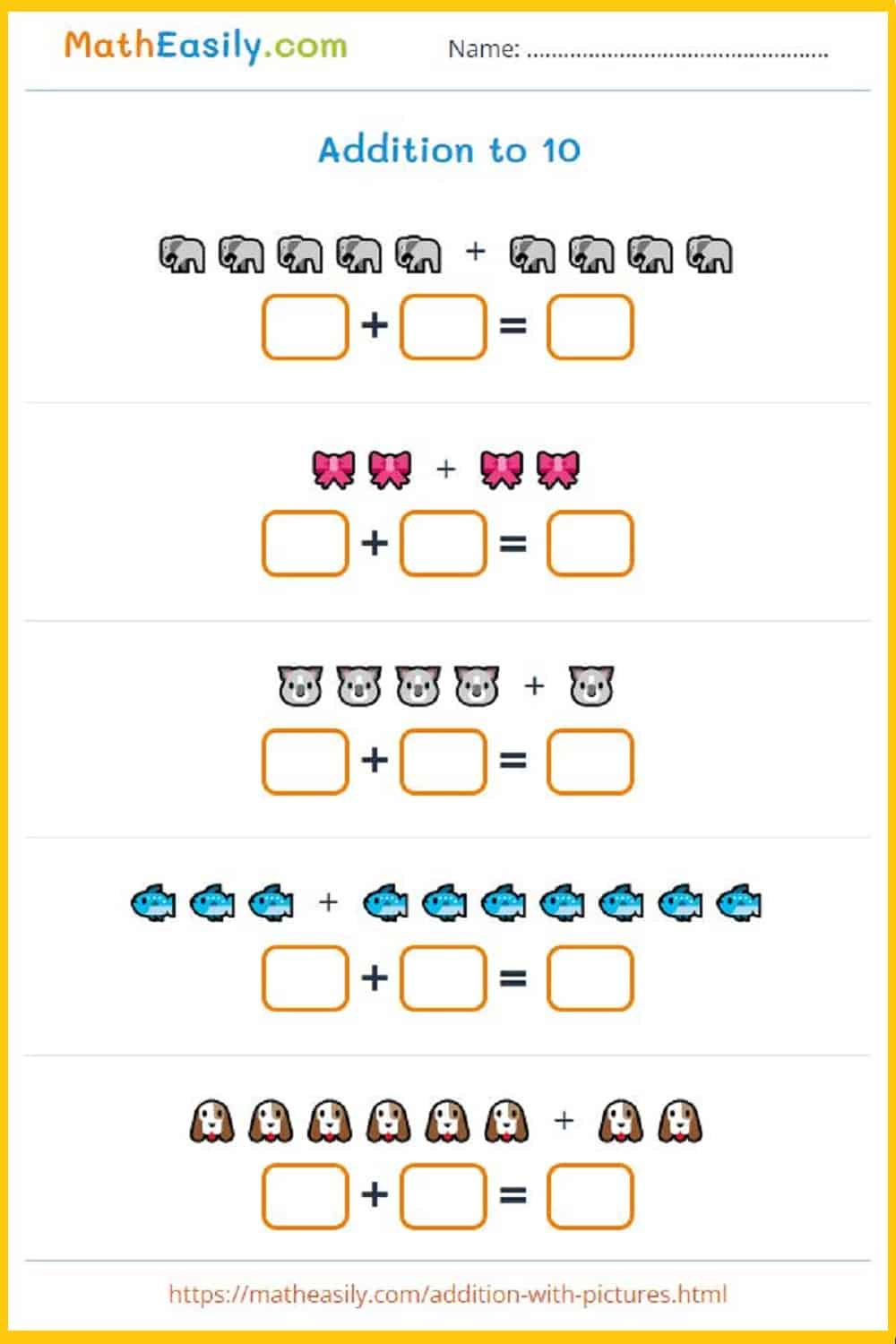Generate a new PDF

The puzzles are generated automatically, so you get a new one every time.Generate a new PDF

### Numbers before and after

Fill in the missing numbers 1-100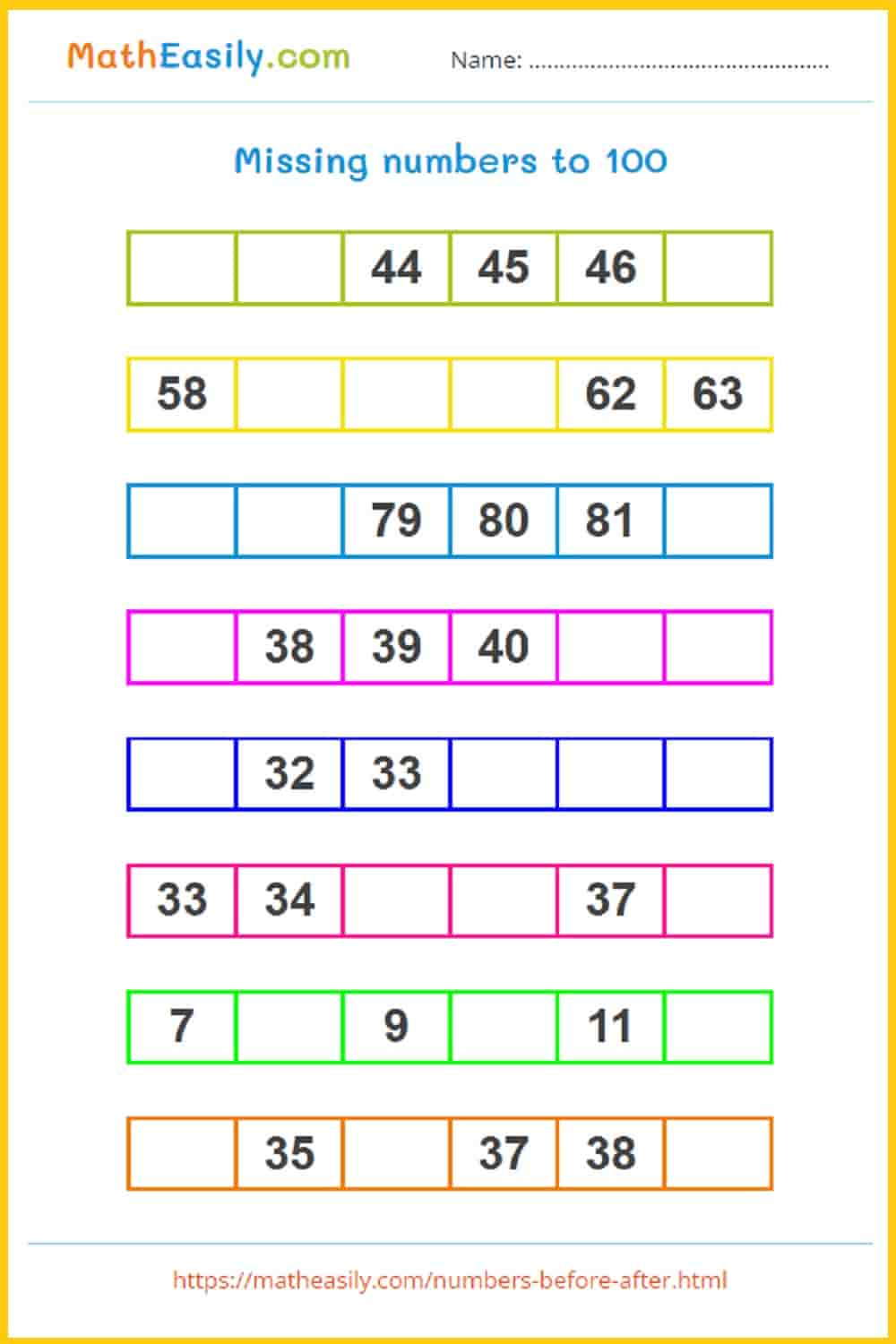Generate a new PDF

Addition BINGO 1-20: worksheets in PDF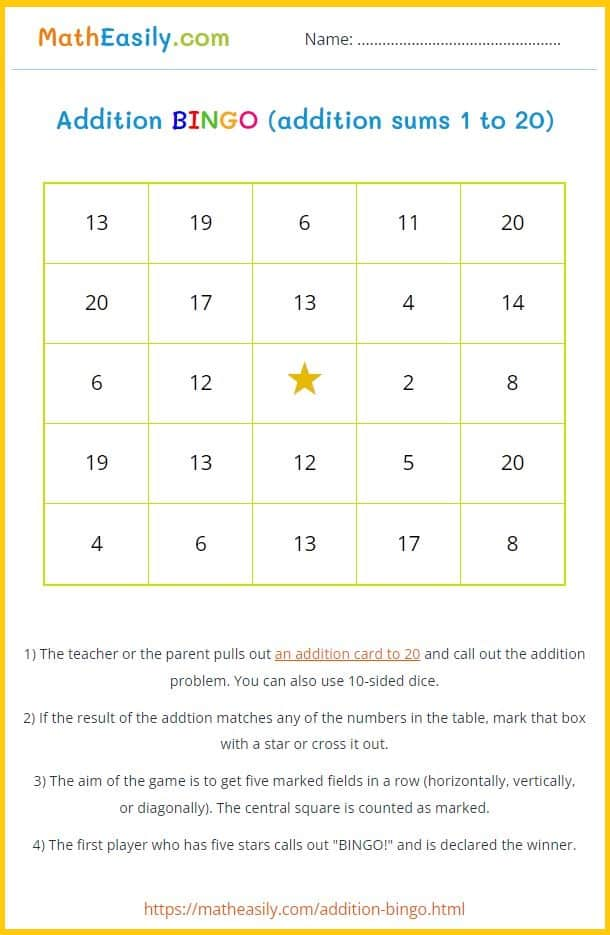Generate a new PDF

Most of these 1st grade math worksheets are generated automatically, so every time you get a new worksheet. So, if there is written ´Generate a new PDF´ under the worksheet, you can download as many different math worksheets for 1st graders as you want.

### School schedule

School timetable template in PDF and EXCEL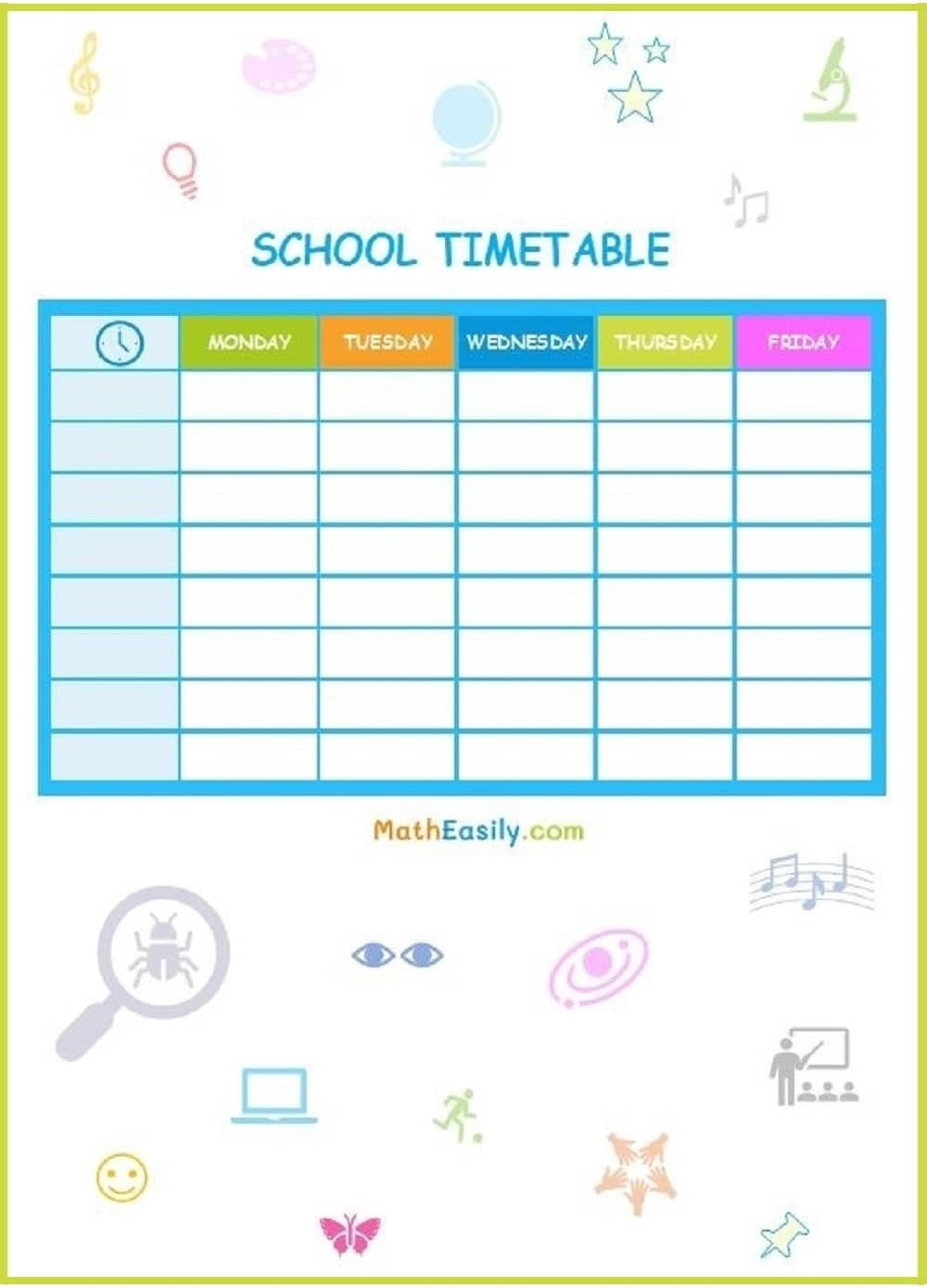### Counting games

Count pictures and write the number 1-10.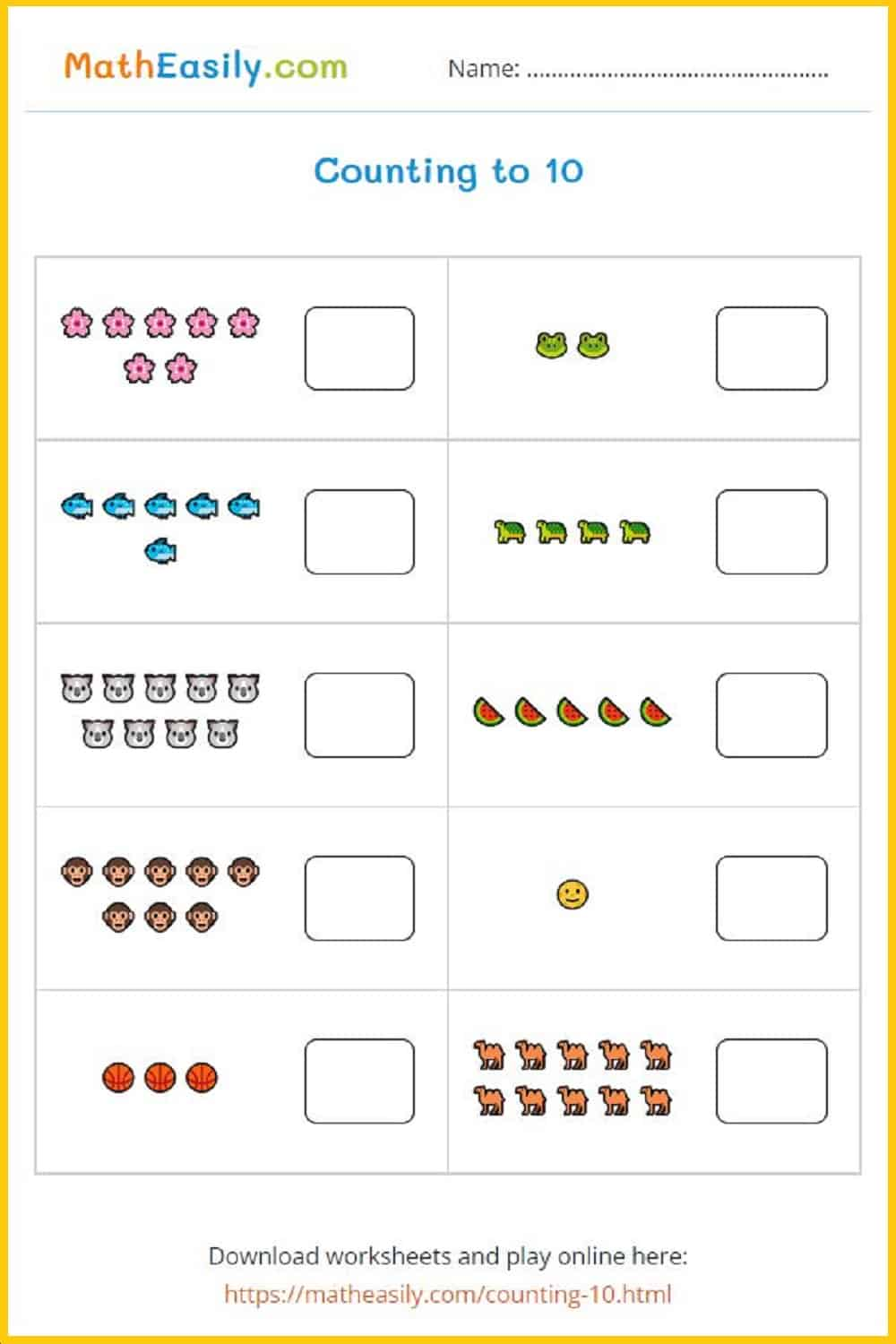Generate a new PDF

### Counting games

Counting games to 20. Generate a new worksheet.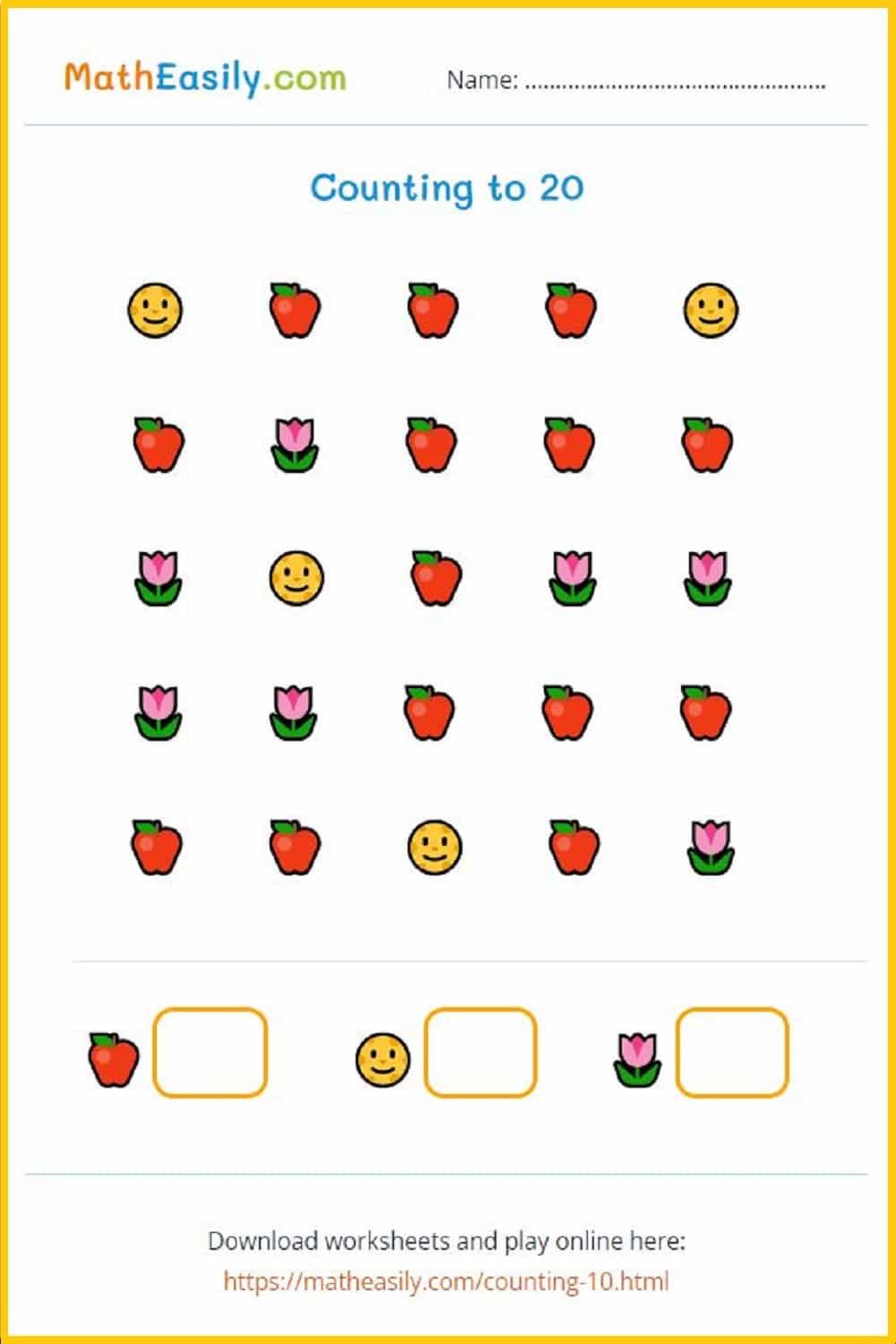Generate a new PDF

### Counting to 20

Count pictures and write the number 1-20.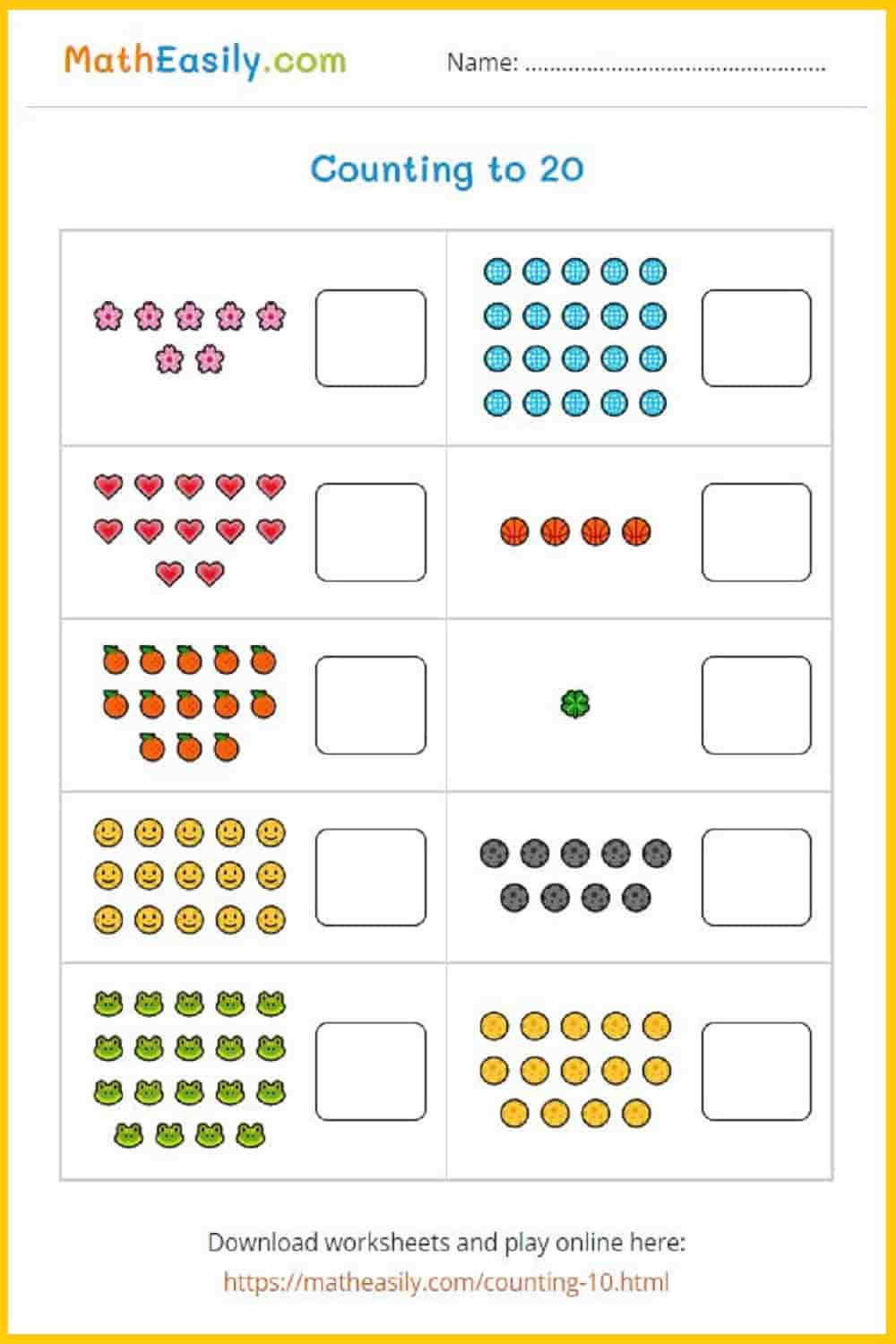Generate a new PDF

### 3D Shapes Games

3D shapes chart in PDF. Easy to print on A3 or A4 paper.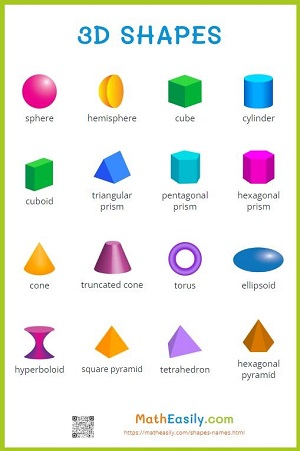### Shapes names### 2D Shapes games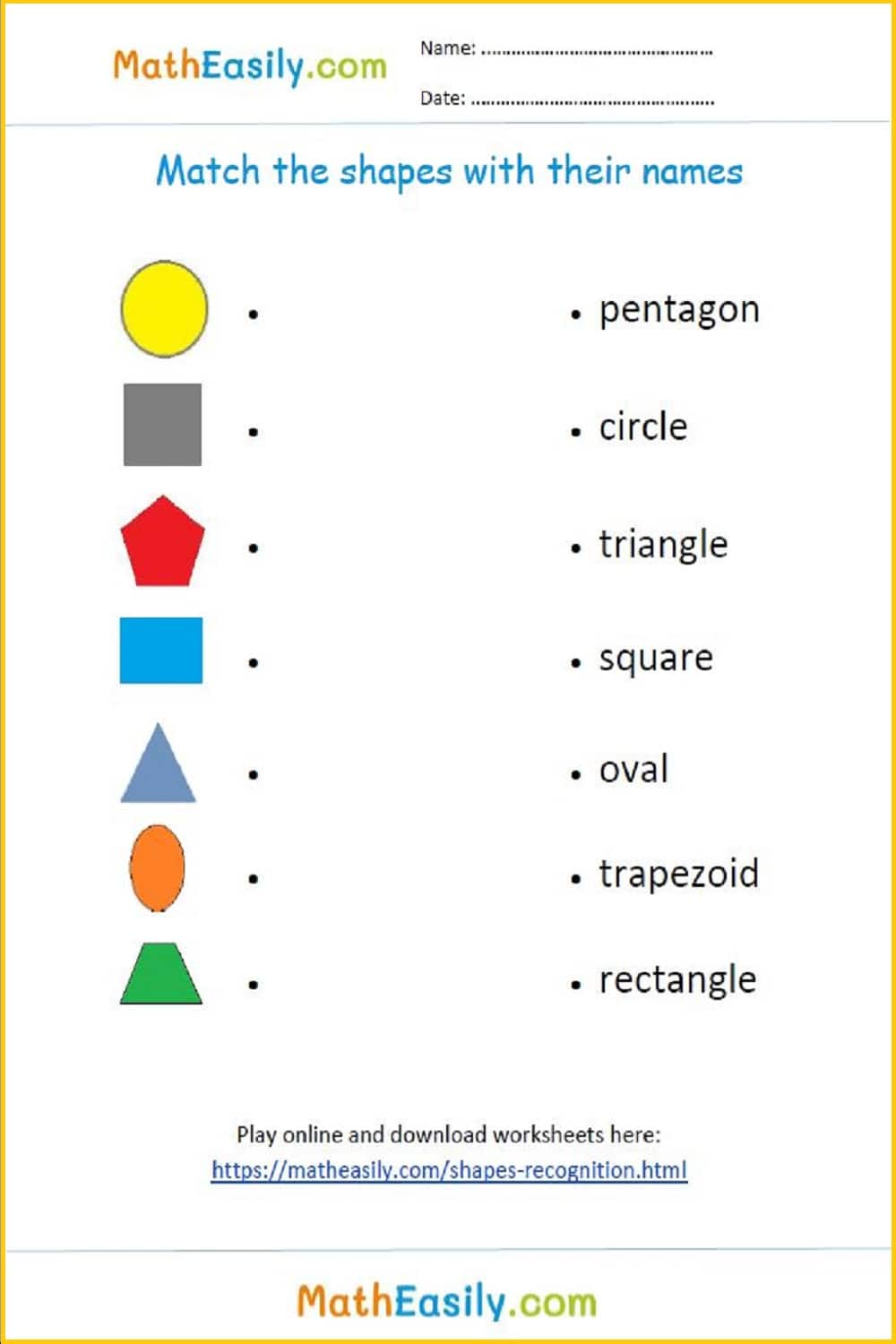### 2D Shapes recognition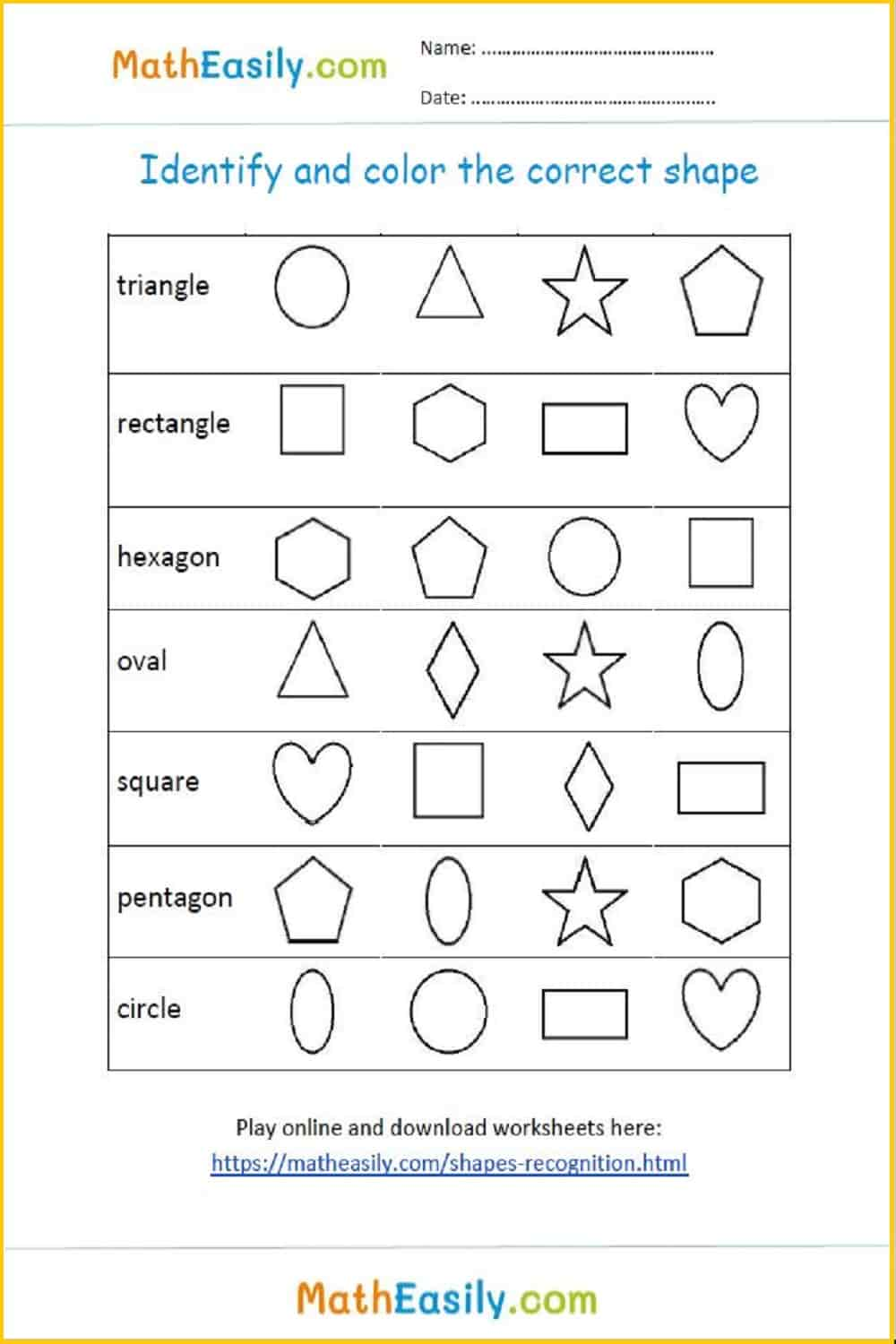### 100 Math Puzzles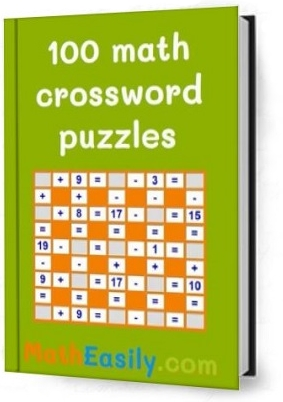Look inside here

### Missing numbers to 20

Missing numbers for kindergarten 1-20.Generate a new PDF

### What comes after

Fill in the missing numbers what comes after 1-50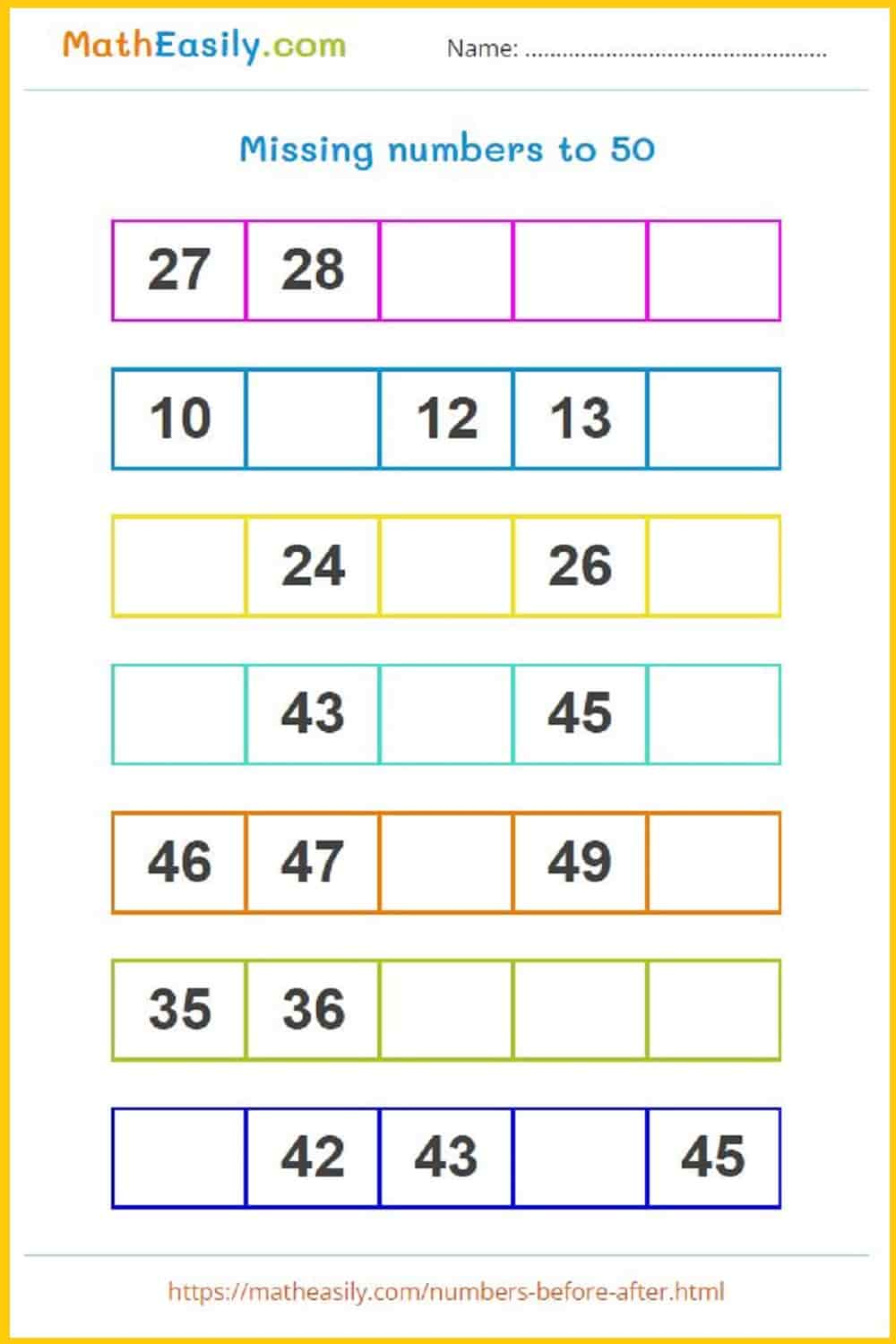Generate a new PDF

### What comes after

Fill in the missing numbers what comes after 1-50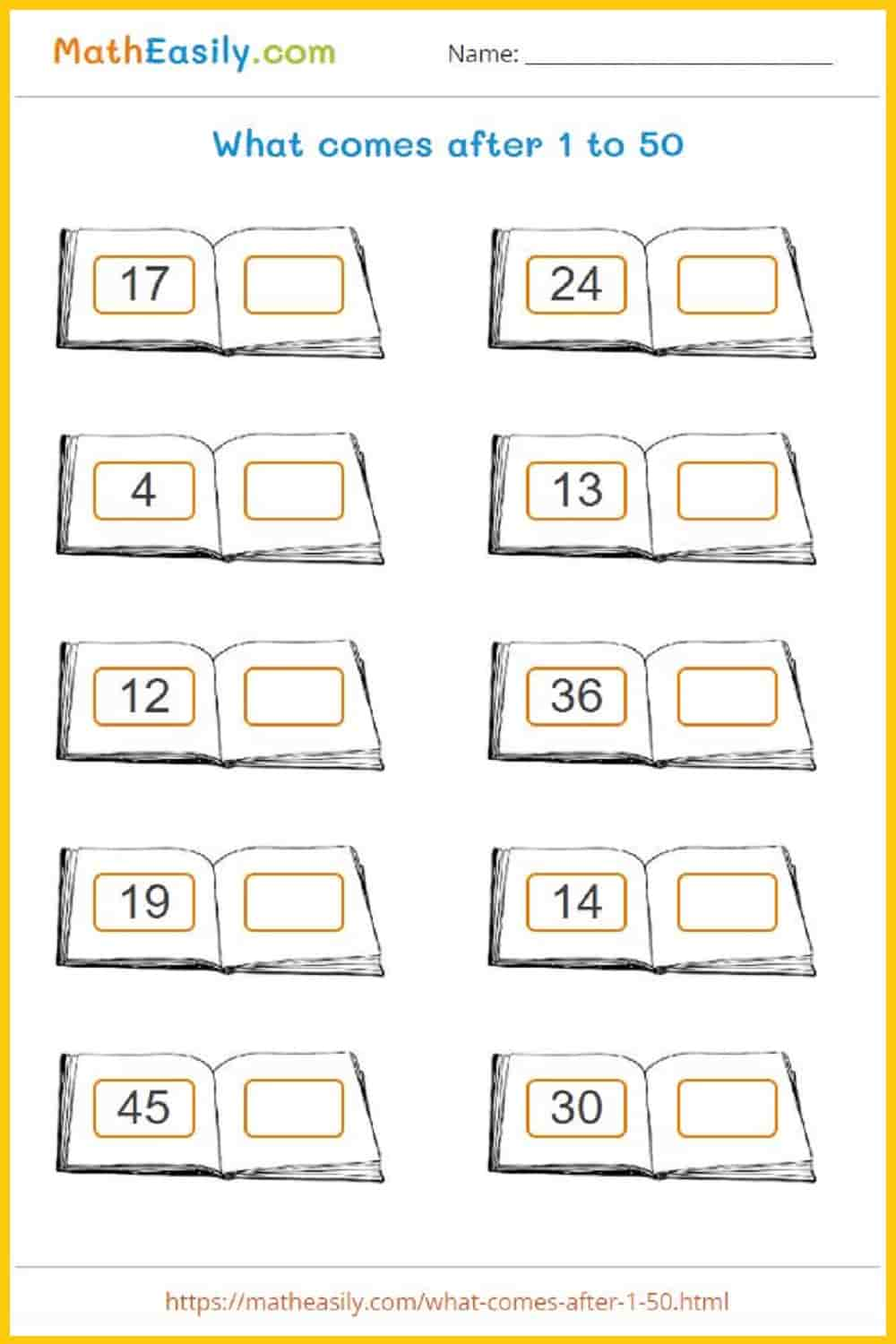Generate a new PDF

### After numbers to 50

Fill in the missing numbers what comes after 1-50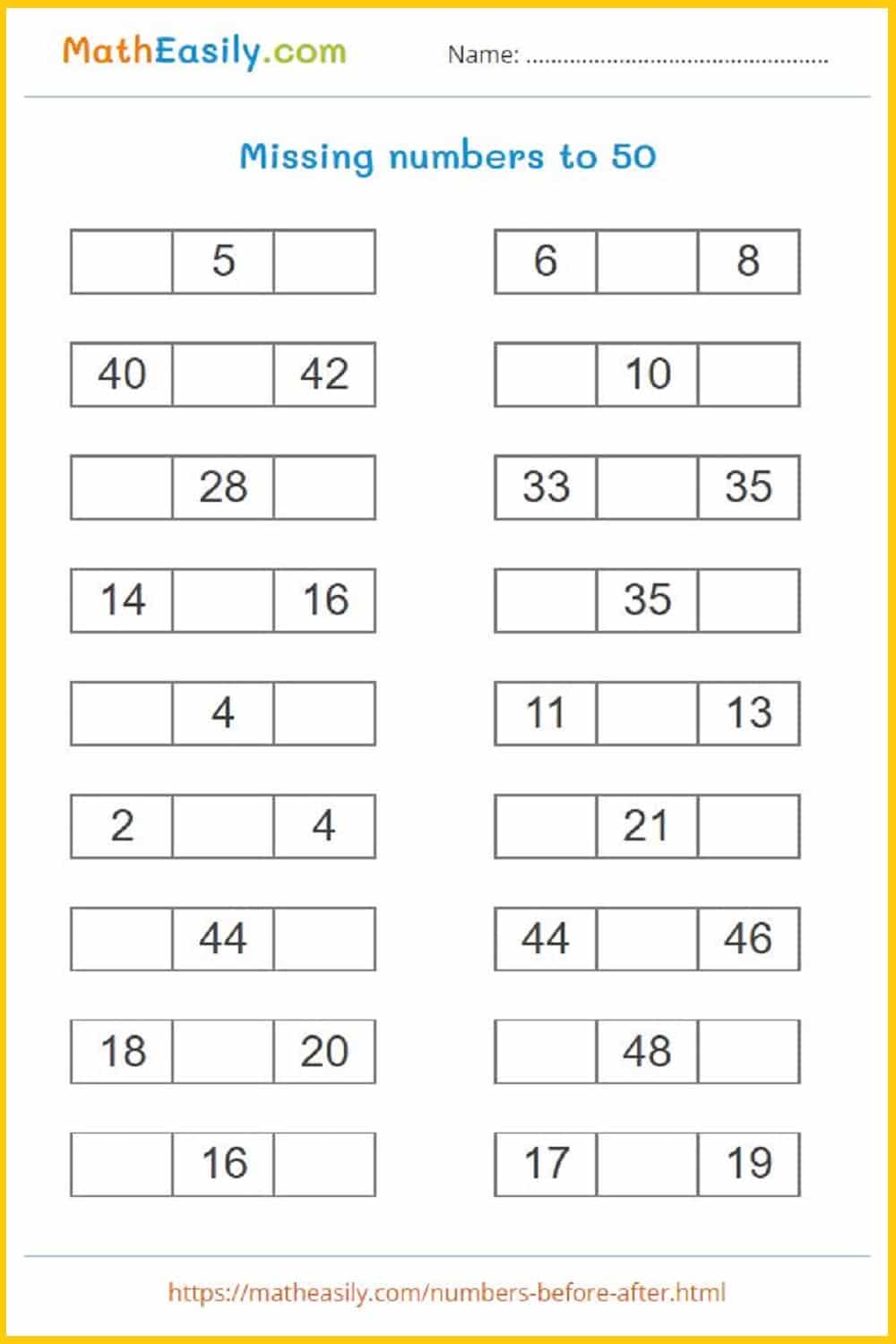Generate a new PDF

### After numbers to 1-50

Fill in the missing numbers what comes after 1-50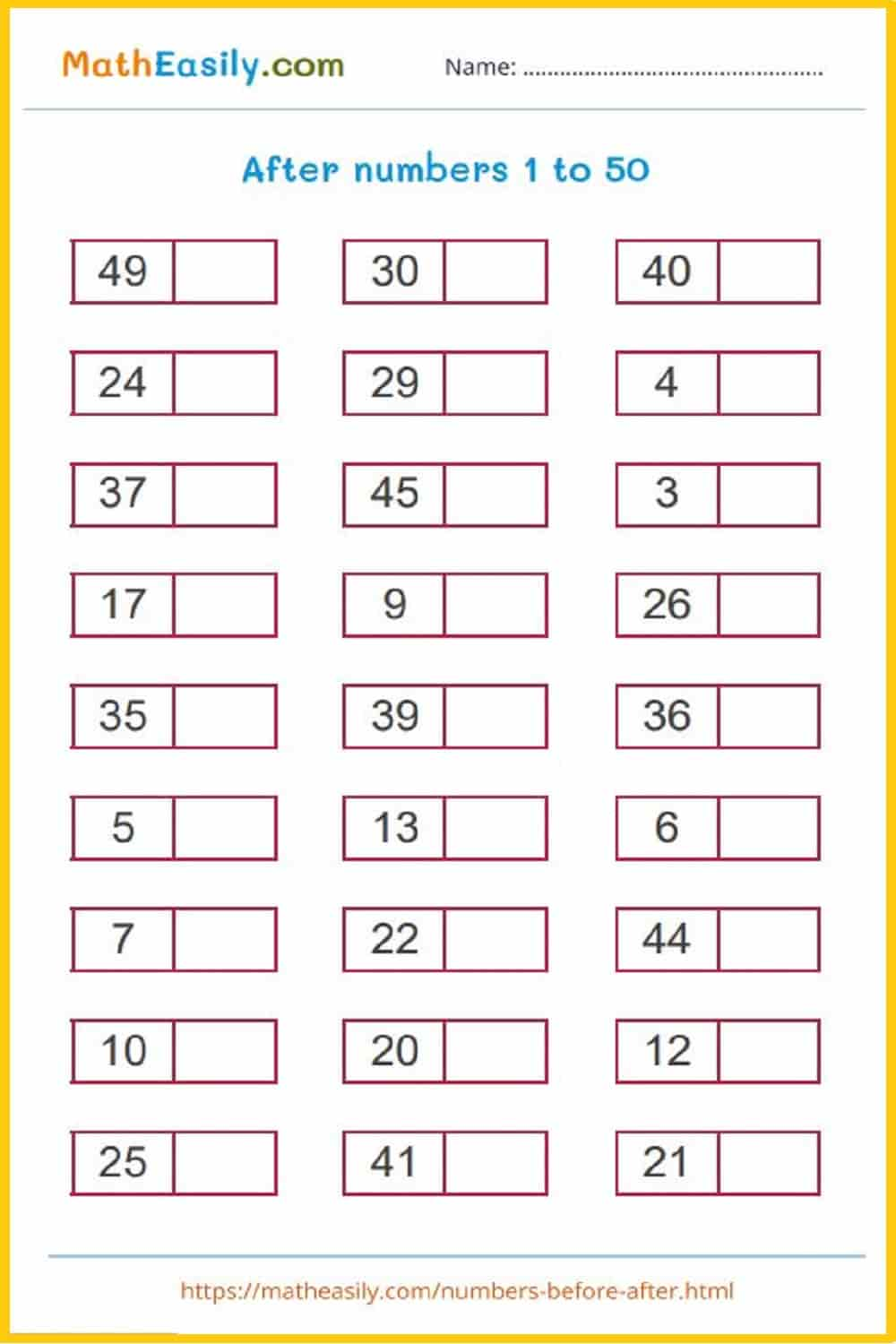Generate a new PDF

### Number chart

Fill in the missing numbers to 50 in the number chart.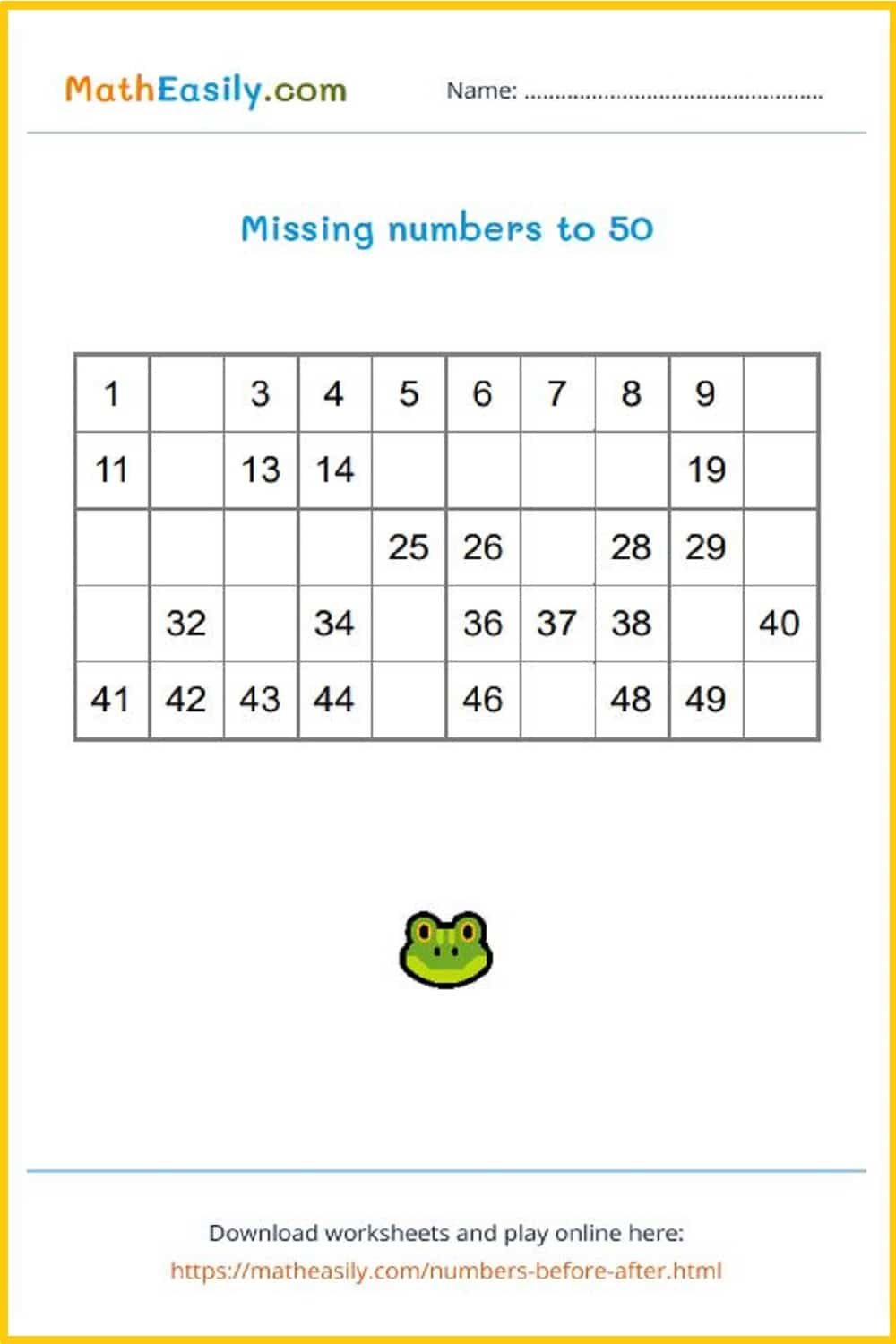Generate a new PDF

### After numbers 1 - 50

Fill in the missing numbers what comes after 1-50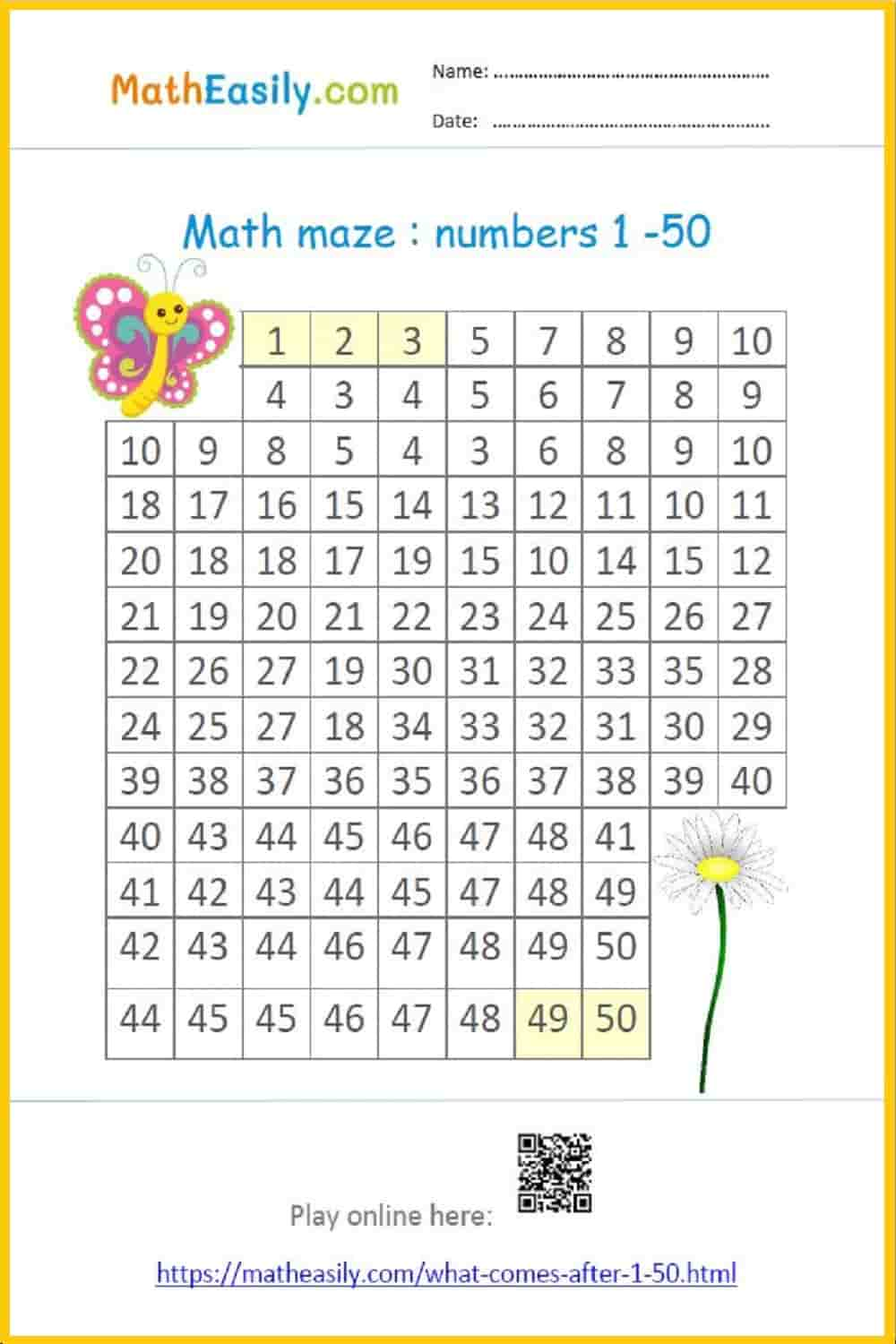### Numbers 1 - 50

Connect the dots - after numbers 1-50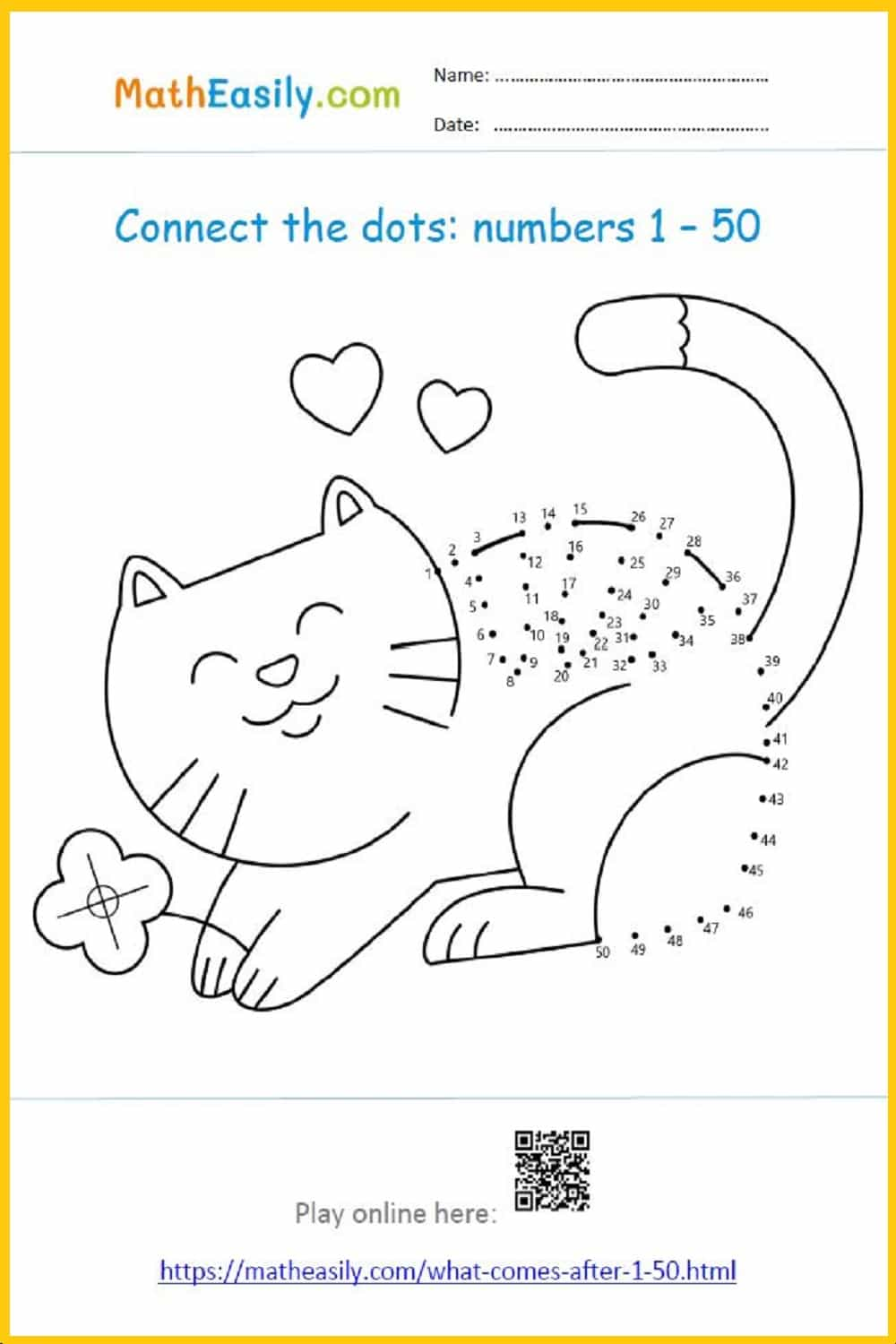### Number chart 1-100

Fill in the missing numbers in the hundreds chart.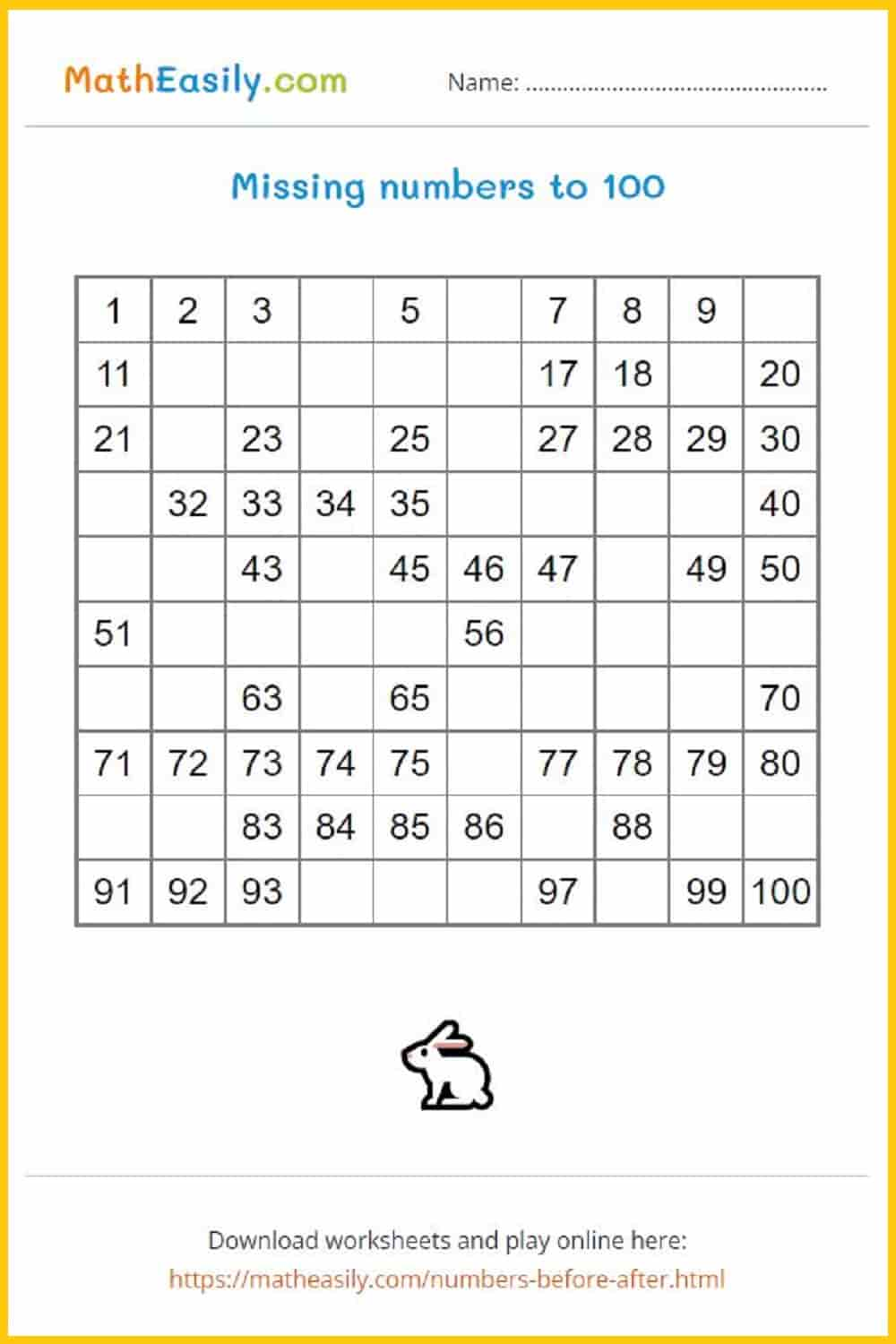Generate a new PDF

### After numbers to 100

Fill in the number what comes after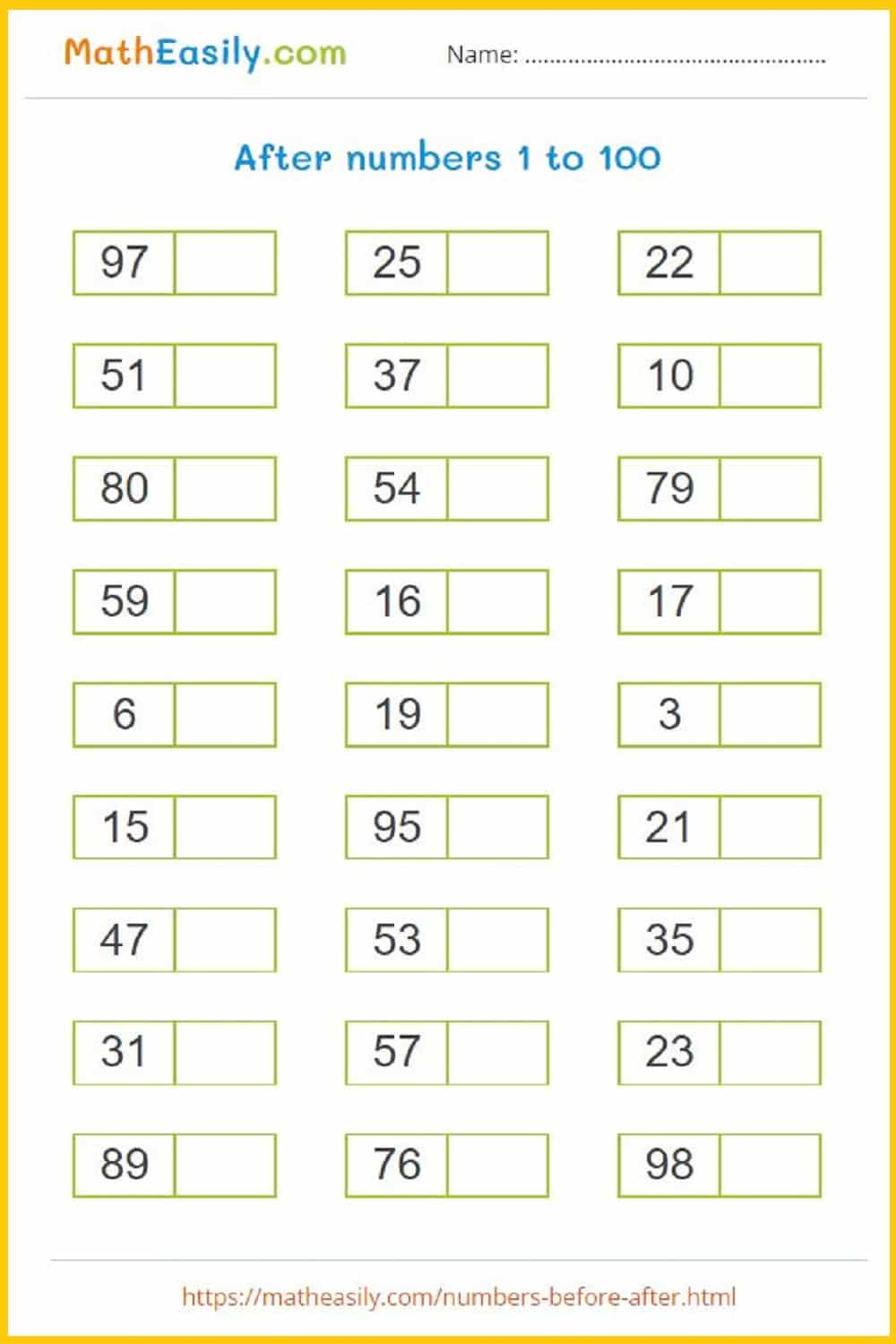Generate a new PDF

### Missing numbers to 100

Fill in the numbers before and after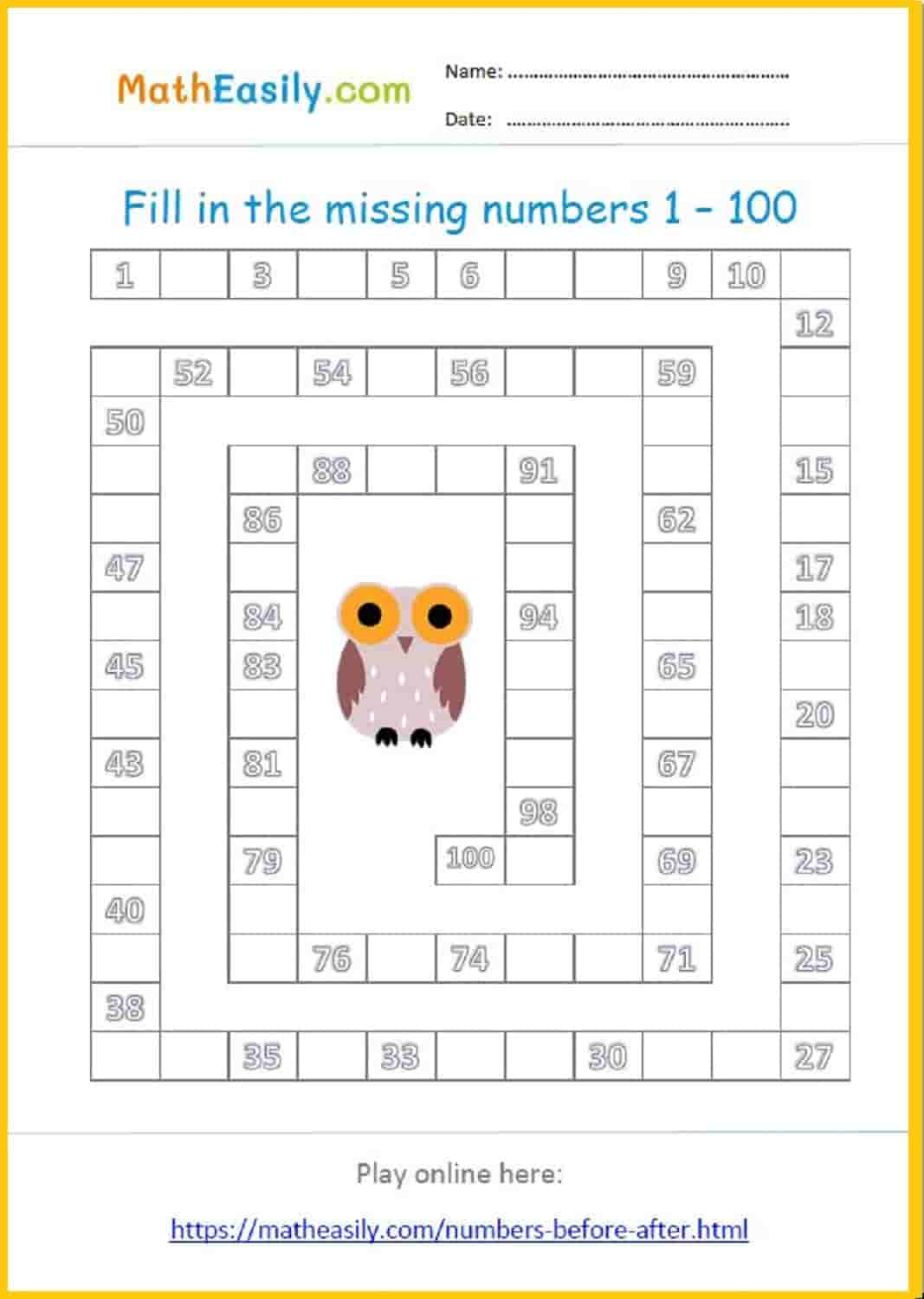### Missing numbers to 100

Fill in the missing numbers before, between and after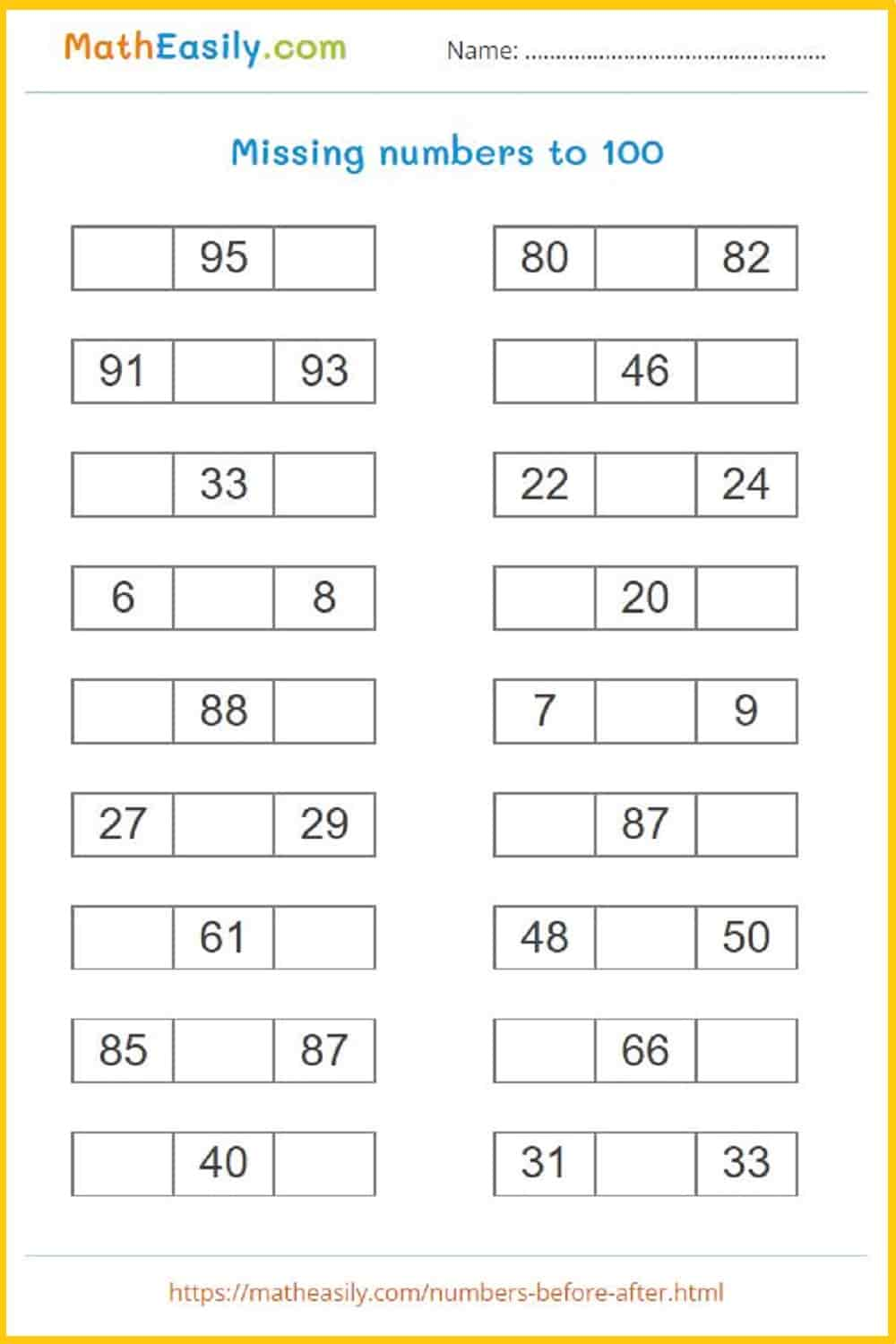Generate a new PDF

### Numbers 1 to 100

Connect the dots - after numbers 1-100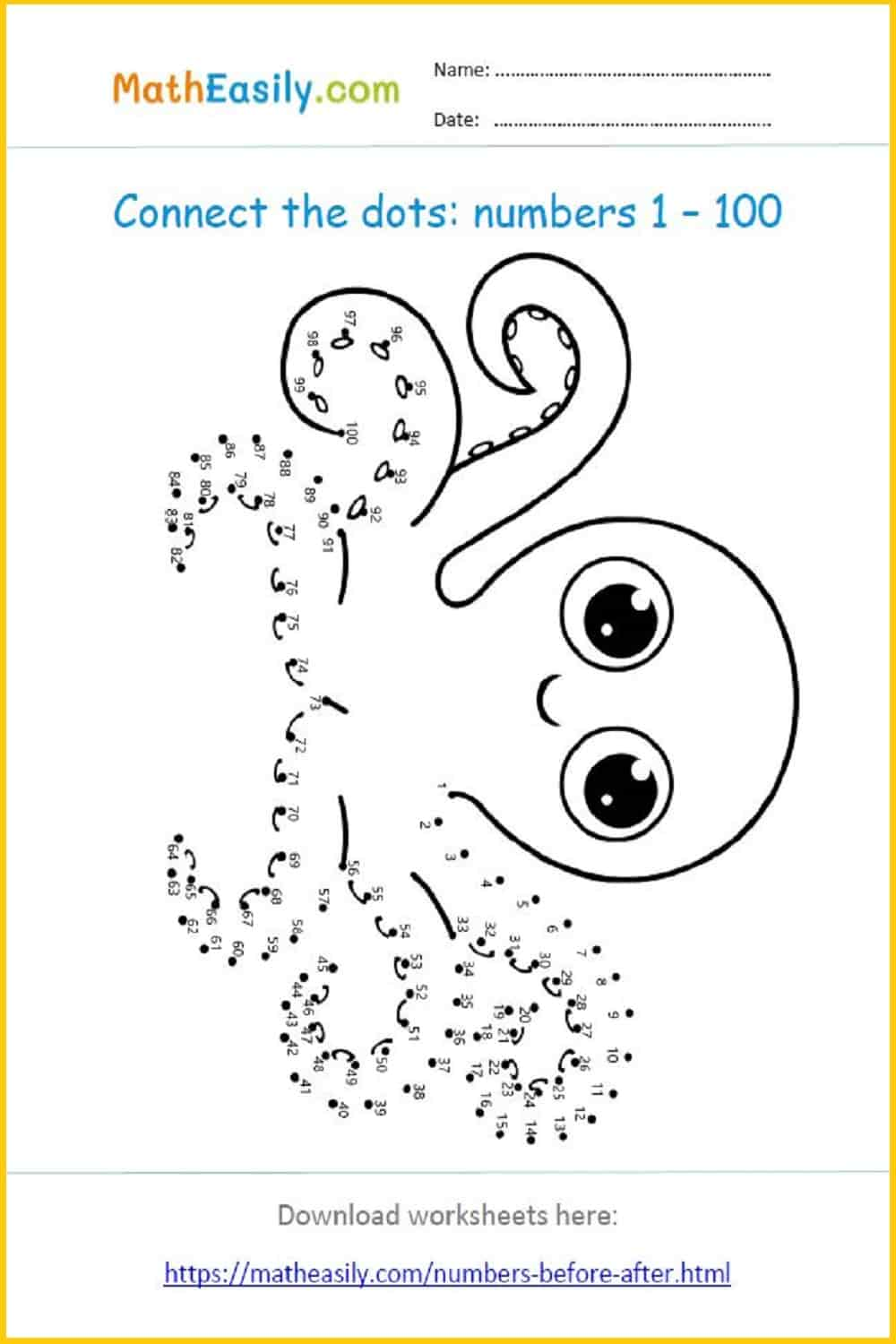### Missing numbers to 100

Fill in the missing numbers before, between and after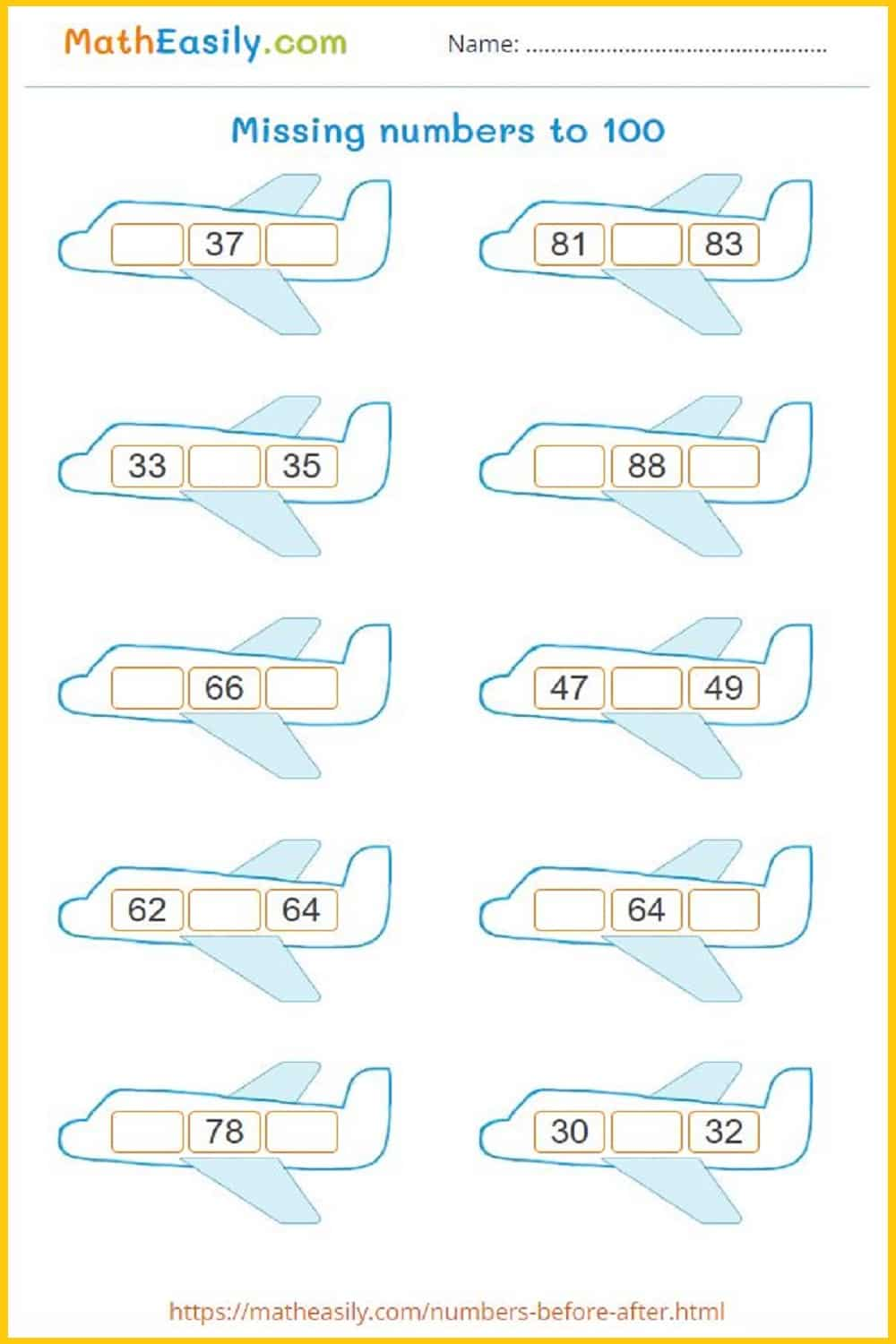Generate a new PDF

### Numbers to 1000

Fill in the numbers before, after or between to 1000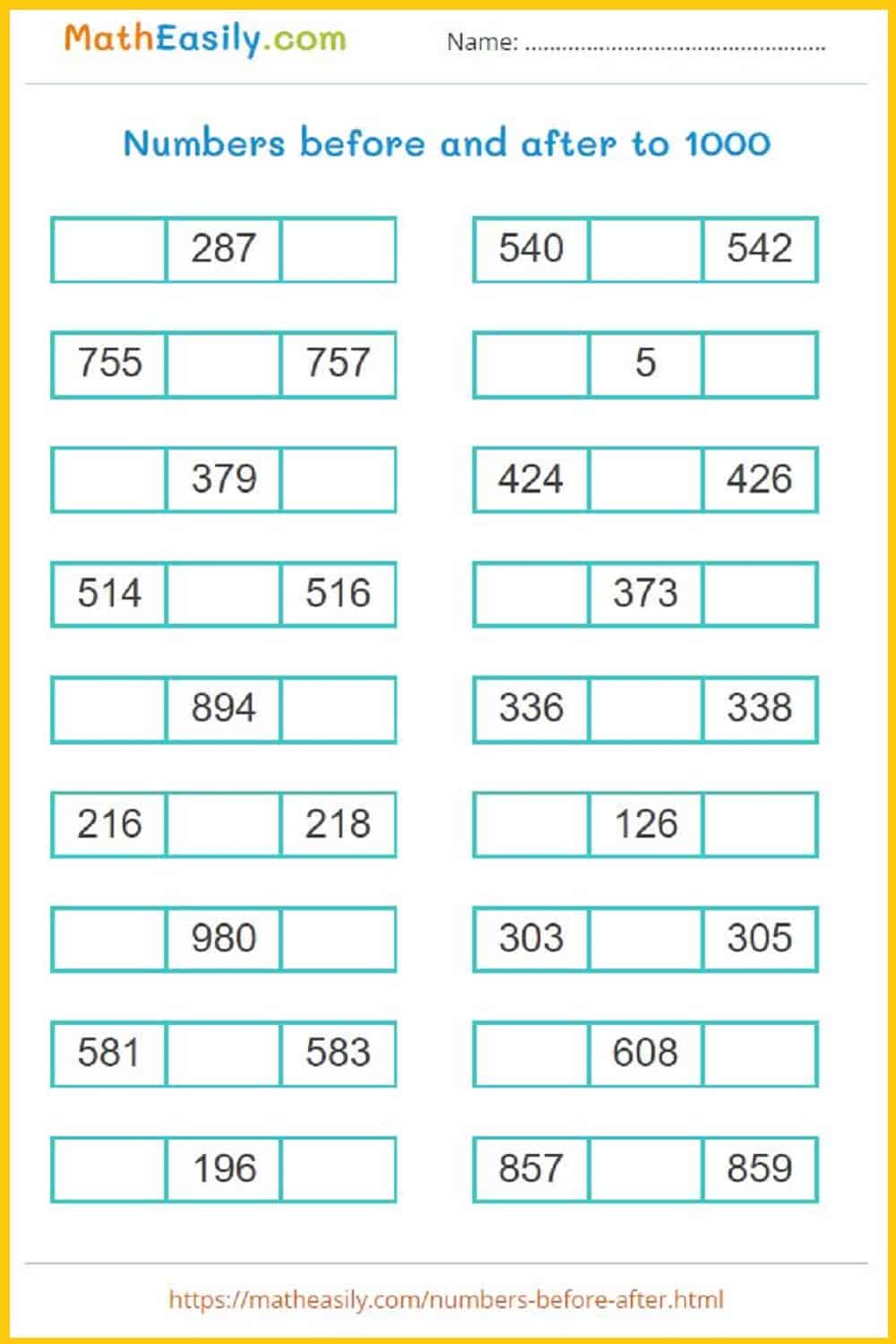Generate a new PDF

### Comparing numbers

Comparing numbers to 10: generate a worksheet.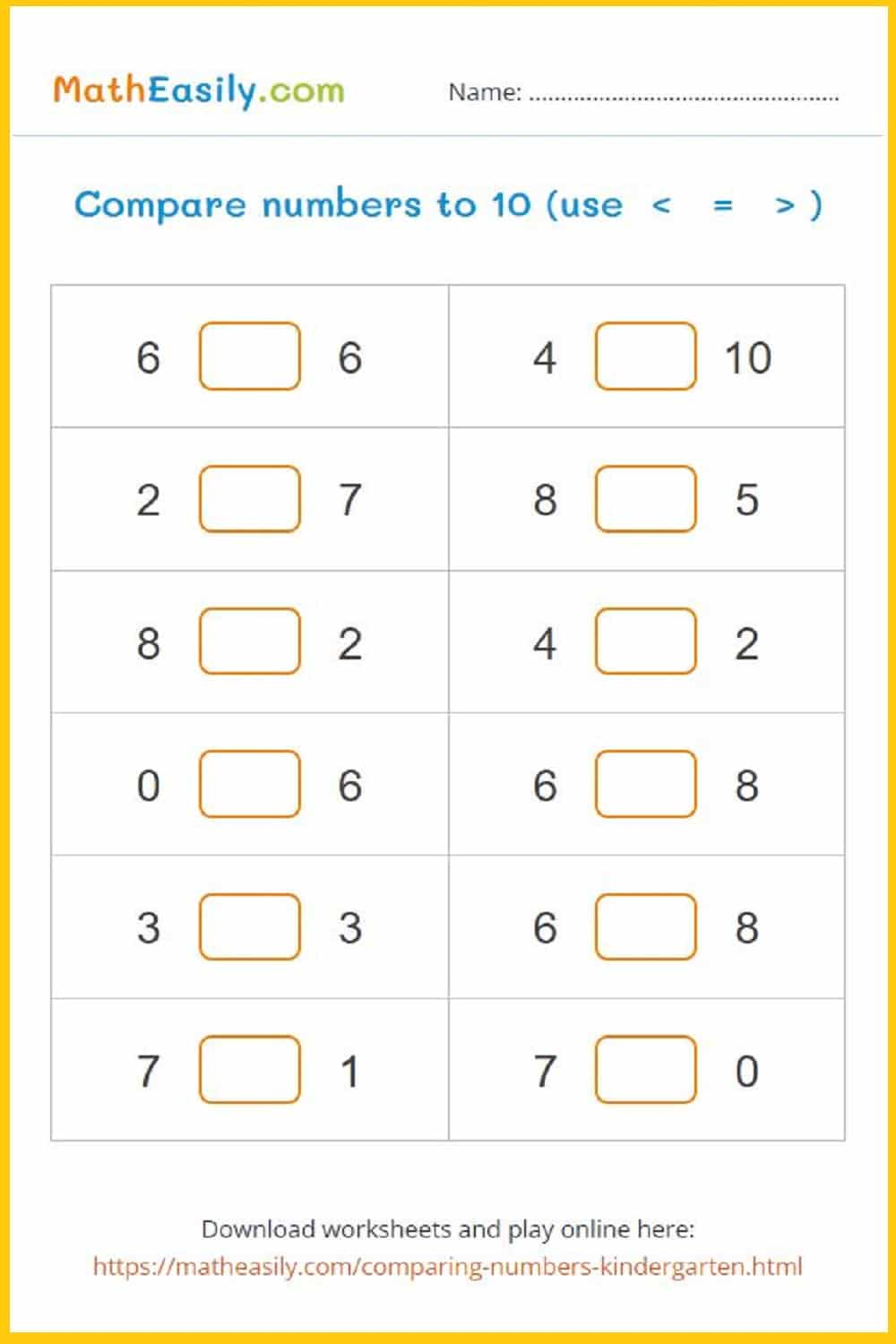Generate a new PDF

### Comparing numbers

Comparing numbers to 20: generate a worksheet.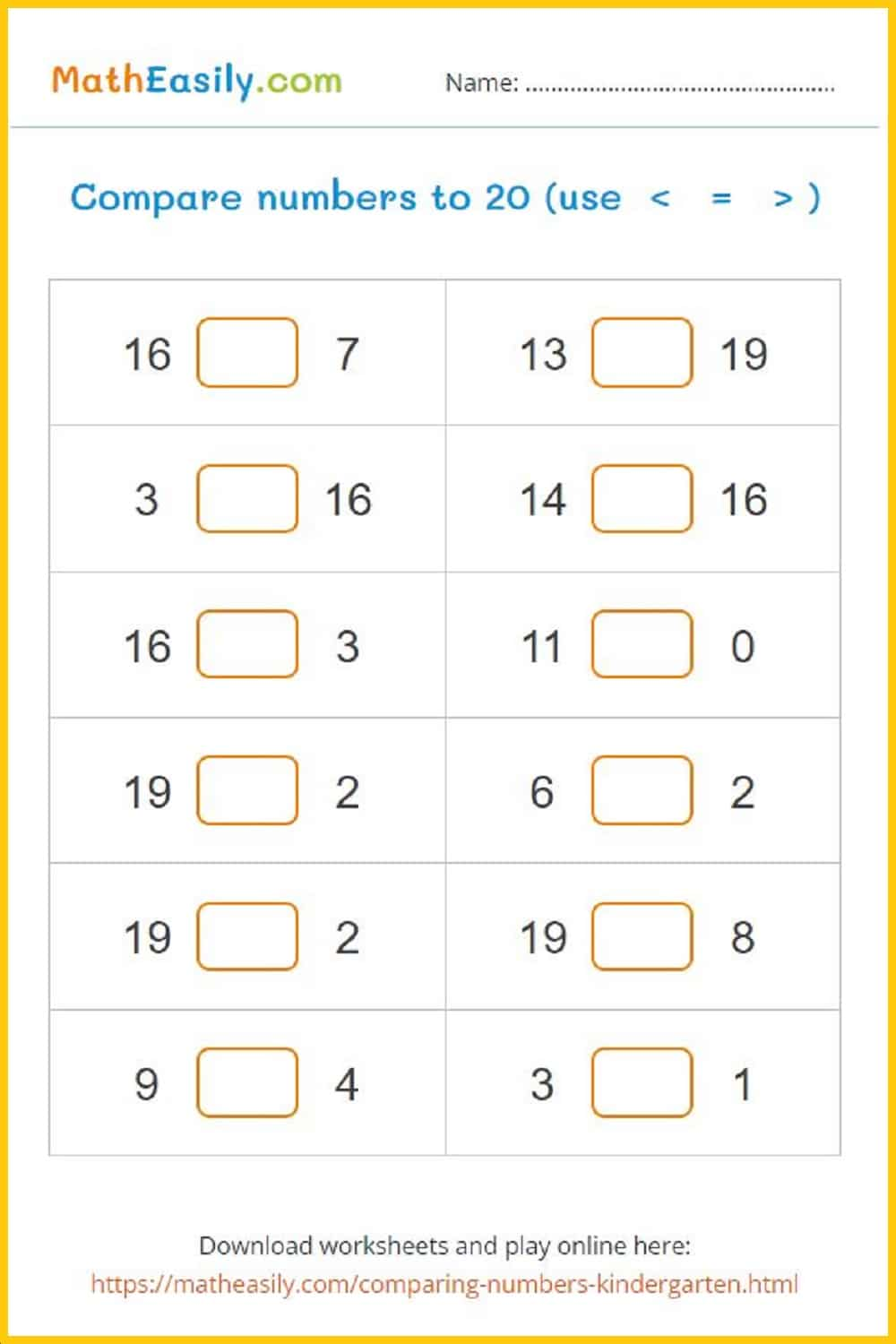Generate a new PDF

### Comparing numbers

Comparing numbers to 100: generate a worksheet.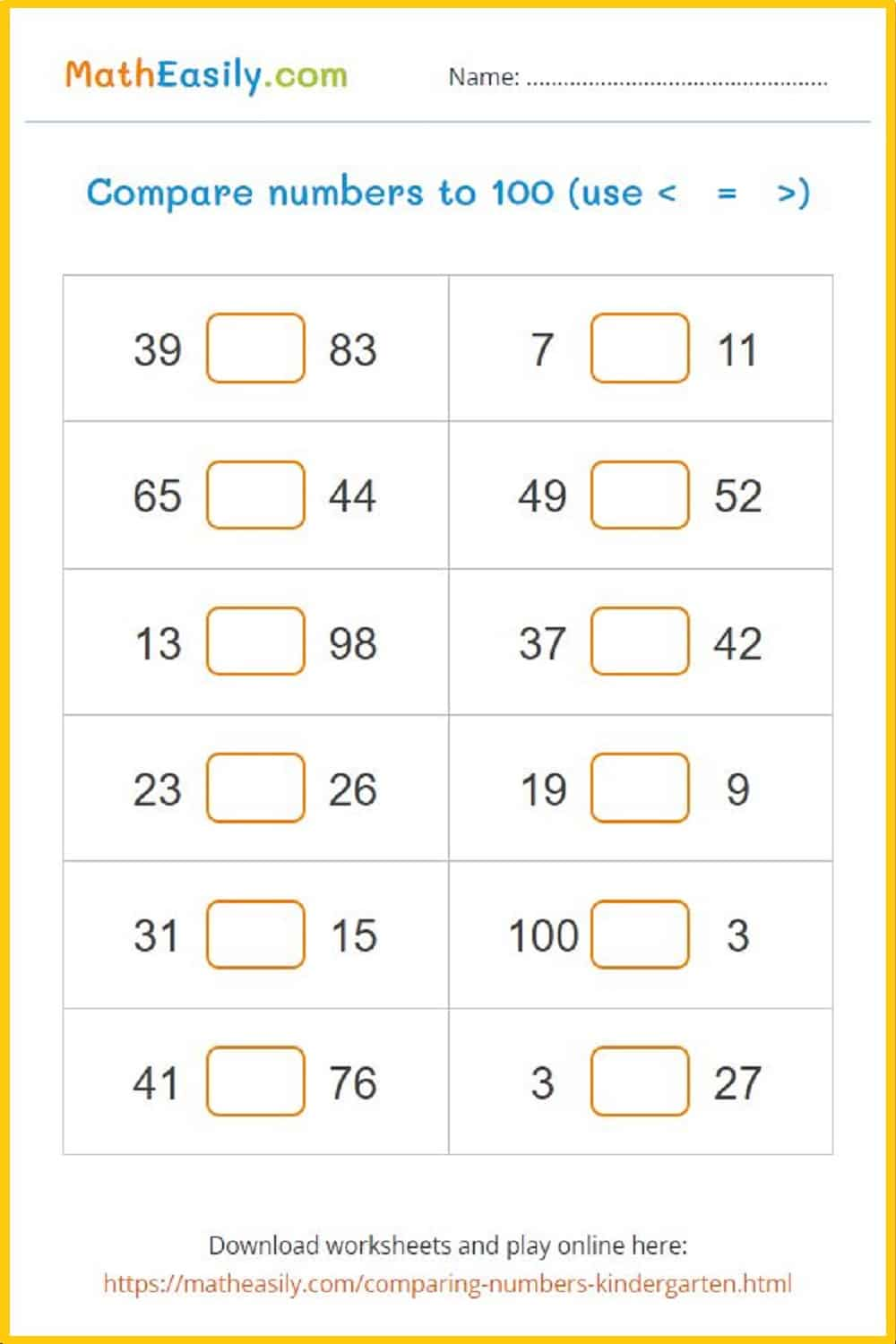Generate a new PDF

##### 100 Matchstick Math Puzzles

Printable math matchstick puzzles with answers in PDF. Move 1 stick to fix the equation.

US\$ 7.00

LOOK INSIDE

Dice game: single digit addition up to 20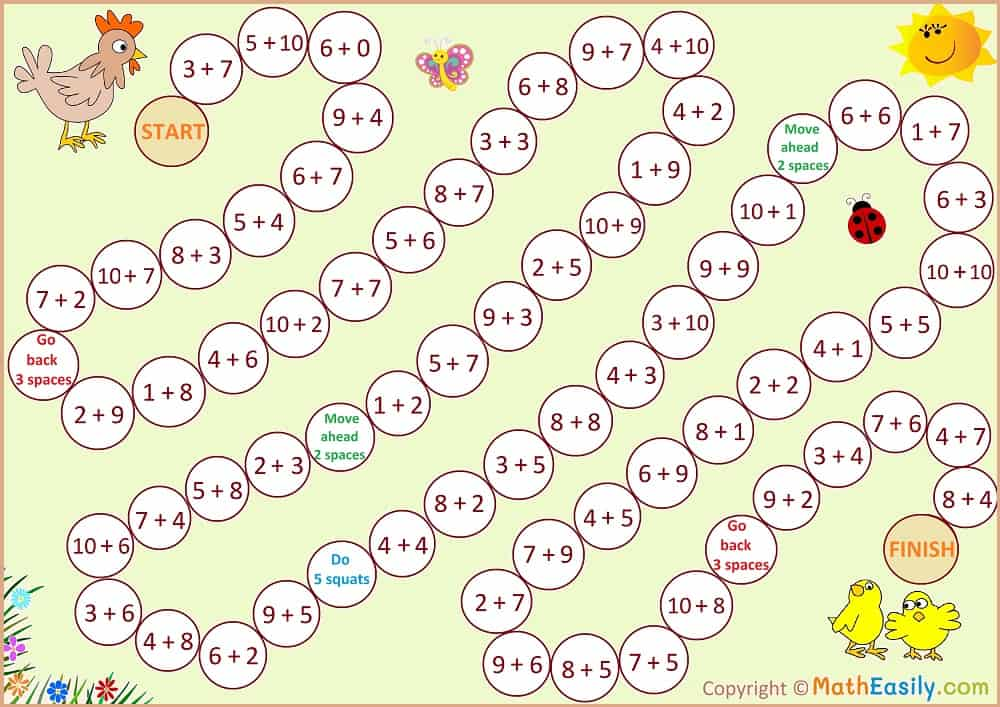Single digit addition to 10: worksheets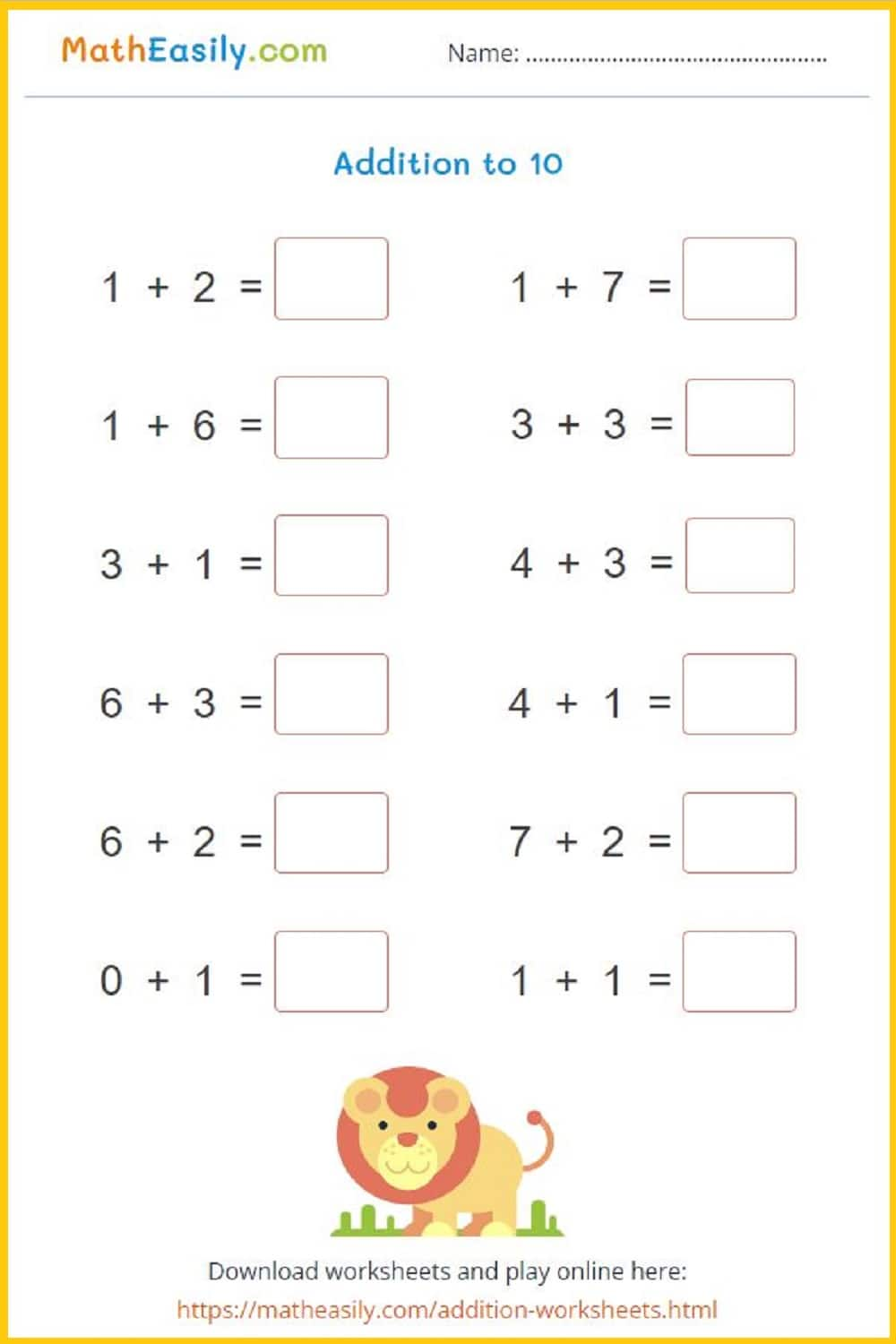Generate a new PDF

Addition up to 20 with help of picturesGenerate a new PDF

Addition BINGO 1-12: worksheets in PDFGenerate a new PDF

Dice game: single digit addition up to 12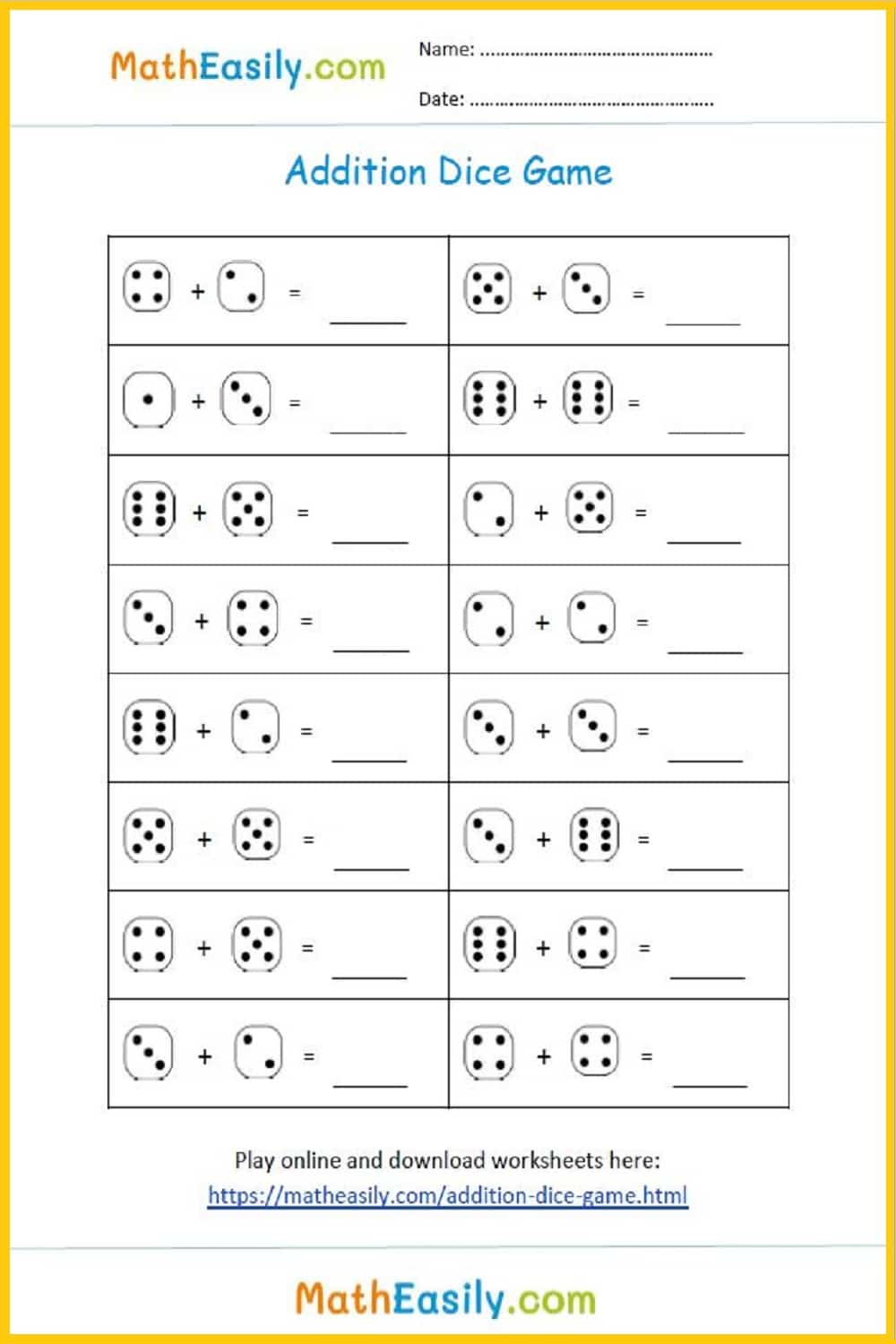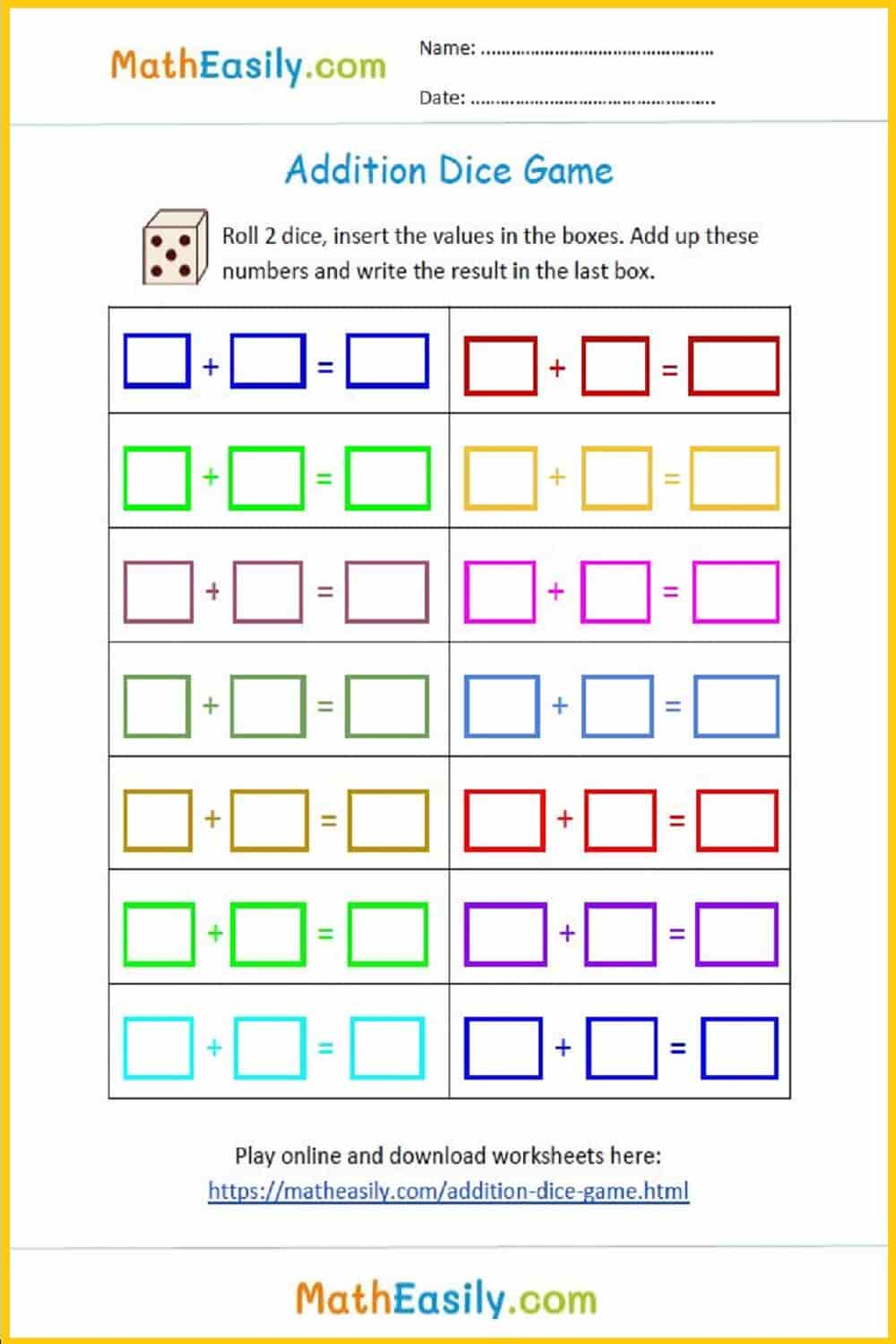Addition to 20: worksheets and games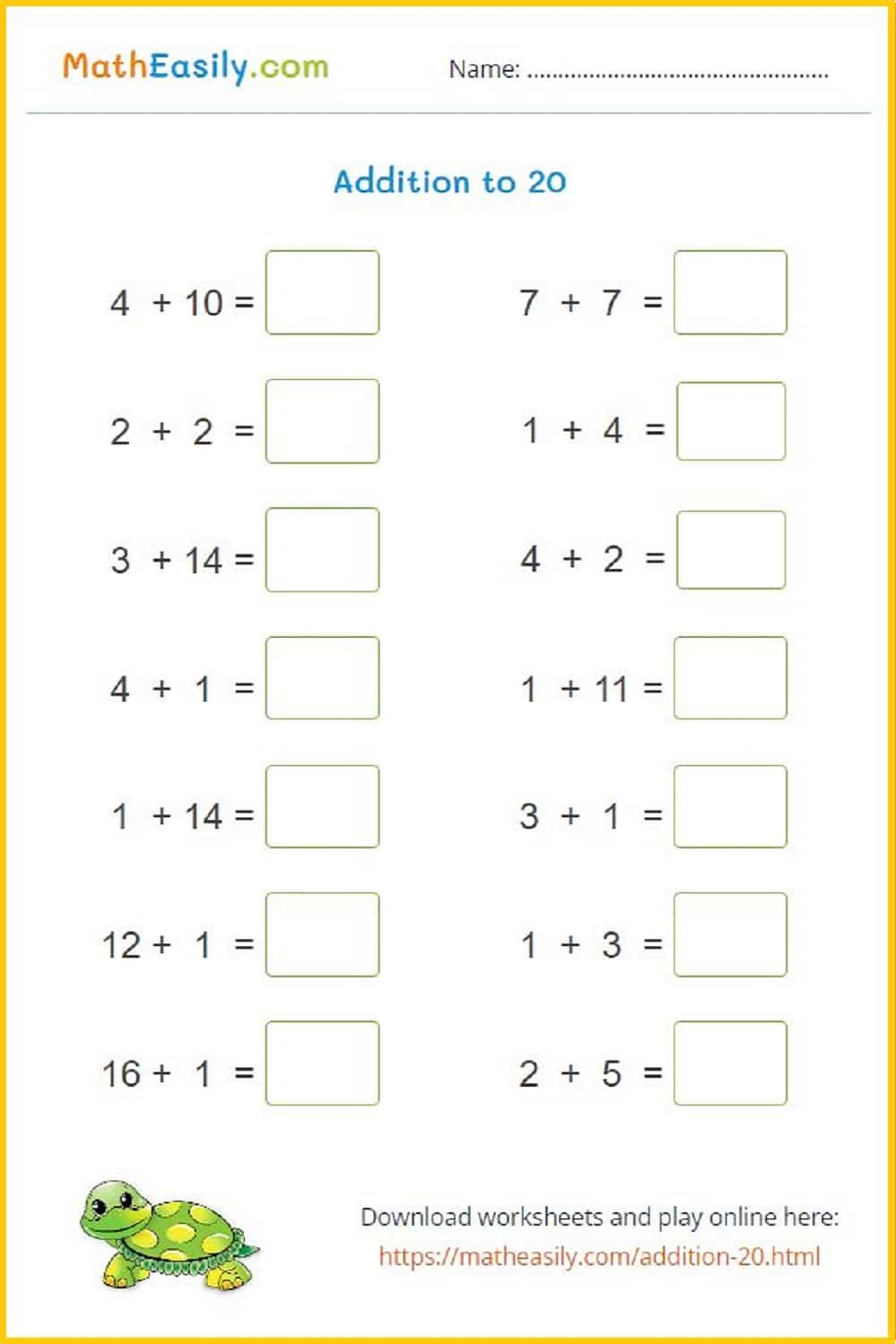Generate a new PDF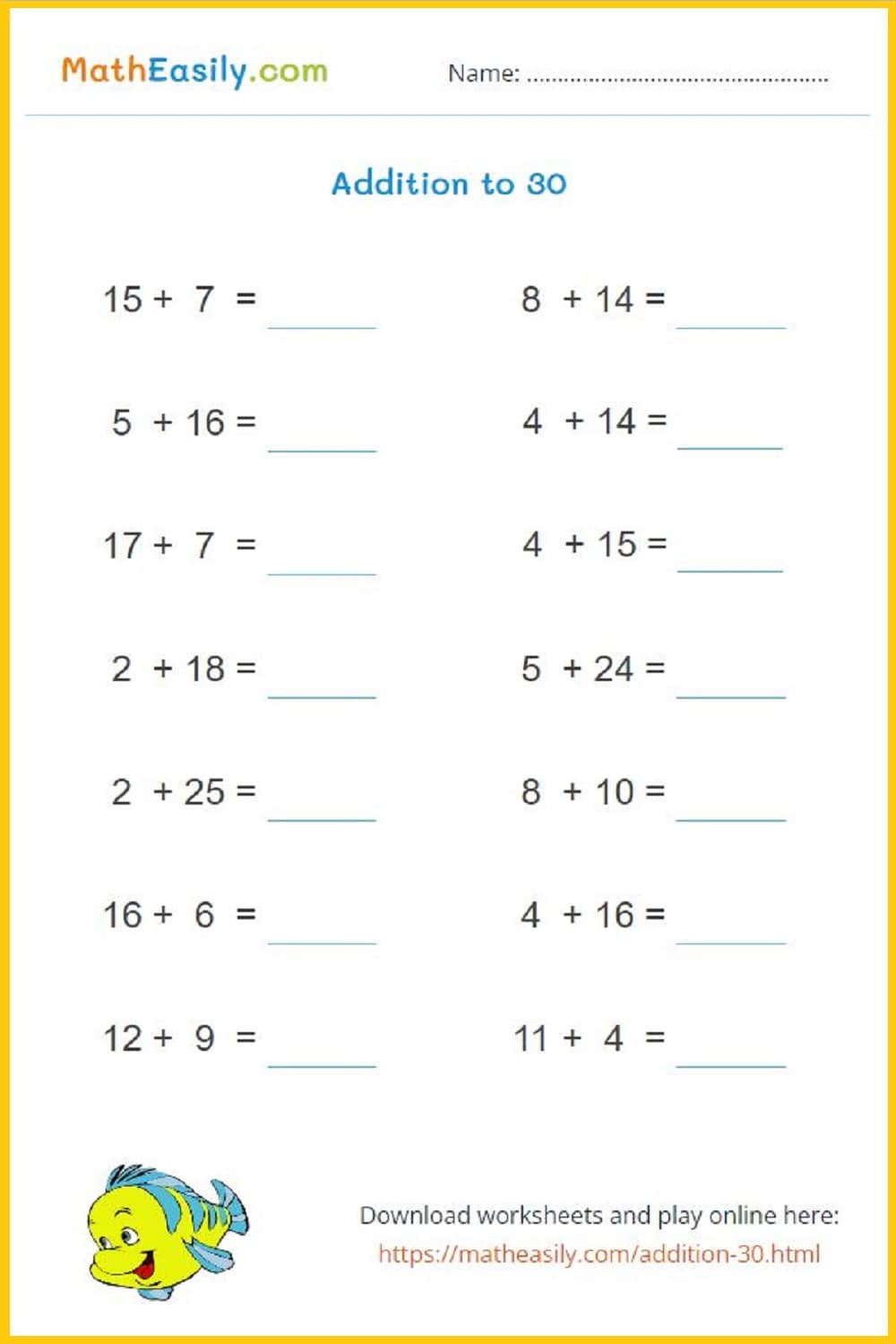Generate a new PDF

### 1st & 2nd Grade Math

Addition & Subtraction. Place Value, Regrouping and More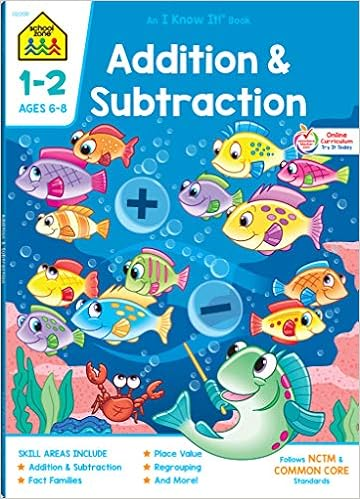Shop on Amazon

Fill in the addition pyramid puzzles up to 20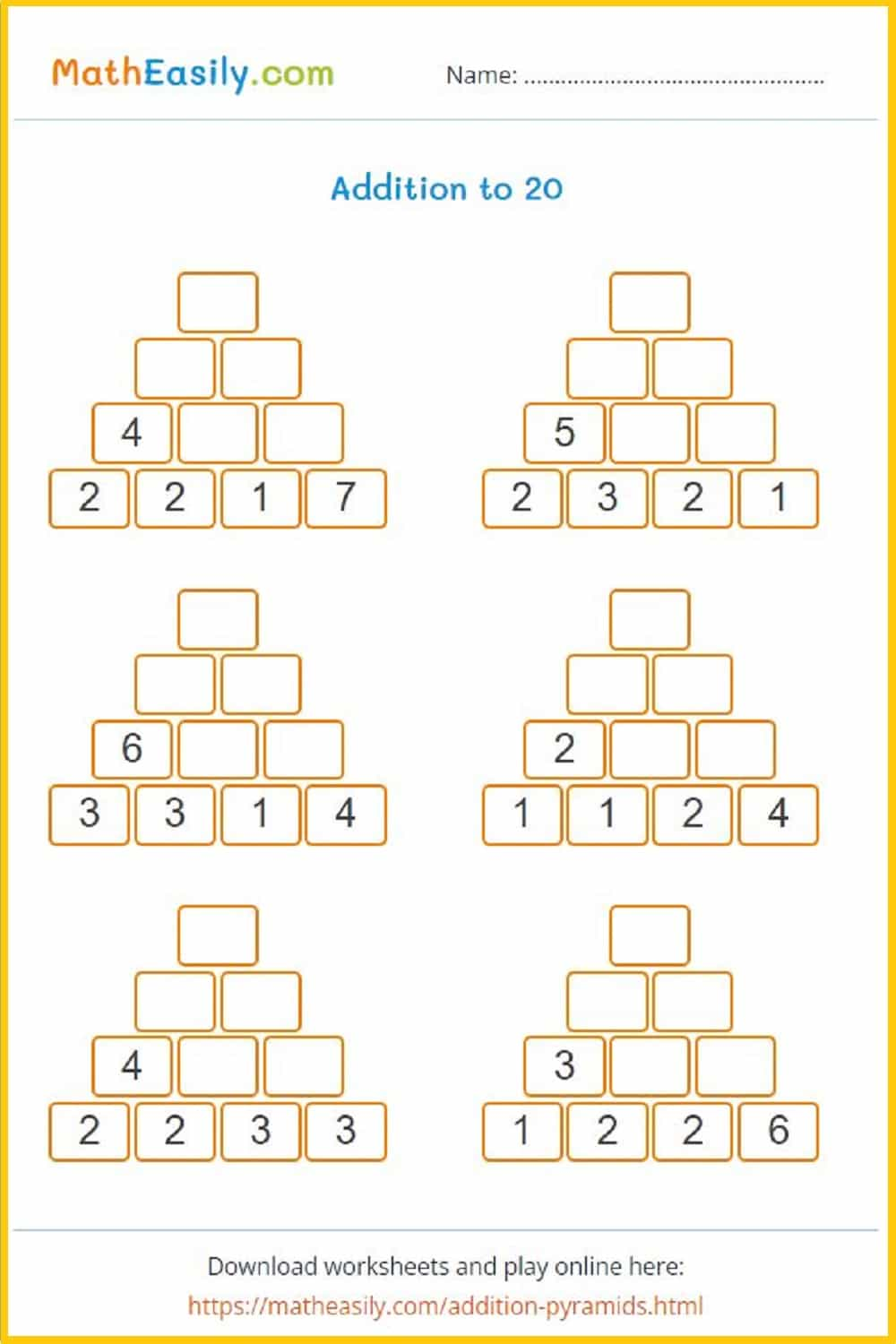Generate a new PDF

### Number pyramids

Fill in the addition pyramid puzzles up to 30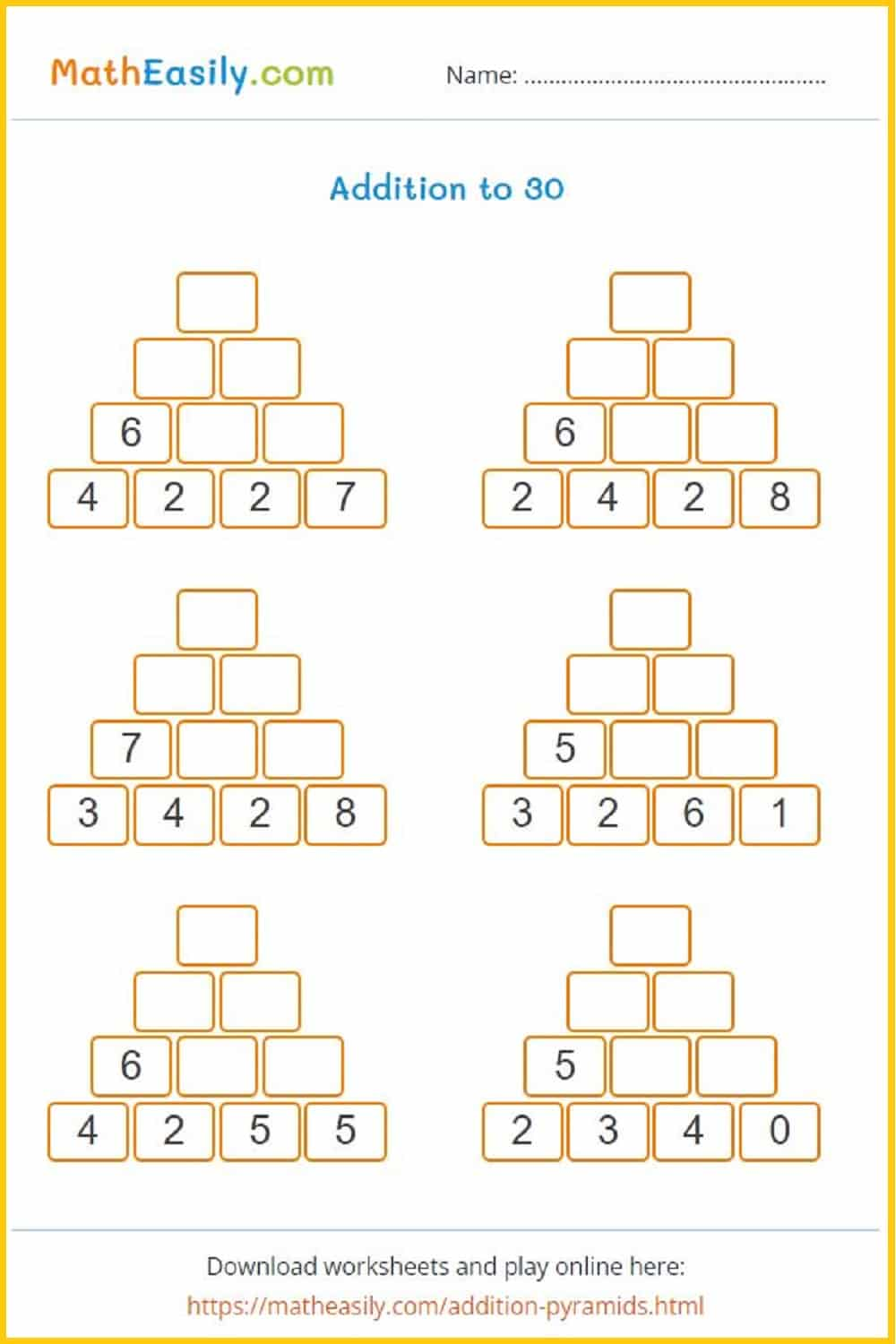Generate a new PDF

### Math pyramids

Fill in the addition pyramid puzzles up to 50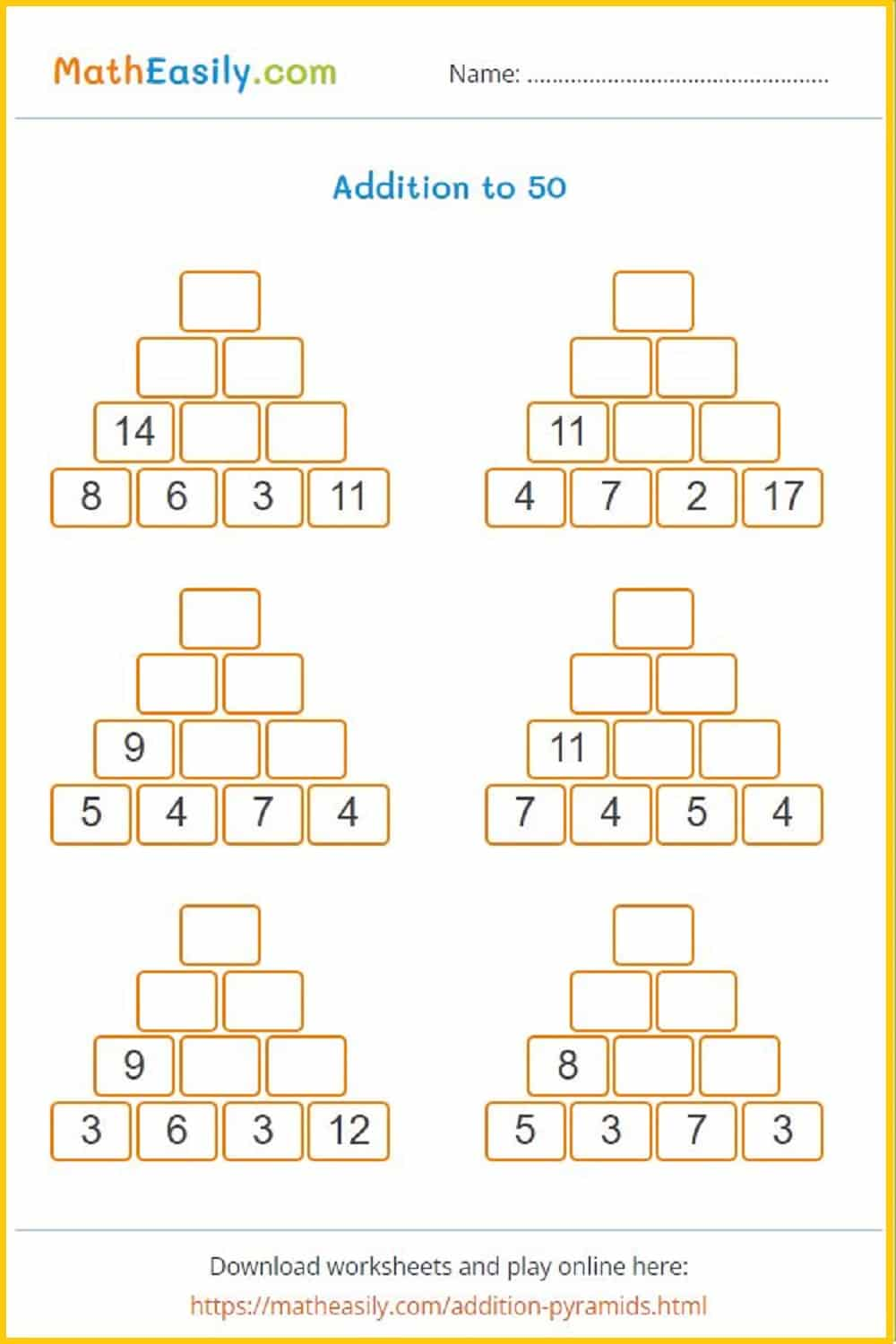Generate a new PDF

### Pyramid pyzzle

Fill in the addition pyramid puzzles up to 100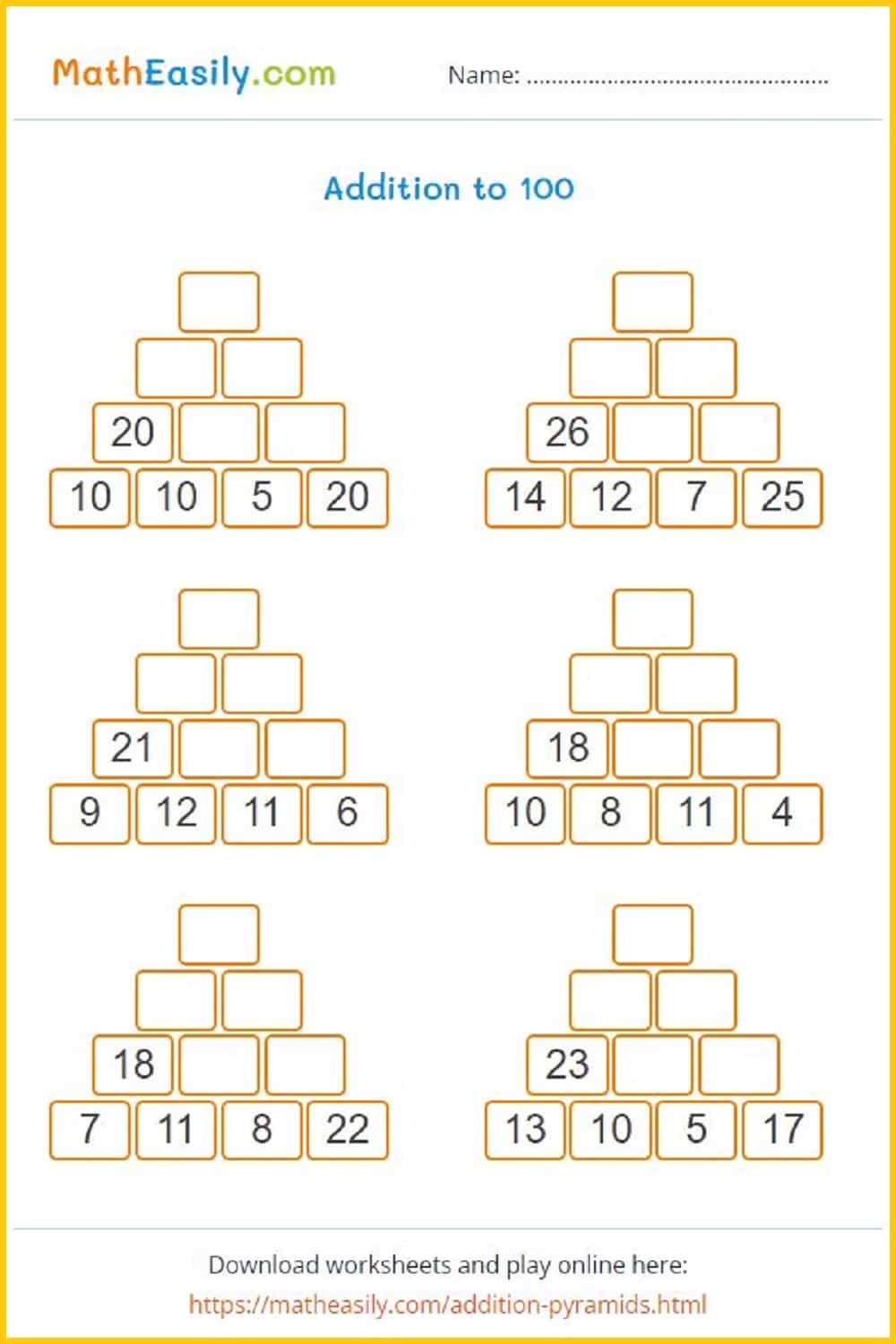Generate a new PDF

### Christmas tree

Fill in the christmas number pyramid puzzles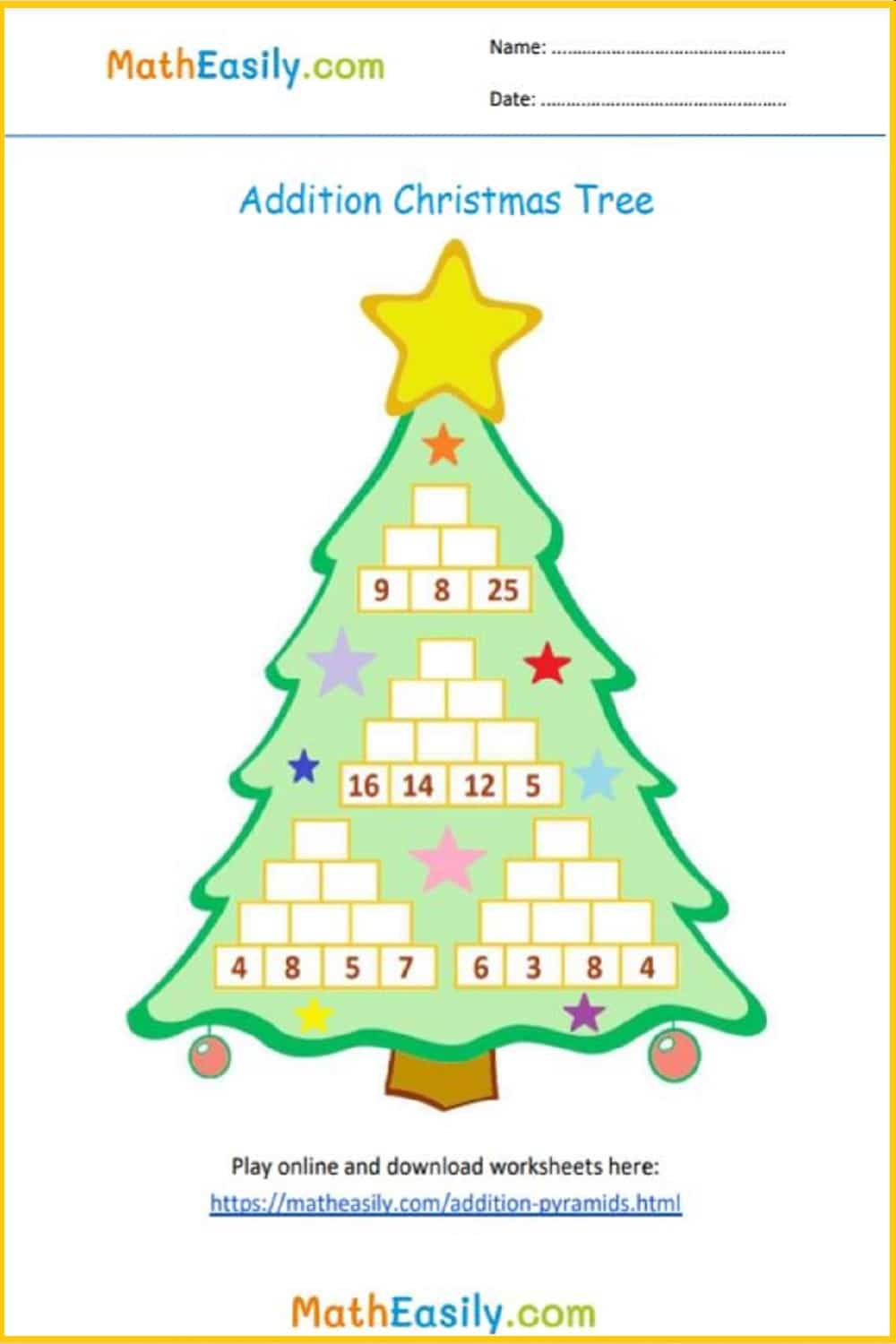### Add and subtract to 20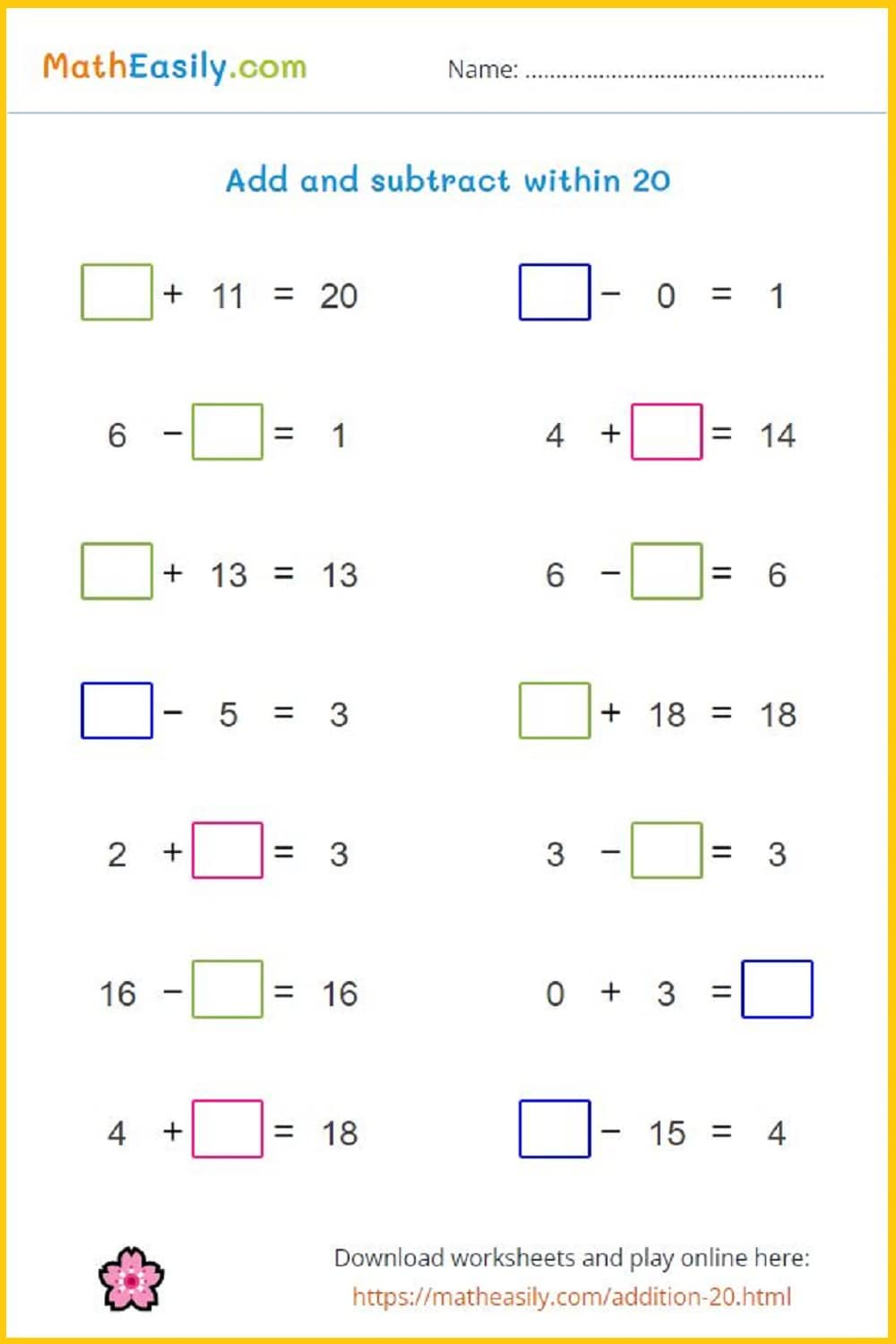Generate the new PDF

### Number bonds to 10

Generate number bonds to 10: worksheets in PDF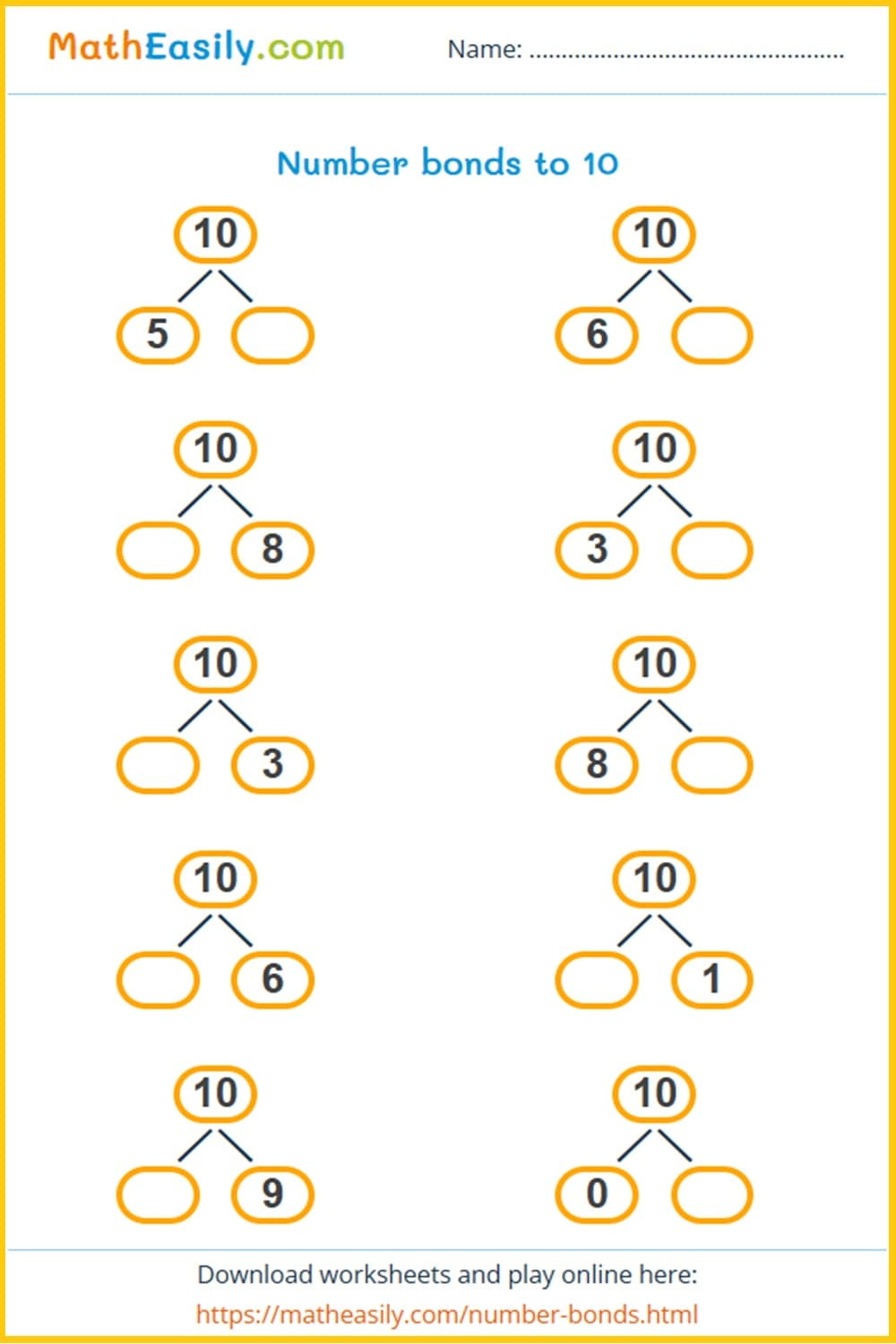Generate the new PDF

### Number bonds to 20

Generate number bonds to 20: worksheets in PDF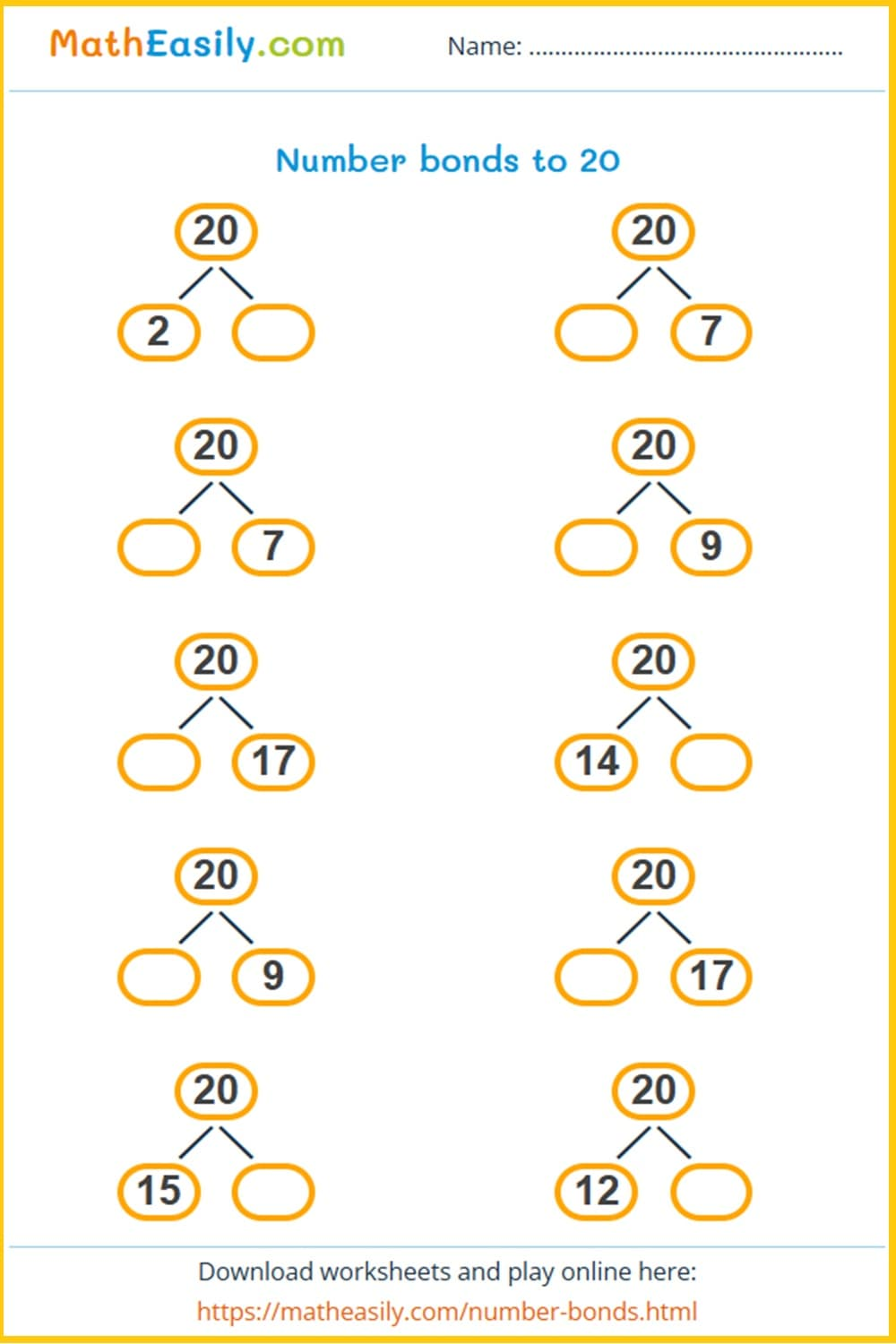Generate a new PDF

### Number bonds to 50

Generate number bonds to 50: worksheets in PDF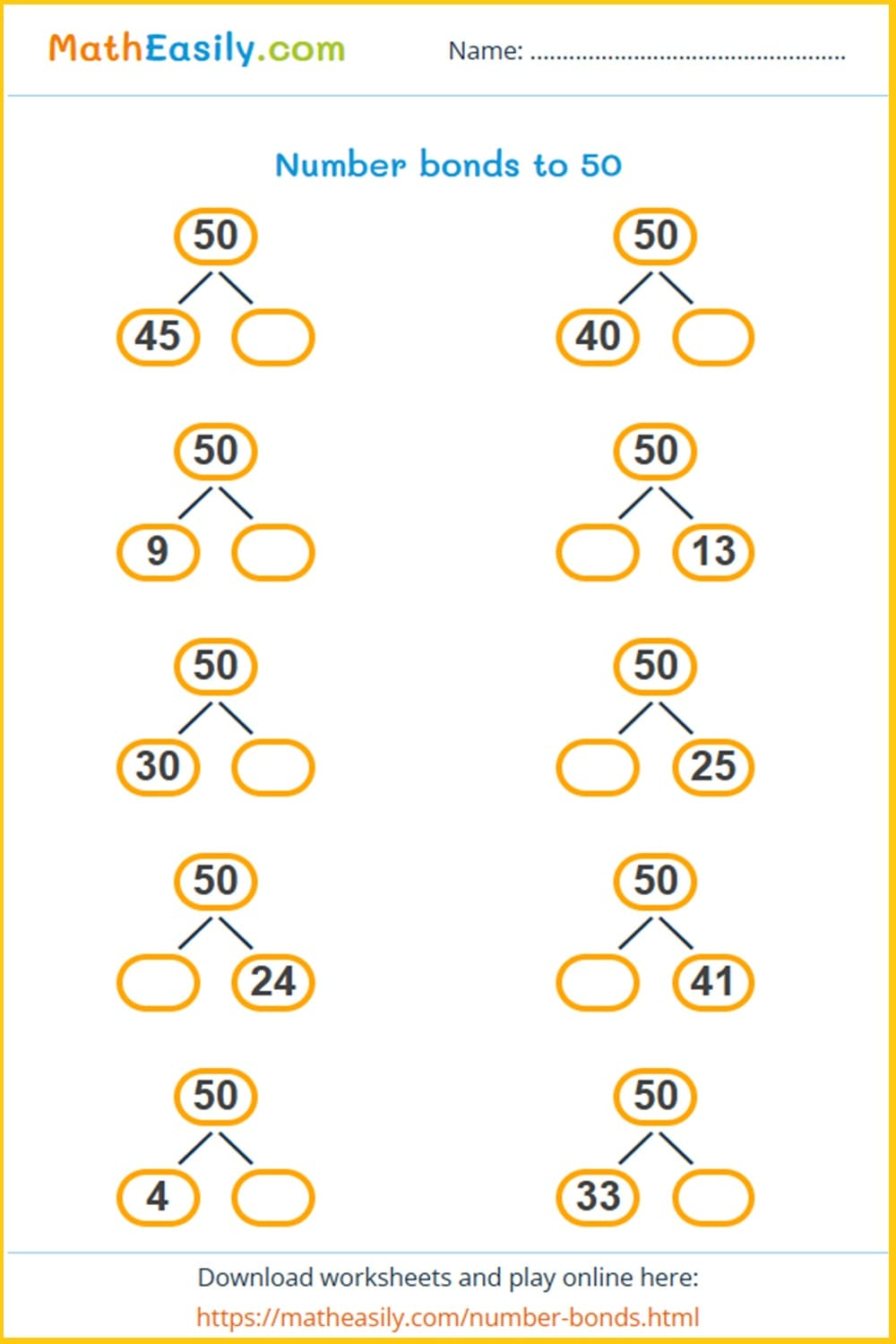Generate a new PDF

### Number bonds to 100

Generate number bonds to 100: worksheets in PDF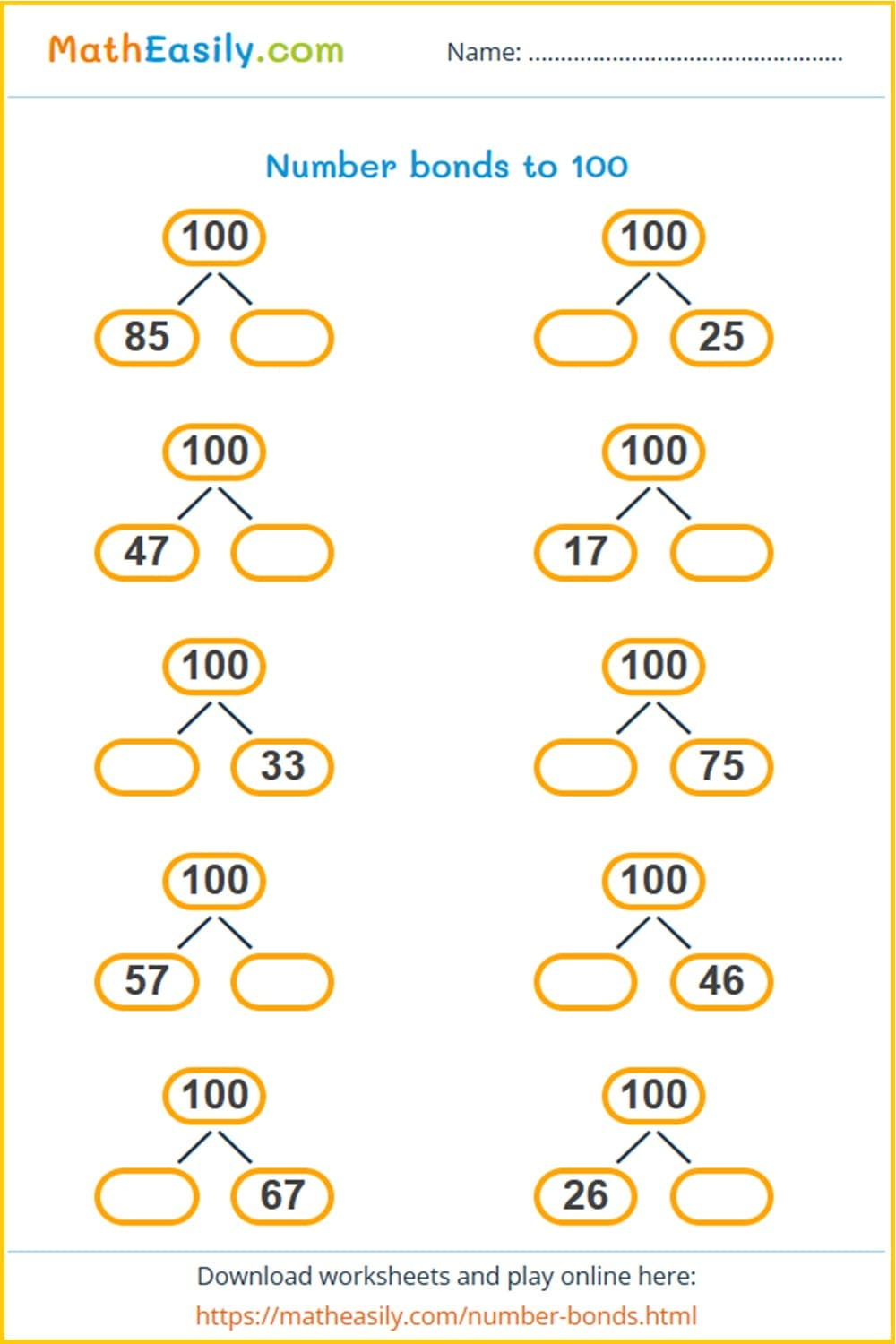Generate a new PDF

### Math picture puzzles

Solve maths picture puzzles: easy equations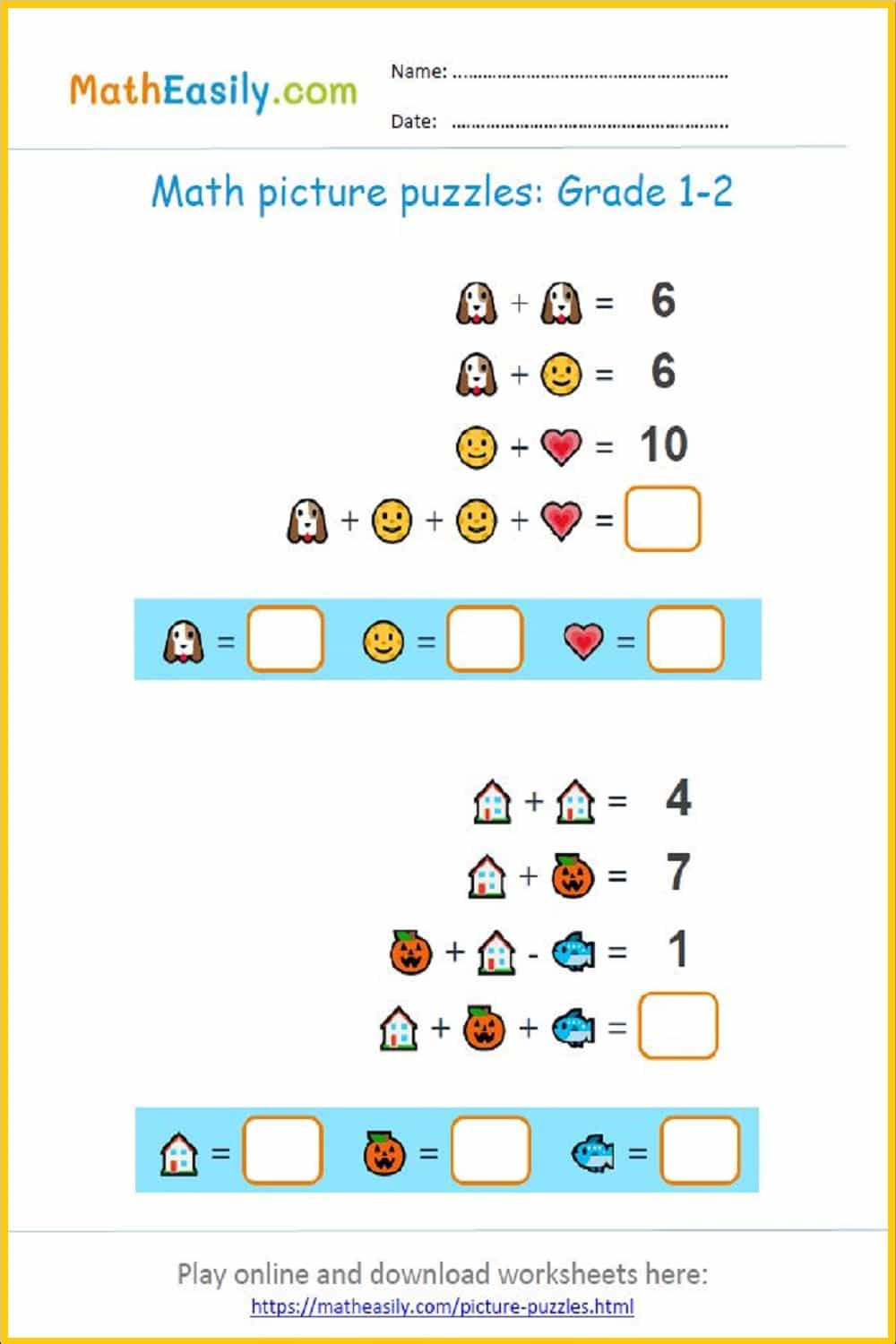Generate a new PDF

### Math crossword puzzles

Fill in the math crossword puzzles up to 20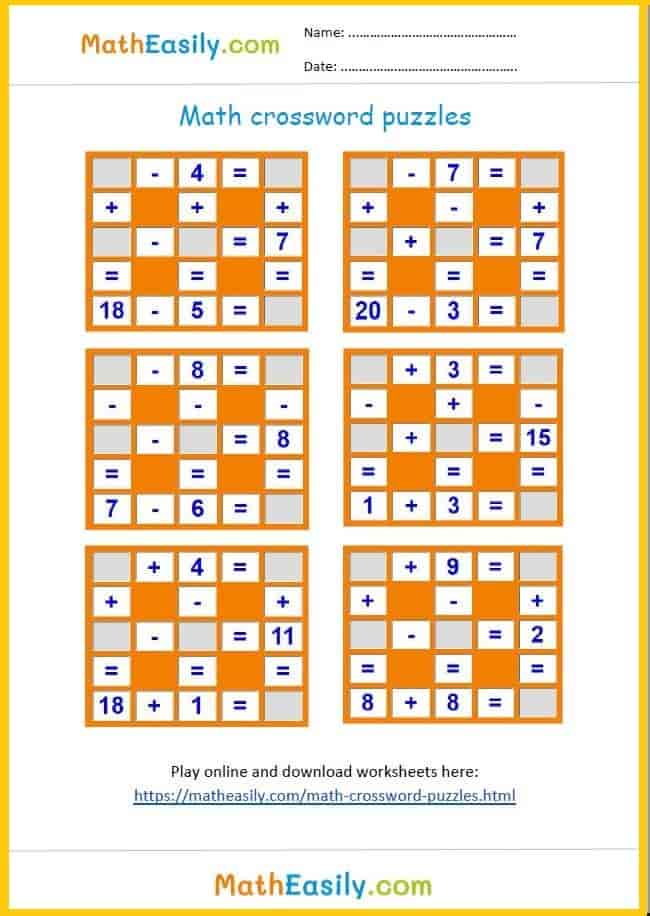Mixed addition and subtraction puzzles up to 20Mixed addition and subtraction puzzles up to 30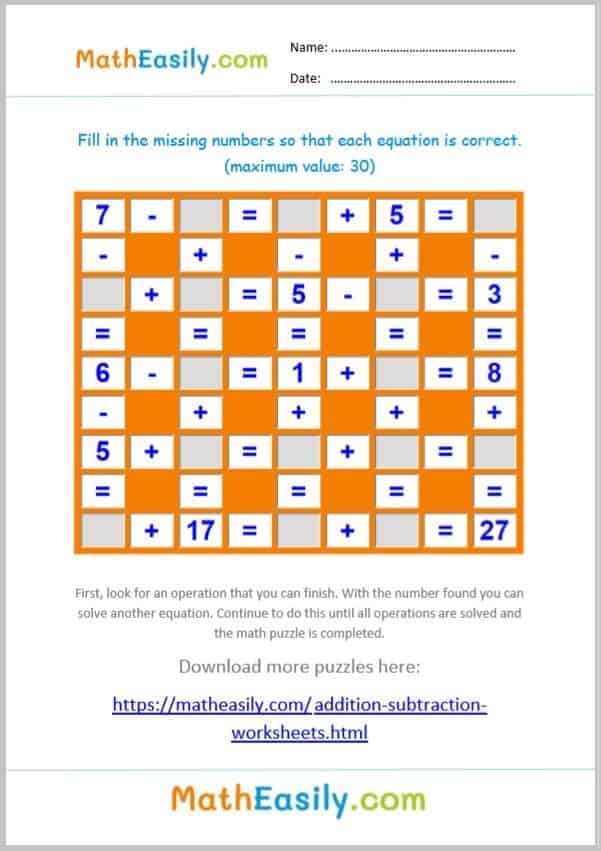Mixed addition and subtraction puzzles up to 50### Add and subtract to 100

Mixed addition and subtraction puzzles up to 100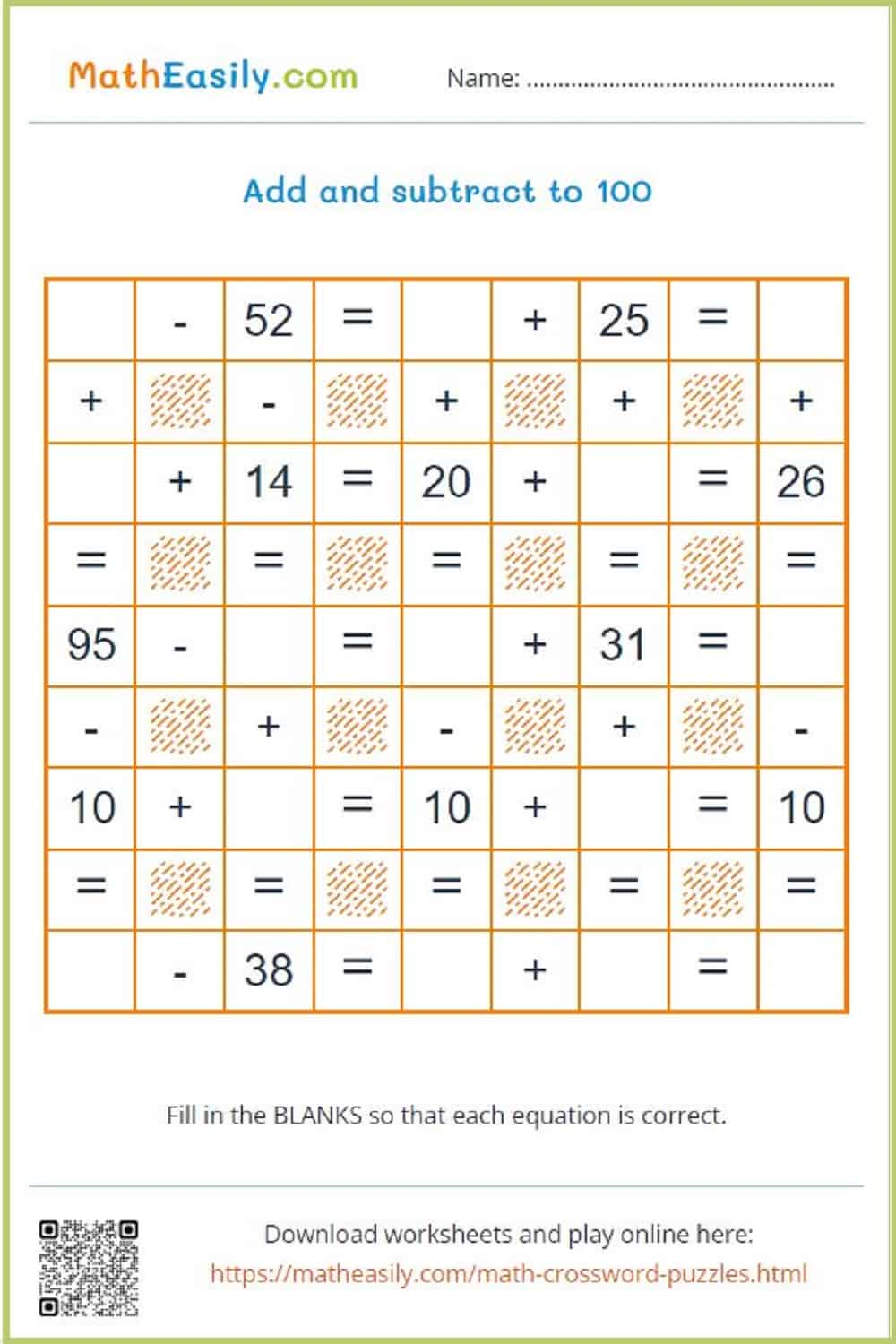Generate a new PDF

#### Multiplication games in PDF

50 multiplication puzzles

Fun printable puzzles to practice multiplication. 50 different pictures, many multiplication tasks.

US\$ 3.00

## Grade 1 math worksheets PDF: subtraction

### Single digit subtraction

Subtraction within 10 with help of pictures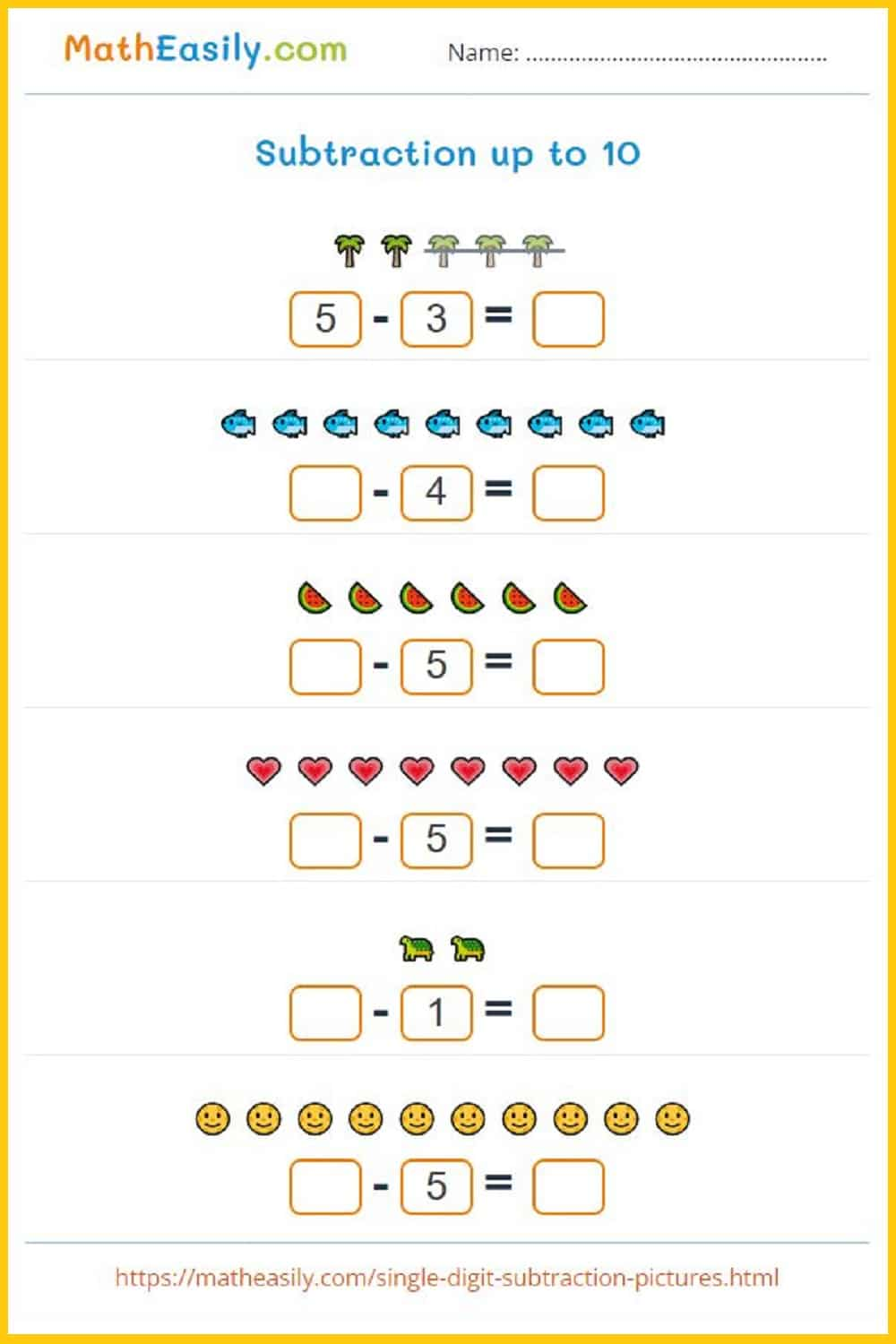Generate a new PDF

### Single digit subtraction

Subtraction within 10 worksheets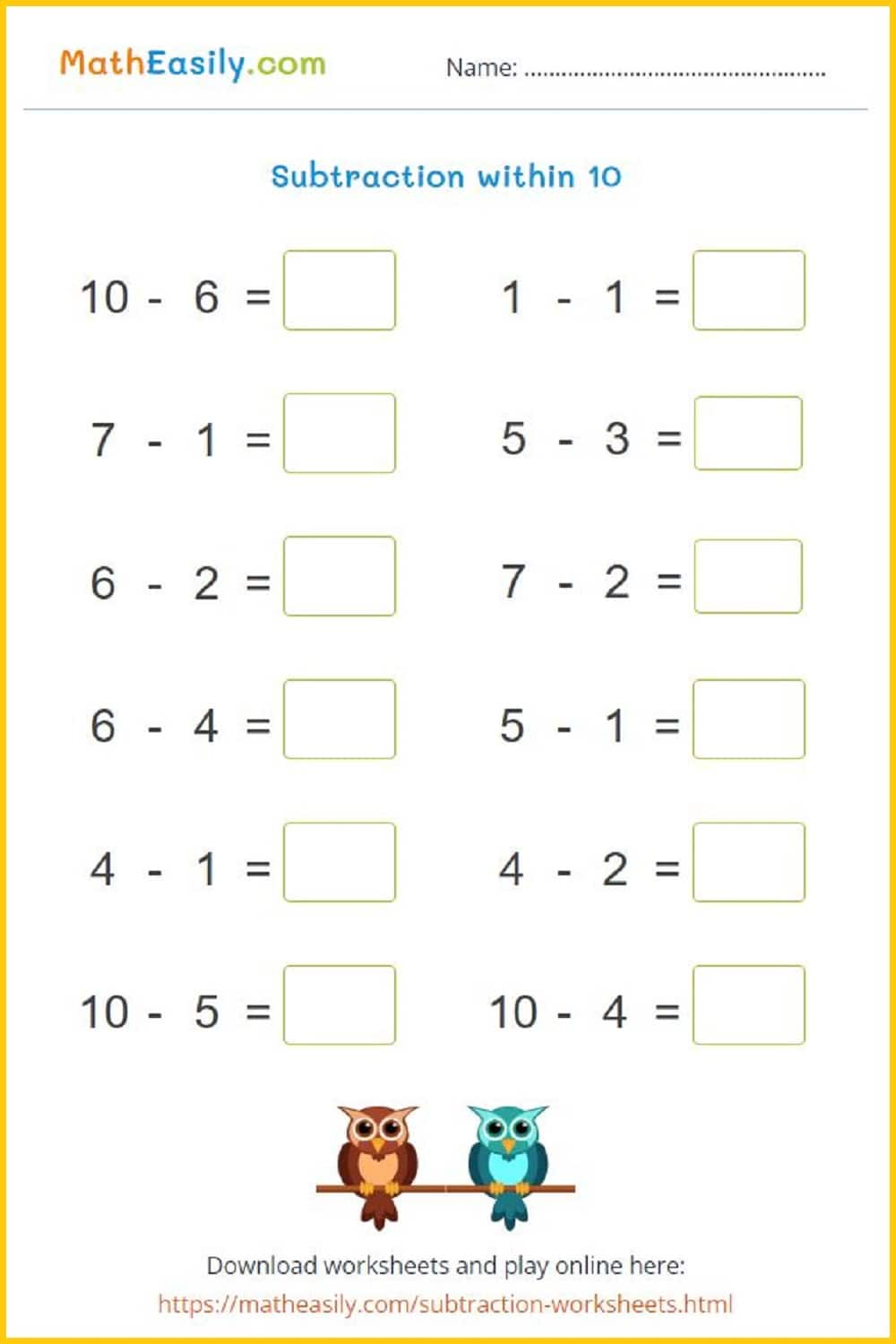Generate a new PDF

### Subtraction puzzles

Generate new subtraction puzzles, more than 50 pictures available.Generate a new PDF

### Subtraction up to 20

Subtraction within 20 with help of pictures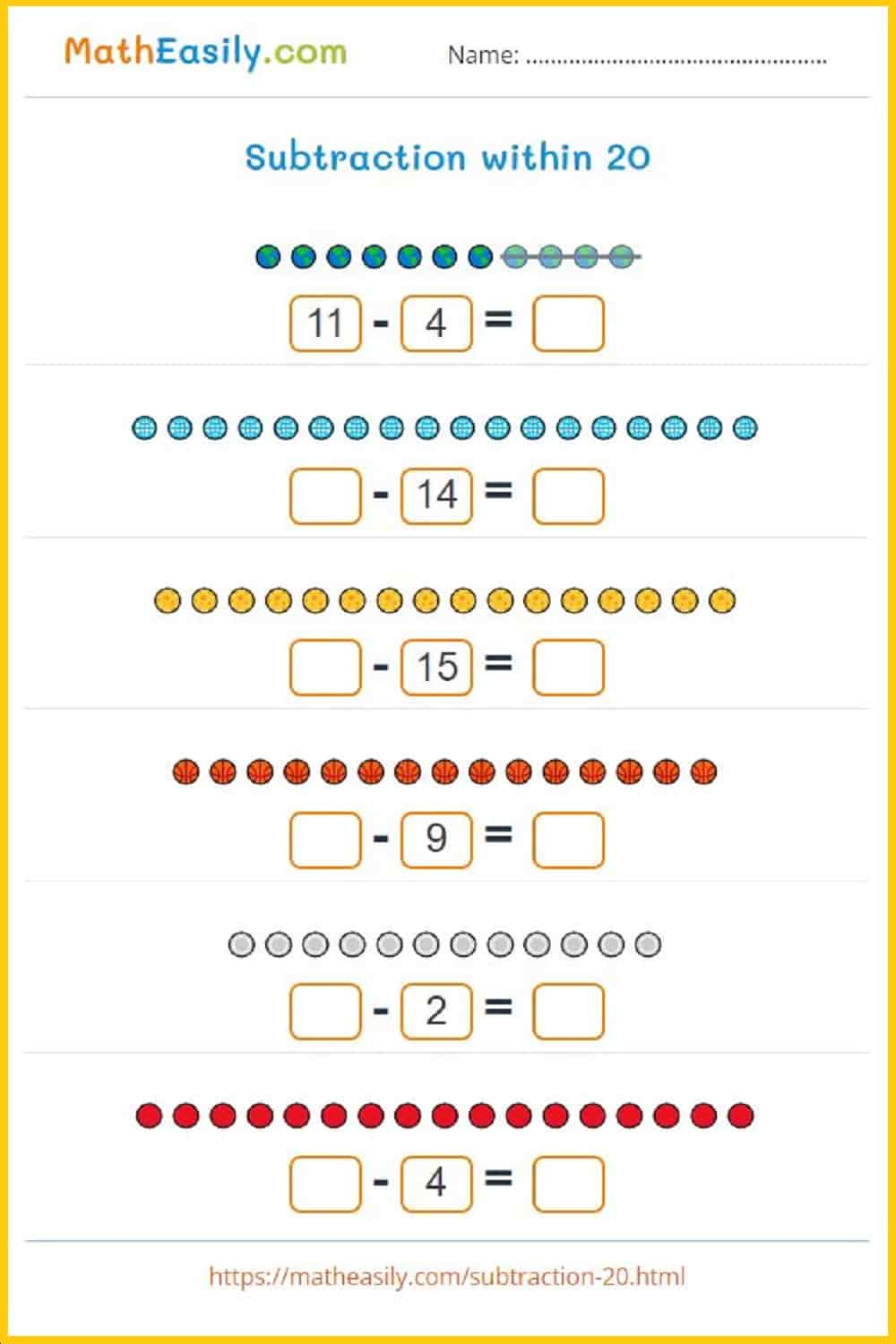Generate a new PDF

### Subtraction up to 20

Subtraction within 20: worksheets with answers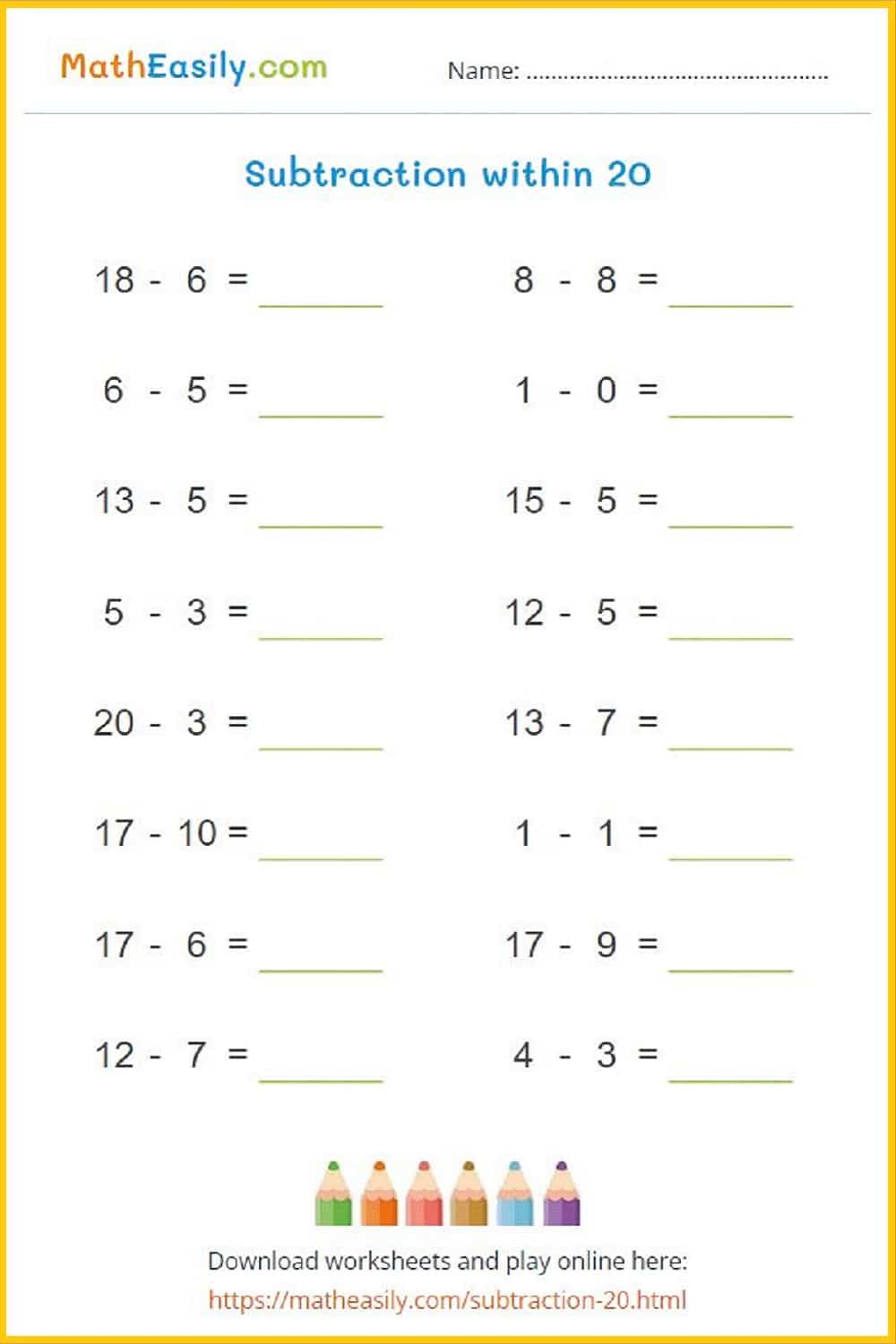Generate a new PDF

### Subtraction up to 30

Subtraction within 30: worksheets with answers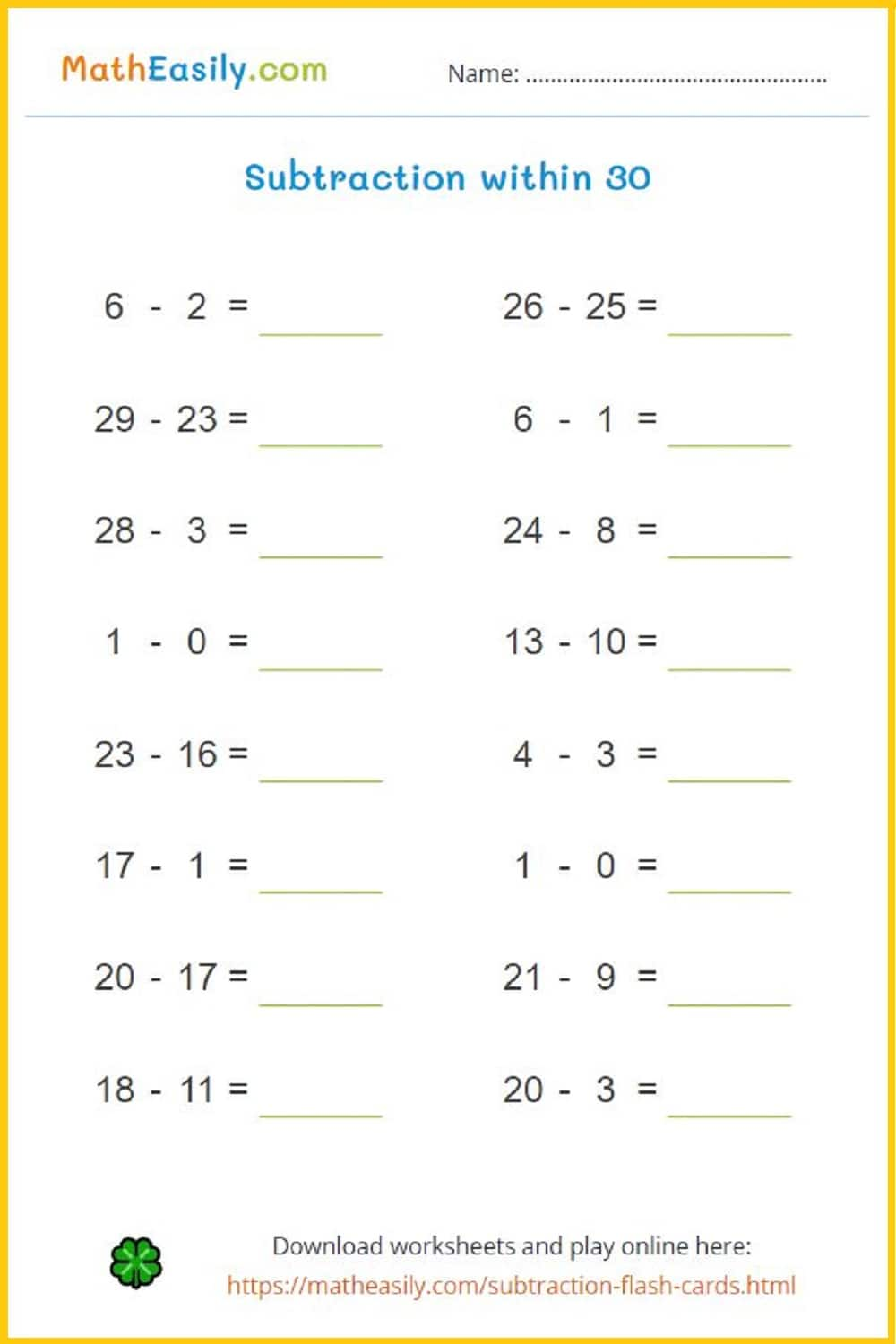Generate a new PDF

### Subtraction pyramid

Fill in subtraction pyramid puzzles up to 20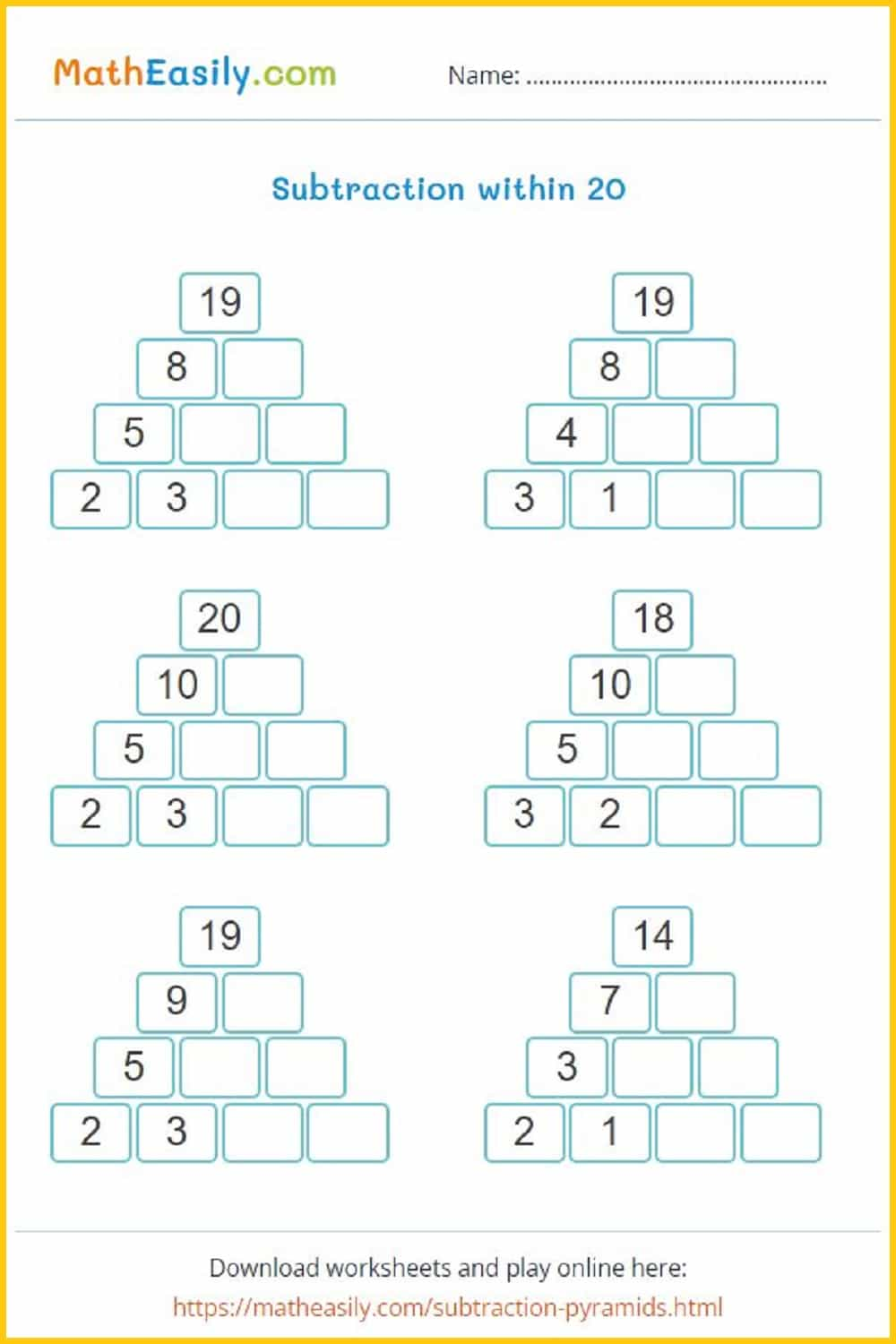Generate a new PDF

### Subtraction pyramids

Fill in subtraction pyramid puzzles up to 30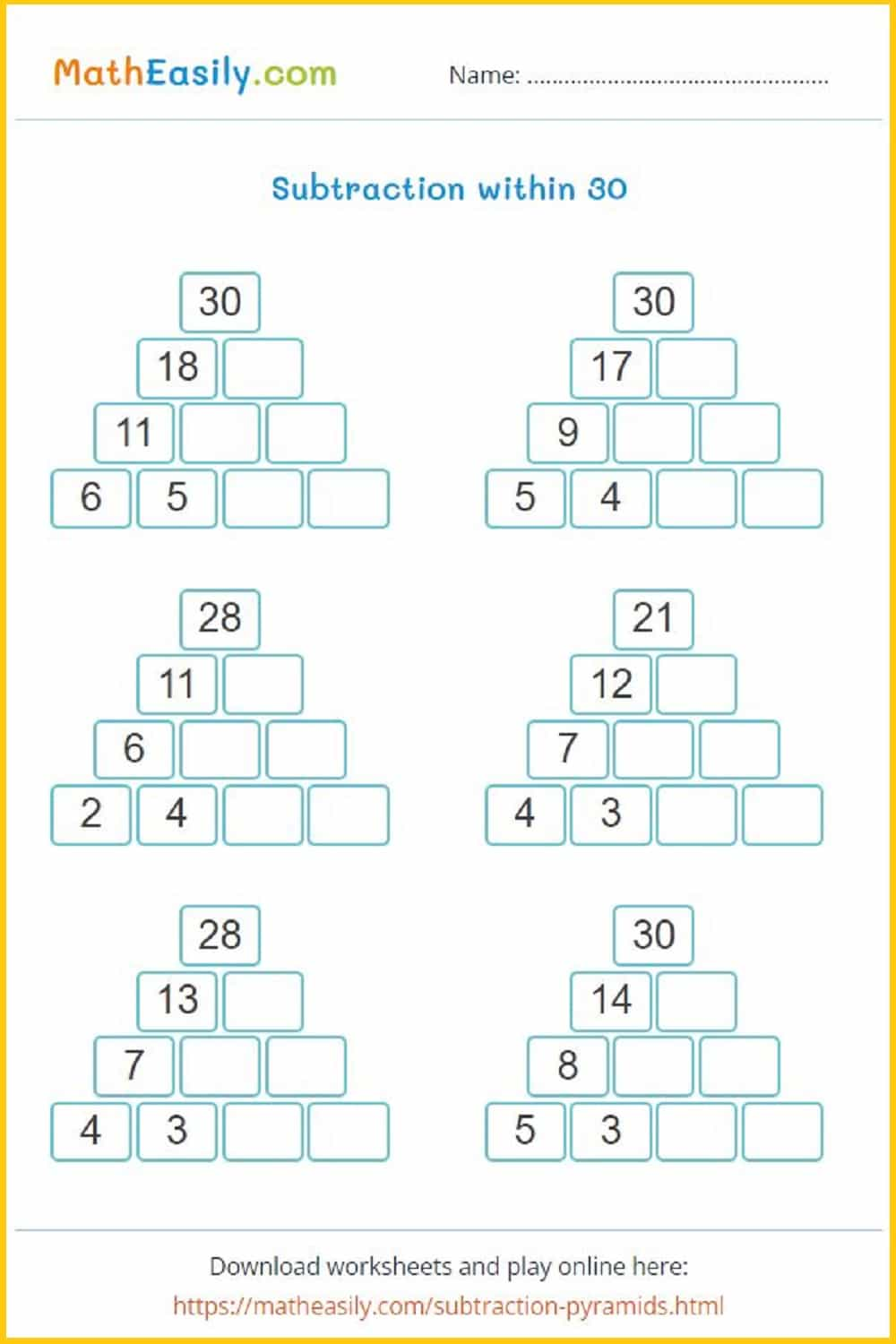Generate a new PDF

### Subtraction pyramid

Fill in subtraction pyramid puzzles up to 50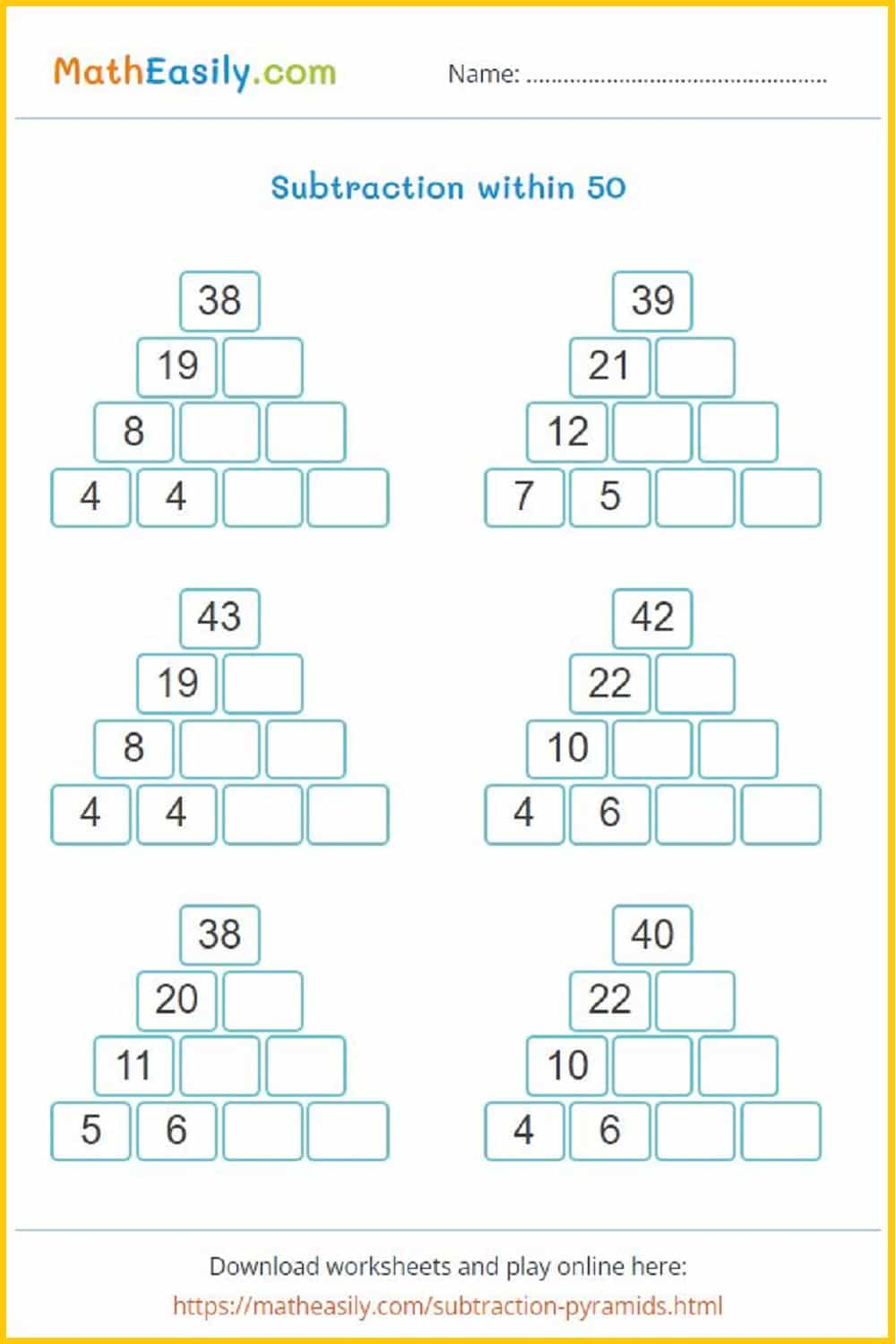Generate a new PDF

### Subtraction pyramids

Fill in subtraction pyramid puzzles up to 100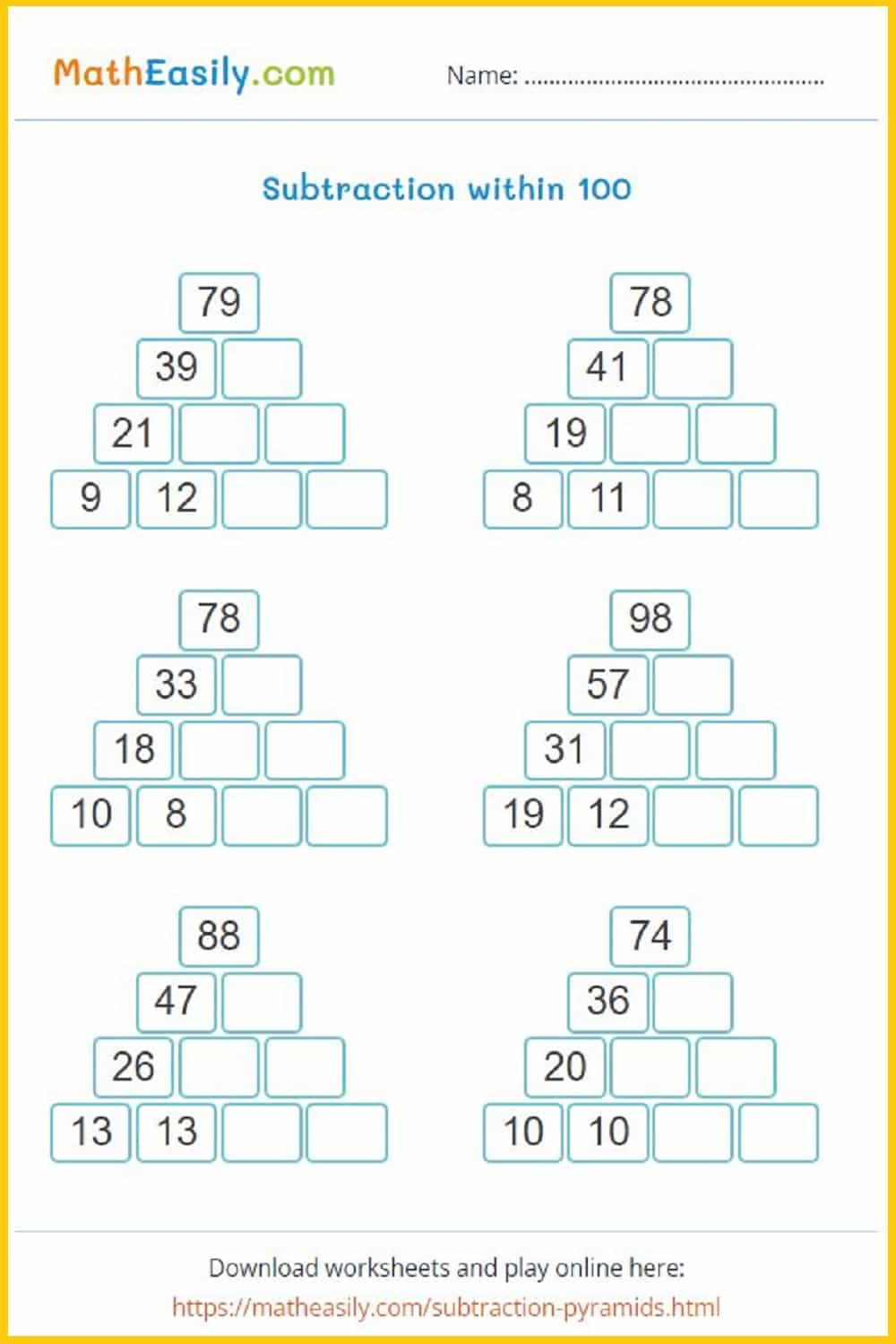Generate a new PDF

## Math worksheets for grade 1: multiplication

Our printable first grade multiplication worksheets help kids to learn multiplication. These free printable multiplication worksheets for grade 1 in PDF have been prepared to help students learn mathematics easily.

### Multiplication board game

Learning multiplication is one of the most challenging tasks in math. To help our kids to learn it easily I designed this printable multiplication board game in PDF.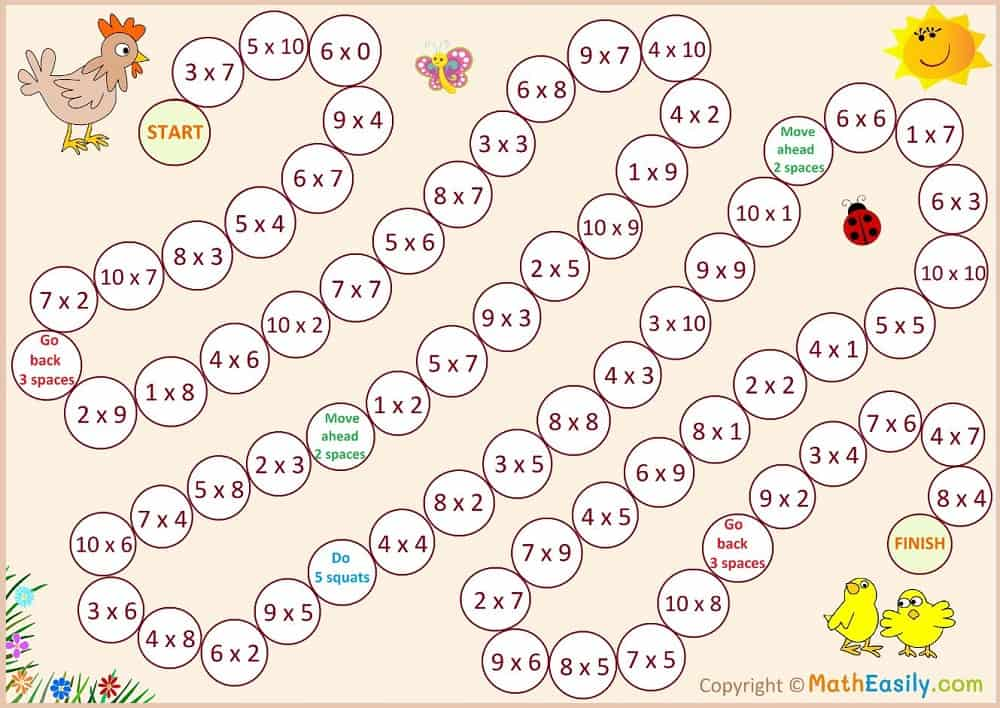### Multiplication table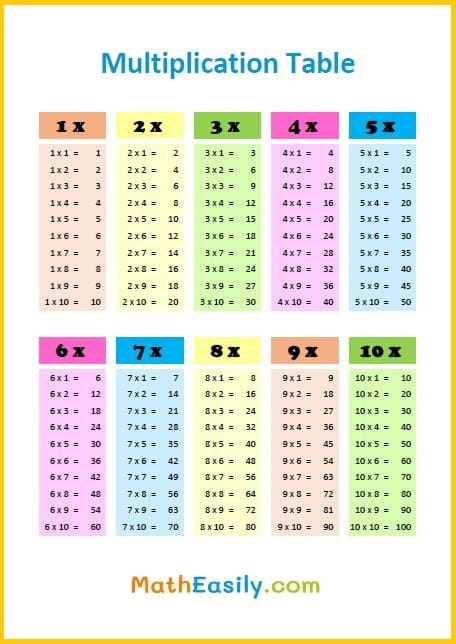### Multiplication chart

Free printable multiplication chart 1-12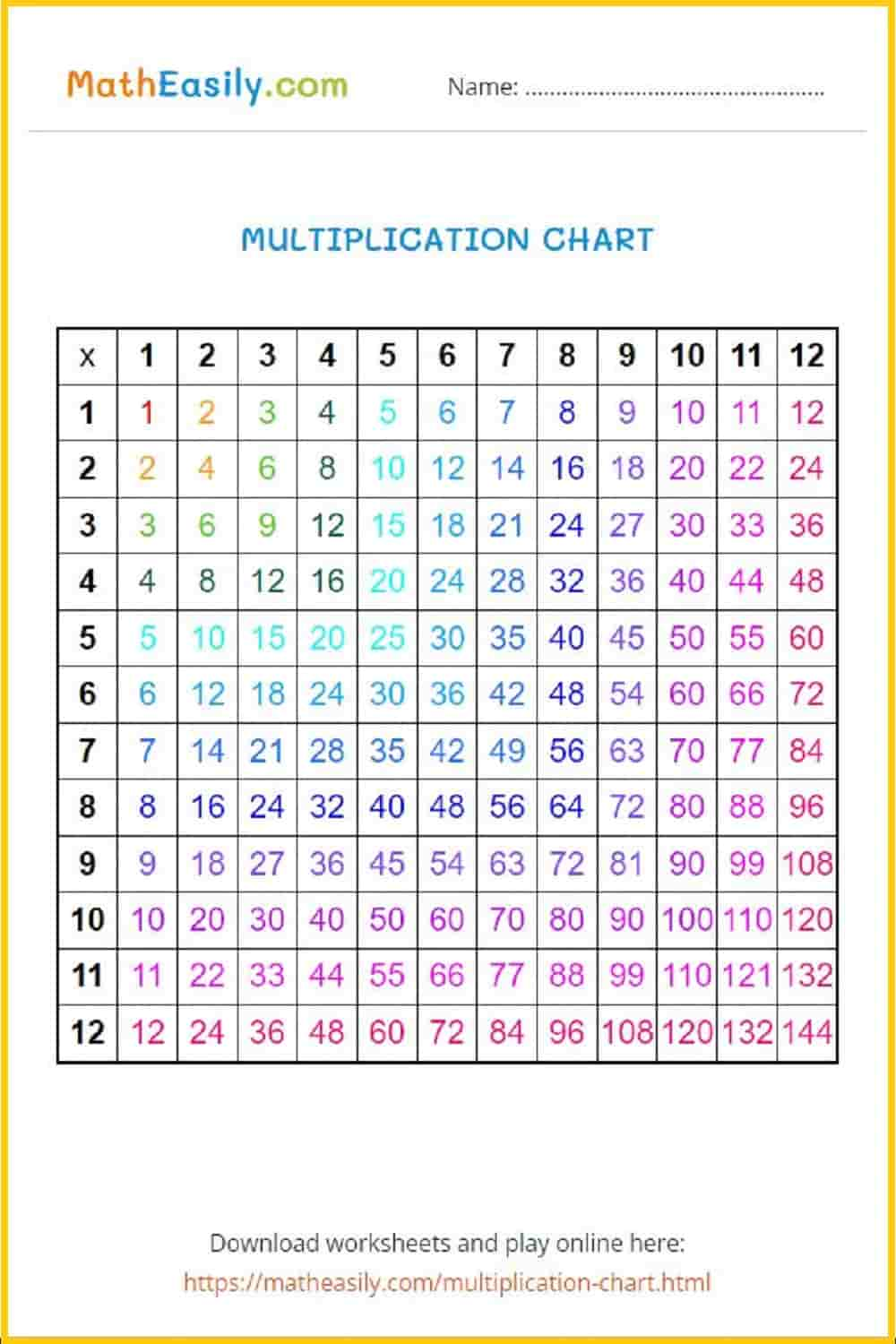### Multiplication puzzles

Generate multiplication puzzles, more than 50 pictures available.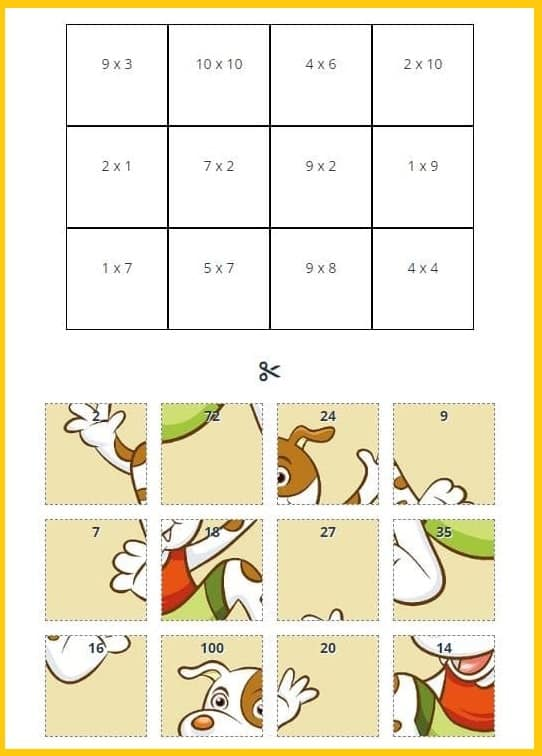Generate a new PDF

### Multiplication dice game

Play easy multiplication game with dice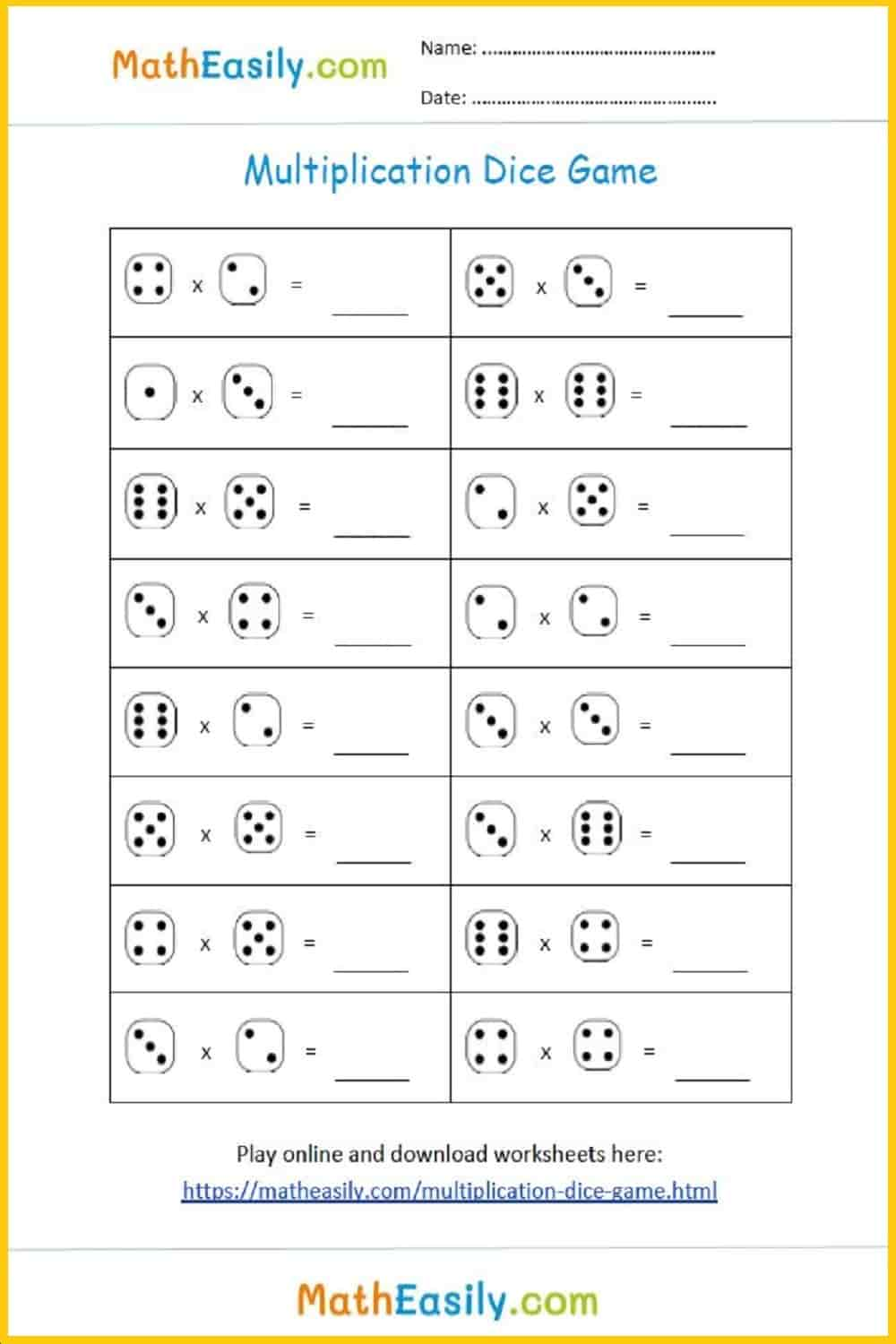### Multiplication dice game

Play easy multiplication game with dice### Single digit multiplication

Practice single digit multiplication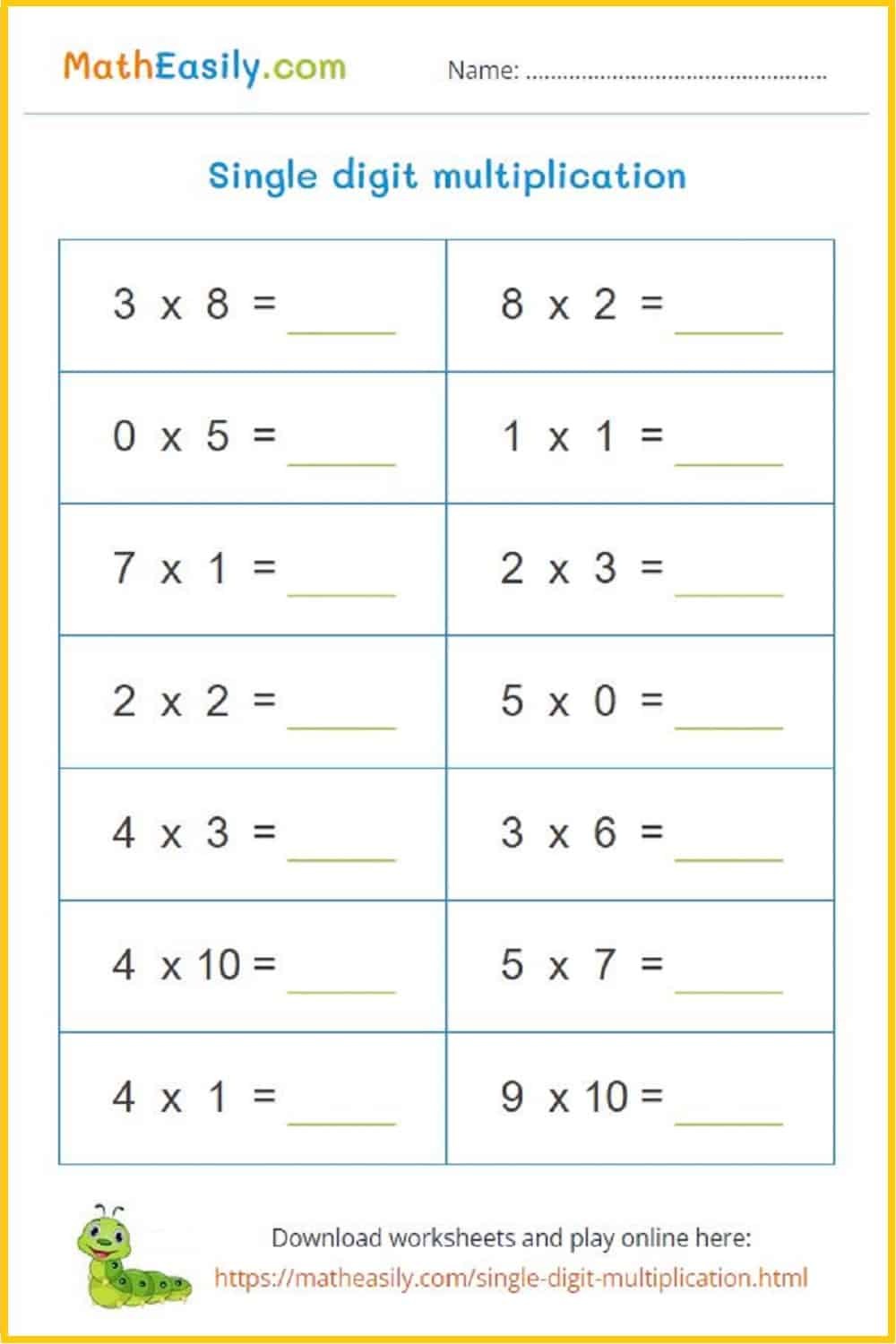Generate a new PDF

### Multiplication pyramids

Multiplication pyramids to 100: worksheets with answersGenerate a new PDF

### Multiplication pyramids

Multiplication pyramids to 300: worksheets with answers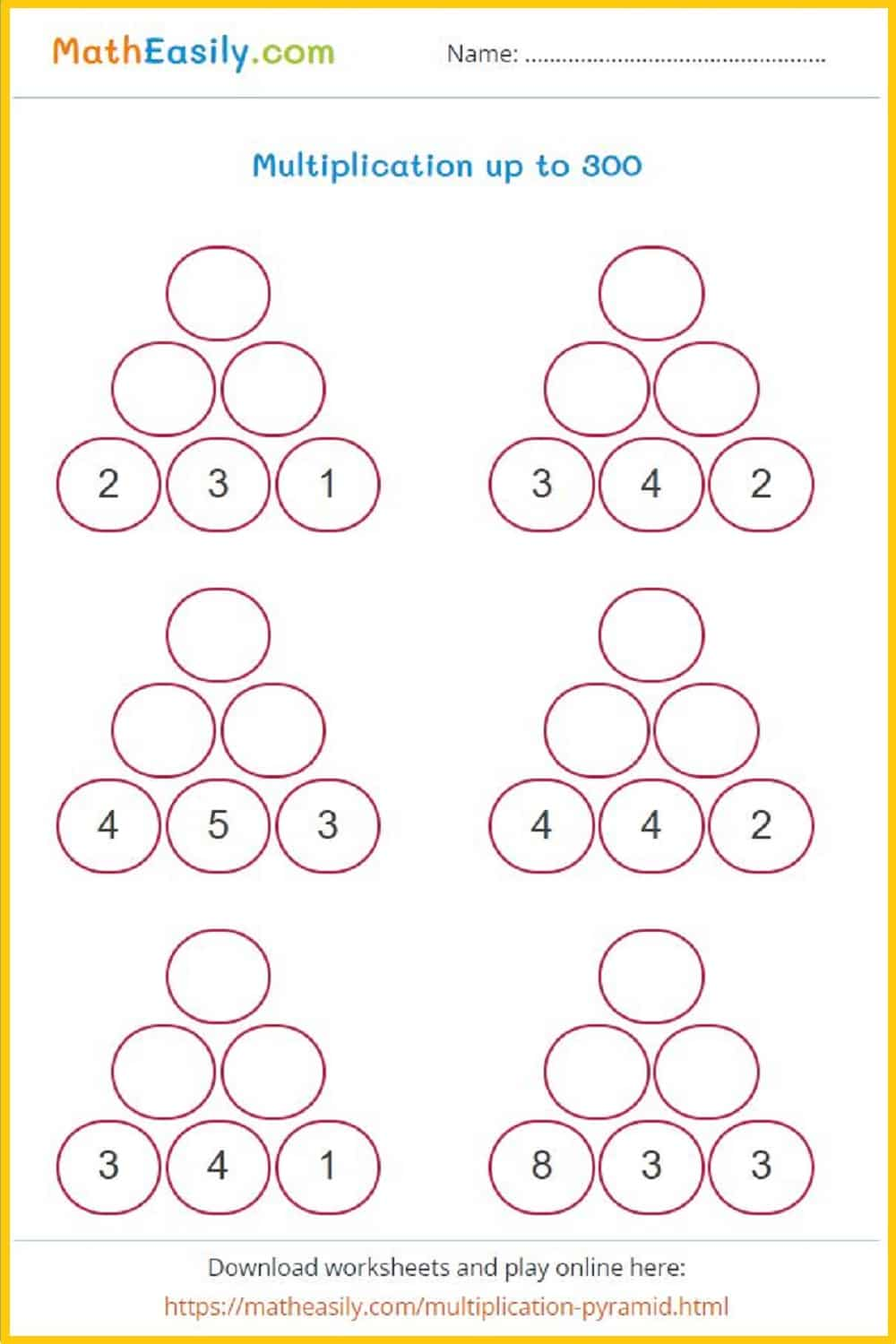Generate a new PDF

### Multiplication comparison

Multiply and compare the products: worksheets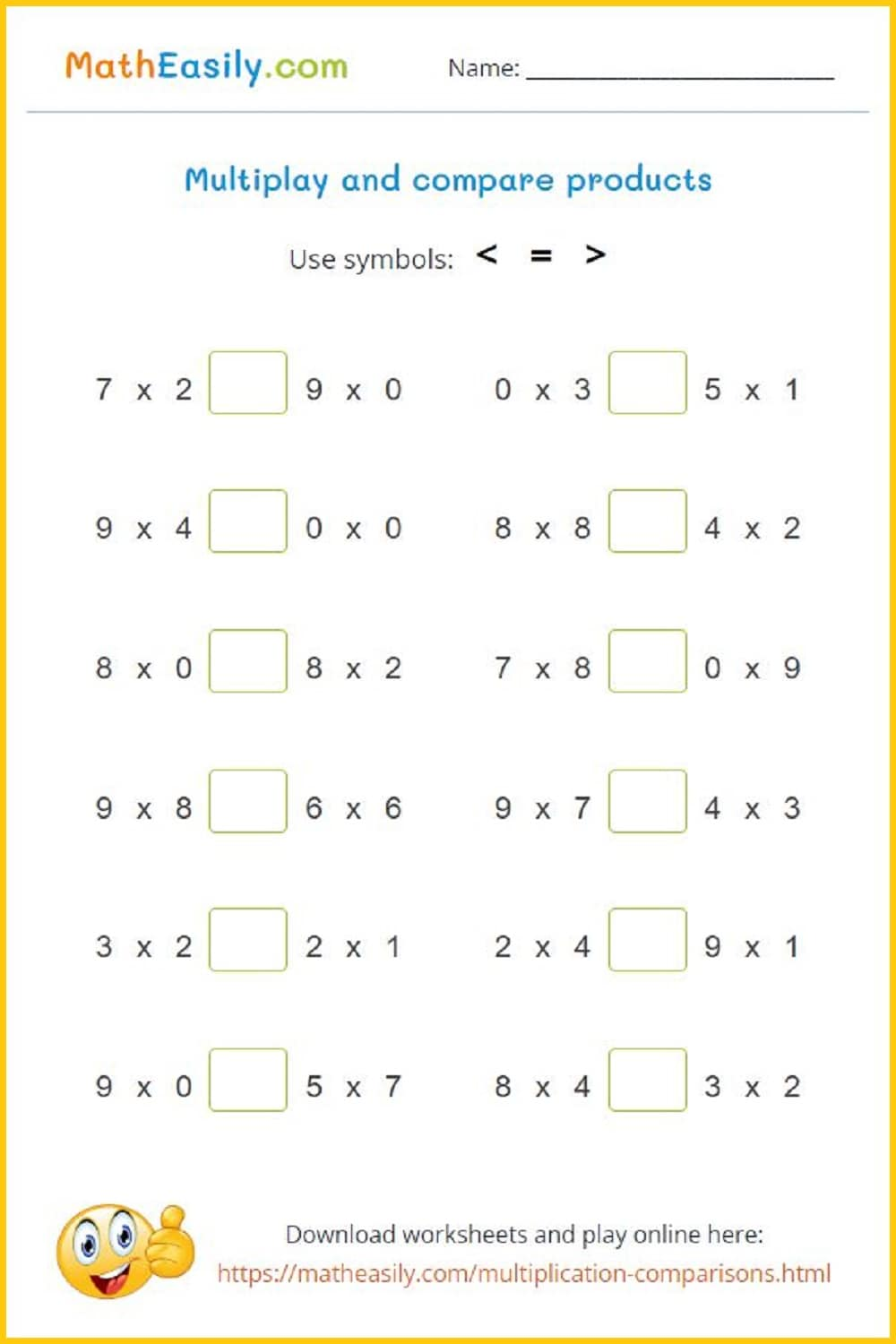Generate a new PDF

## Free first grade math worksheets

Our free 1st grade math worksheets are PDF documents which you can easily download. Just click on picture of the grade 1 math worksheet and download the PDF. Each of these printable math 1st grade worksheets contains also a link to the online game where kids can practice a given math skill.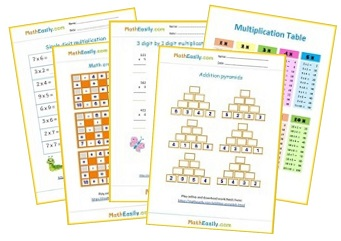Free Math Worksheets

## Other math worksheets

• Kindergarten Math Worksheets